# US8690073B2 - Control of flow rate and thermal conditions using two-layered thin films separated by flexible seals and rotatable pivot - Google Patents

Control of flow rate and thermal conditions using two-layered thin films separated by flexible seals and rotatable pivot Download PDF

## Info

Publication number
US8690073B2
US8690073B2 US13/032,049 US201113032049A US8690073B2 US 8690073 B2 US8690073 B2 US 8690073B2 US 201113032049 A US201113032049 A US 201113032049A US 8690073 B2 US8690073 B2 US 8690073B2
Authority
US
United States
Prior art keywords
substrate
fluid
dimensionless
thermal
flexible
Prior art date
Legal status (The legal status is an assumption and is not a legal conclusion. Google has not performed a legal analysis and makes no representation as to the accuracy of the status listed.)
Expired - Fee Related, expires
Application number
US13/032,049
Other versions
US20110165369A1 (en
Inventor
Kambiz Vafai
Current Assignee (The listed assignees may be inaccurate. Google has not performed a legal analysis and makes no representation or warranty as to the accuracy of the list.)
Kambix Innovations LLC
Original Assignee
Kambix Innovations LLC
Priority date (The priority date is an assumption and is not a legal conclusion. Google has not performed a legal analysis and makes no representation as to the accuracy of the date listed.)
Filing date
Publication date
Priority to US47085003P priority Critical
Priority to US10/840,303 priority patent/US7654468B2/en
Priority to US11/184,932 priority patent/US7770809B2/en
Priority to US12/793,826 priority patent/US8172156B2/en
Application filed by Kambix Innovations LLC filed Critical Kambix Innovations LLC
Priority to US13/032,049 priority patent/US8690073B2/en
Assigned to KAMBIX INNOVATIONS, LLC reassignment KAMBIX INNOVATIONS, LLC ASSIGNMENT OF ASSIGNORS INTEREST (SEE DOCUMENT FOR DETAILS). Assignors: KHALED, ABDUL RAHIM A., VAFAI, KAMBIZ
Publication of US20110165369A1 publication Critical patent/US20110165369A1/en
Application granted granted Critical
Publication of US8690073B2 publication Critical patent/US8690073B2/en
Expired - Fee Related legal-status Critical Current

• 239000010409 thin films Substances 0.000 title claims abstract description 277
• 239000000758 substrates Substances 0.000 claims description 409
• 239000010410 layers Substances 0.000 abstract description 253
• 238000007789 sealing Methods 0.000 abstract description 15
• 230000000694 effects Effects 0.000 description 198
• 238000001816 cooling Methods 0.000 description 78
• 239000006185 dispersions Substances 0.000 description 73
• 239000002826 coolants Substances 0.000 description 50
• 239000002529 flux Substances 0.000 description 46
• 230000004907 flux Effects 0.000 description 46
• 230000001603 reducing Effects 0.000 description 38
• 239000002105 nanoparticles Substances 0.000 description 36
• 238000009826 distribution Methods 0.000 description 35
• 238000006722 reduction reactions Methods 0.000 description 32
• 239000000203 mixtures Substances 0.000 description 30
• 238000000034 methods Methods 0.000 description 29
• 239000011882 ultra-fine particles Substances 0.000 description 28
• 238000002485 combustion reactions Methods 0.000 description 27
• 239000010408 films Substances 0.000 description 25
• 239000003570 air Substances 0.000 description 24
• 238000010438 heat treatment Methods 0.000 description 23
• 239000000463 materials Substances 0.000 description 23
• 239000004793 Polystyrene Substances 0.000 description 22
• 230000000875 corresponding Effects 0.000 description 21
• 230000001965 increased Effects 0.000 description 20
• 239000002245 particles Substances 0.000 description 19
• 239000000725 suspensions Substances 0.000 description 19
• 230000000712 assembly Effects 0.000 description 18
• 230000001276 controlling effects Effects 0.000 description 18
• 239000010949 copper Substances 0.000 description 18
• 229910052802 copper Inorganic materials 0.000 description 18
• 238000010586 diagrams Methods 0.000 description 17
• 230000000051 modifying Effects 0.000 description 17
• 239000000243 solutions Substances 0.000 description 17
• 230000002708 enhancing Effects 0.000 description 16
• 239000007789 gases Substances 0.000 description 16
• 239000000446 fuels Substances 0.000 description 15
• 230000003534 oscillatory Effects 0.000 description 14
• 238000006073 displacement reactions Methods 0.000 description 13
• 238000004458 analytical methods Methods 0.000 description 12
• 229910052724 xenon Inorganic materials 0.000 description 11
• 239000007788 liquids Substances 0.000 description 10
• 239000002356 single layers Substances 0.000 description 10
• 239000011901 water Substances 0.000 description 10
• 229910052734 helium Inorganic materials 0.000 description 9
• 239000001307 helium Substances 0.000 description 9
• 238000007906 compression Methods 0.000 description 8
• 229920001169 thermoplastics Polymers 0.000 description 8
• 239000004416 thermosoftening plastic Substances 0.000 description 8
• 230000001131 transforming Effects 0.000 description 8
• 230000027455 binding Effects 0.000 description 7
• 230000003247 decreasing Effects 0.000 description 7
• 238000009472 formulation Methods 0.000 description 7
• 239000000126 substances Substances 0.000 description 7
• 238000002844 melting Methods 0.000 description 6
• 230000035882 stress Effects 0.000 description 6
• 230000001052 transient Effects 0.000 description 6
• 229910000906 Bronze Inorganic materials 0.000 description 5
• 239000010974 bronze Substances 0.000 description 5
• 239000003795 chemical substances by application Substances 0.000 description 5
• 238000004100 electronic packaging Methods 0.000 description 5
• 229910052751 metals Inorganic materials 0.000 description 5
• 239000002184 metals Substances 0.000 description 5
• 230000000737 periodic Effects 0.000 description 5
• 229910052700 potassium Inorganic materials 0.000 description 5
• 229910052720 vanadium Inorganic materials 0.000 description 5
• 229910052782 aluminium Inorganic materials 0.000 description 4
• 238000009413 insulation Methods 0.000 description 4
• 238000002156 mixing Methods 0.000 description 4
• 239000002071 nanotubes Substances 0.000 description 4
• 238000000926 separation method Methods 0.000 description 4
• 238000000844 transformation Methods 0.000 description 4
• 229910052727 yttrium Inorganic materials 0.000 description 4
• 281000117604 Prentice Hall companies 0.000 description 3
• 229910052770 Uranium Inorganic materials 0.000 description 3
• 238000006243 chemical reactions Methods 0.000 description 3
• 230000002596 correlated Effects 0.000 description 3
• 238000005259 measurements Methods 0.000 description 3
• 238000005086 pumping Methods 0.000 description 3
• 230000005514 two-phase flow Effects 0.000 description 3
• 229920001821 Foam rubber Polymers 0.000 description 2
• 101710072640 Prostate-Specific Antigen Proteins 0.000 description 2
• 102100011339 Prostate-specific antigen Human genes 0.000 description 2
• 239000000427 antigens Substances 0.000 description 2
• 238000005537 brownian motion Methods 0.000 description 2
• 239000011852 carbon nanoparticles Substances 0.000 description 2
• 230000000739 chaotic Effects 0.000 description 2
• 239000004020 conductors Substances 0.000 description 2
• 229920003013 deoxyribonucleic acids Polymers 0.000 description 2
• 230000018109 developmental process Effects 0.000 description 2
• 239000004811 fluoropolymers Substances 0.000 description 2
• 229920002313 fluoropolymers Polymers 0.000 description 2
• 239000006260 foams Substances 0.000 description 2
• 229910052737 gold Inorganic materials 0.000 description 2
• 239000010931 gold Substances 0.000 description 2
• 238000005461 lubrication Methods 0.000 description 2
• -1 polytetrafluoroethylene Polymers 0.000 description 2
• 239000004810 polytetrafluoroethylene Substances 0.000 description 2
• 229920001343 polytetrafluoroethylenes Polymers 0.000 description 2
• 239000007787 solids Substances 0.000 description 2
• 230000003068 static Effects 0.000 description 2
• 238000009827 uniform distribution Methods 0.000 description 2
• 280000011469 Biological Detection Systems companies 0.000 description 1
• 241000978750 Havardia Species 0.000 description 1
• 240000008528 Hevea brasiliensis Species 0.000 description 1
• 280000812534 Integral Energy companies 0.000 description 1
• 229910017436 S2 Can Inorganic materials 0.000 description 1
• 102000004965 antibodies Human genes 0.000 description 1
• 108090001123 antibodies Proteins 0.000 description 1
• 239000007864 aqueous solutions Substances 0.000 description 1
• 239000004199 argon Substances 0.000 description 1
• 229910052786 argon Inorganic materials 0.000 description 1
• 230000004323 axial length Effects 0.000 description 1
• 238000005452 bending Methods 0.000 description 1
• 238000004364 calculation methods Methods 0.000 description 1
• 229910052799 carbon Inorganic materials 0.000 description 1
• 238000004891 communication Methods 0.000 description 1
• 230000000295 complement Effects 0.000 description 1
• 238000005260 corrosion Methods 0.000 description 1
• 230000001419 dependent Effects 0.000 description 1
• 238000009792 diffusion process Methods 0.000 description 1
• 230000003292 diminished Effects 0.000 description 1
• 201000010099 diseases Diseases 0.000 description 1
• 229920001971 elastomers Polymers 0.000 description 1
• 238000005516 engineering processes Methods 0.000 description 1
• 238000002474 experimental methods Methods 0.000 description 1
• 230000002349 favourable Effects 0.000 description 1
• 238000009396 hybridization Methods 0.000 description 1
• 230000003993 interaction Effects 0.000 description 1
• 230000001788 irregular Effects 0.000 description 1
• 229910052743 krypton Inorganic materials 0.000 description 1
• 230000004301 light adaptation Effects 0.000 description 1
• 229910001338 liquidmetals Inorganic materials 0.000 description 1
• 238000004519 manufacturing process Methods 0.000 description 1
• 238000004377 microelectronics Methods 0.000 description 1
• 230000004048 modification Effects 0.000 description 1
• 238000006011 modification reactions Methods 0.000 description 1
• 239000002365 multiple layers Substances 0.000 description 1
• 229920001194 natural rubbers Polymers 0.000 description 1
• 229910052754 neon Inorganic materials 0.000 description 1
• 230000000704 physical effects Effects 0.000 description 1
• 229920000642 polymers Polymers 0.000 description 1
• 238000002360 preparation methods Methods 0.000 description 1
• 230000002940 repellent Effects 0.000 description 1
• 101710010674 rpoE Proteins 0.000 description 1
• 150000003839 salts Chemical class 0.000 description 1
• 239000003566 sealing materials Substances 0.000 description 1
• 239000002002 slurries Substances 0.000 description 1
• 239000011780 sodium chloride Substances 0.000 description 1
• 241000894007 species Species 0.000 description 1
• 230000003746 surface roughness Effects 0.000 description 1
• 239000010936 titanium Substances 0.000 description 1
• 238000004642 transportation engineering Methods 0.000 description 1

## Images

•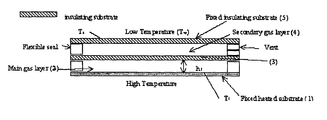•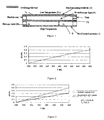•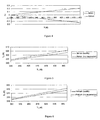•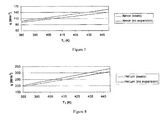•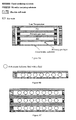•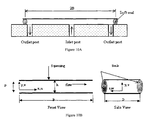•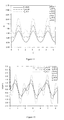•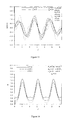•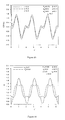•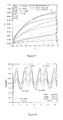•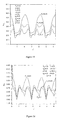•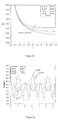••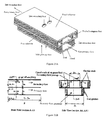•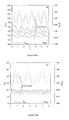•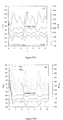•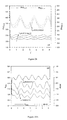•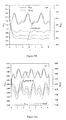•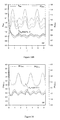•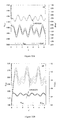••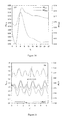•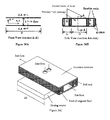•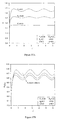•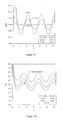•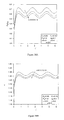•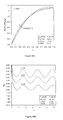•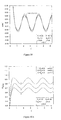••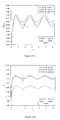••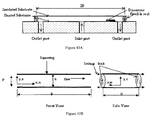•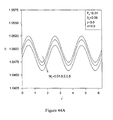•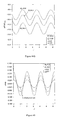•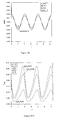•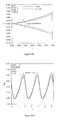•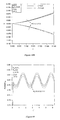•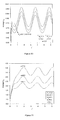•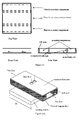•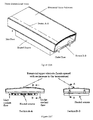•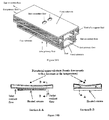•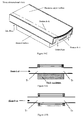•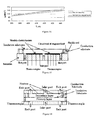•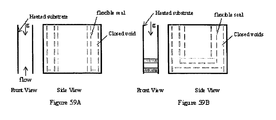•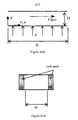•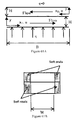•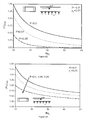•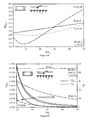•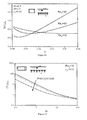•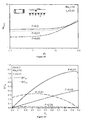•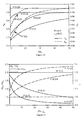•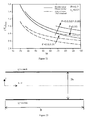•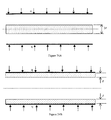•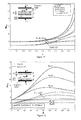•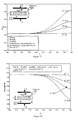•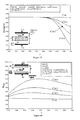•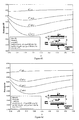•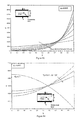•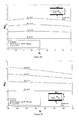•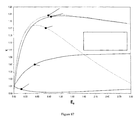•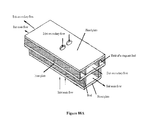•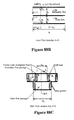## Classifications

• BPERFORMING OPERATIONS; TRANSPORTING
• B01PHYSICAL OR CHEMICAL PROCESSES OR APPARATUS IN GENERAL
• B01JCHEMICAL OR PHYSICAL PROCESSES, e.g. CATALYSIS OR COLLOID CHEMISTRY; THEIR RELEVANT APPARATUS
• B01J19/00Chemical, physical or physico-chemical processes in general; Their relevant apparatus
• B01J19/0093Microreactors, e.g. miniaturised or microfabricated reactors
• BPERFORMING OPERATIONS; TRANSPORTING
• B01PHYSICAL OR CHEMICAL PROCESSES OR APPARATUS IN GENERAL
• B01LCHEMICAL OR PHYSICAL LABORATORY APPARATUS FOR GENERAL USE
• B01L3/00Containers or dishes for laboratory use, e.g. laboratory glassware; Droppers
• B01L3/50Containers for the purpose of retaining a material to be analysed, e.g. test tubes
• B01L3/502Containers for the purpose of retaining a material to be analysed, e.g. test tubes with fluid transport, e.g. in multi-compartment structures
• B01L3/5027Containers for the purpose of retaining a material to be analysed, e.g. test tubes with fluid transport, e.g. in multi-compartment structures by integrated microfluidic structures, i.e. dimensions of channels and chambers are such that surface tension forces are important, e.g. lab-on-a-chip
• B01L3/502746Containers for the purpose of retaining a material to be analysed, e.g. test tubes with fluid transport, e.g. in multi-compartment structures by integrated microfluidic structures, i.e. dimensions of channels and chambers are such that surface tension forces are important, e.g. lab-on-a-chip characterised by the means for controlling flow resistance, e.g. flow controllers, baffles
• BPERFORMING OPERATIONS; TRANSPORTING
• B82NANOTECHNOLOGY
• B82YSPECIFIC USES OR APPLICATIONS OF NANOSTRUCTURES; MEASUREMENT OR ANALYSIS OF NANOSTRUCTURES; MANUFACTURE OR TREATMENT OF NANOSTRUCTURES
• B82Y30/00Nanotechnology for materials or surface science, e.g. nanocomposites
• BPERFORMING OPERATIONS; TRANSPORTING
• B01PHYSICAL OR CHEMICAL PROCESSES OR APPARATUS IN GENERAL
• B01JCHEMICAL OR PHYSICAL PROCESSES, e.g. CATALYSIS OR COLLOID CHEMISTRY; THEIR RELEVANT APPARATUS
• B01J2219/00Chemical, physical or physico-chemical processes in general; Their relevant apparatus
• B01J2219/00781Aspects relating to microreactors
• B01J2219/00783Laminate assemblies, i.e. the reactor comprising a stack of plates
• BPERFORMING OPERATIONS; TRANSPORTING
• B01PHYSICAL OR CHEMICAL PROCESSES OR APPARATUS IN GENERAL
• B01JCHEMICAL OR PHYSICAL PROCESSES, e.g. CATALYSIS OR COLLOID CHEMISTRY; THEIR RELEVANT APPARATUS
• B01J2219/00Chemical, physical or physico-chemical processes in general; Their relevant apparatus
• B01J2219/00781Aspects relating to microreactors
• B01J2219/00801Means to assemble
• B01J2219/00804Plurality of plates
• BPERFORMING OPERATIONS; TRANSPORTING
• B01PHYSICAL OR CHEMICAL PROCESSES OR APPARATUS IN GENERAL
• B01JCHEMICAL OR PHYSICAL PROCESSES, e.g. CATALYSIS OR COLLOID CHEMISTRY; THEIR RELEVANT APPARATUS
• B01J2219/00Chemical, physical or physico-chemical processes in general; Their relevant apparatus
• B01J2219/00781Aspects relating to microreactors
• B01J2219/00801Means to assemble
• B01J2219/00804Plurality of plates
• B01J2219/00808Sealing means
• BPERFORMING OPERATIONS; TRANSPORTING
• B01PHYSICAL OR CHEMICAL PROCESSES OR APPARATUS IN GENERAL
• B01JCHEMICAL OR PHYSICAL PROCESSES, e.g. CATALYSIS OR COLLOID CHEMISTRY; THEIR RELEVANT APPARATUS
• B01J2219/00Chemical, physical or physico-chemical processes in general; Their relevant apparatus
• B01J2219/00781Aspects relating to microreactors
• B01J2219/00858Aspects relating to the size of the reactor
• B01J2219/00864Channel sizes in the nanometer range, e.g. nanoreactors
• BPERFORMING OPERATIONS; TRANSPORTING
• B01PHYSICAL OR CHEMICAL PROCESSES OR APPARATUS IN GENERAL
• B01JCHEMICAL OR PHYSICAL PROCESSES, e.g. CATALYSIS OR COLLOID CHEMISTRY; THEIR RELEVANT APPARATUS
• B01J2219/00Chemical, physical or physico-chemical processes in general; Their relevant apparatus
• B01J2219/00781Aspects relating to microreactors
• B01J2219/00873Heat exchange
• BPERFORMING OPERATIONS; TRANSPORTING
• B01PHYSICAL OR CHEMICAL PROCESSES OR APPARATUS IN GENERAL
• B01JCHEMICAL OR PHYSICAL PROCESSES, e.g. CATALYSIS OR COLLOID CHEMISTRY; THEIR RELEVANT APPARATUS
• B01J2219/00Chemical, physical or physico-chemical processes in general; Their relevant apparatus
• B01J2219/00781Aspects relating to microreactors
• B01J2219/00873Heat exchange
• B01J2219/00876Insulation elements
• BPERFORMING OPERATIONS; TRANSPORTING
• B01PHYSICAL OR CHEMICAL PROCESSES OR APPARATUS IN GENERAL
• B01LCHEMICAL OR PHYSICAL LABORATORY APPARATUS FOR GENERAL USE
• B01L2300/08Geometry, shape and general structure
• B01L2300/0861Configuration of multiple channels and/or chambers in a single devices
• B01L2300/0874Three dimensional network
• BPERFORMING OPERATIONS; TRANSPORTING
• B01PHYSICAL OR CHEMICAL PROCESSES OR APPARATUS IN GENERAL
• B01LCHEMICAL OR PHYSICAL LABORATORY APPARATUS FOR GENERAL USE
• B01L2400/00Moving or stopping fluids
• B01L2400/06Valves, specific forms thereof
• B01L2400/0633Valves, specific forms thereof with moving parts
• BPERFORMING OPERATIONS; TRANSPORTING
• B01PHYSICAL OR CHEMICAL PROCESSES OR APPARATUS IN GENERAL
• B01LCHEMICAL OR PHYSICAL LABORATORY APPARATUS FOR GENERAL USE
• B01L2400/00Moving or stopping fluids
• B01L2400/08Regulating or influencing the flow resistance
• B01L2400/082Active control of flow resistance, e.g. flow controllers
• BPERFORMING OPERATIONS; TRANSPORTING
• B01PHYSICAL OR CHEMICAL PROCESSES OR APPARATUS IN GENERAL
• B01LCHEMICAL OR PHYSICAL LABORATORY APPARATUS FOR GENERAL USE
• B01L7/00Heating or cooling apparatus; Heat insulating devices
• FMECHANICAL ENGINEERING; LIGHTING; HEATING; WEAPONS; BLASTING
• F23COMBUSTION APPARATUS; COMBUSTION PROCESSES
• F23CMETHODS OR APPARATUS FOR COMBUSTION USING FLUID FUEL OR SOLID FUEL SUSPENDED IN  A CARRIER GAS OR AIR
• F23C2900/00Special features of, or arrangements for combustion apparatus using fluid fuels or solid fuels suspended in air; Combustion processes therefor
• F23C2900/03001Miniaturized combustion devices using fluid fuels
• FMECHANICAL ENGINEERING; LIGHTING; HEATING; WEAPONS; BLASTING
• F23COMBUSTION APPARATUS; COMBUSTION PROCESSES
• F23NREGULATING OR CONTROLLING COMBUSTION
• F23N2900/00Special features of, or arrangements for controlling combustion
• F23N2900/01001Micro Electro Mechanical Systems [MEMS] for controlling fuel supply to burners
• FMECHANICAL ENGINEERING; LIGHTING; HEATING; WEAPONS; BLASTING
• F28HEAT EXCHANGE IN GENERAL
• F28FDETAILS OF HEAT-EXCHANGE AND HEAT-TRANSFER APPARATUS, OF GENERAL APPLICATION
• F28F13/00Arrangements for modifying heat-transfer, e.g. increasing, decreasing
• FMECHANICAL ENGINEERING; LIGHTING; HEATING; WEAPONS; BLASTING
• F28HEAT EXCHANGE IN GENERAL
• F28FDETAILS OF HEAT-EXCHANGE AND HEAT-TRANSFER APPARATUS, OF GENERAL APPLICATION
• F28F2230/00Sealing means
• FMECHANICAL ENGINEERING; LIGHTING; HEATING; WEAPONS; BLASTING
• F28HEAT EXCHANGE IN GENERAL
• F28FDETAILS OF HEAT-EXCHANGE AND HEAT-TRANSFER APPARATUS, OF GENERAL APPLICATION
• F28F3/00Plate-like or laminated elements; Assemblies of plate-like or laminated elements
• F28F3/08Elements constructed for building-up into stacks, e.g. capable of being taken apart for cleaning
• F28F3/10Arrangements for sealing the margins

## Abstract

Devices for the control of flow rate and thermal conditions using two-layered thin films separated by flexible complex seals. The plates of an upper thin film are separated by a sealing assembly. The sealing assembly is composed of an elastic soft seal separating closed voids of stagnant fluid and in contact with the upper plate of the device as it is the heated plate. The lower and the upper plates of the lower thin film can be separated by an elastic soft seal. The lower and upper thin films are named as main and secondary layers, respectively. Both the upper plate of the secondary layer and the lower plate of the main layer are fixed while the intermediate plate and is free to move in the vertical direction. When the pressure or the working temperature in the secondary layer increases, the secondary layer expands causing the main layer thickness to shrink. This reduces the main layer flow rate and temperature gradients within the main layer.

## Description

CROSS-REFERENCE TO RELATED APPLICATIONS

This patent is a Continuation-in-Part of U.S. patent application Ser. No. 12/793,826, entitled “Methods and Devices Comprising Flexible Seals, Flexible Microchannels, or Both for Modulating or Controlling Flow and Heat” filed on Jun. 6, 2010, which in turn is a continuation of U.S. patent application Ser. No. 11/184,932, entitled “Methods and Devices Comprising Flexible Seals, Flexible Microchannels, or Both For Modulating or Controlling Flow and Heat,” which was filed on Jul. 20, 2005, the disclosure of which is incorporated herein by reference in its entirety. U.S. patent application Ser. No. 11/184,932 in turn is a continuation-in-part of U.S. patent application Ser. No. 10/840,303, filed 7 May 2004, which claims the benefit of U.S. Provisional Patent Application No. 60/470,850 filed 16 May 2003, which are herein incorporated by reference in their entirety.

BACKGROUND OF THE INVENTION

1. Field of the Invention

The present invention generally relates to thin film channels, microfluidic devices, biosensors, electronic cooling, control of fuel flow prior combustion and insulating assemblies.

2. Description of the Related Art

Thin films are used in a variety of devices, including electrical, electronic, chemical, and biological devices, for modulating or controlling flow and heat characteristics in the devices. See e.g. Vafai & Wang (1992) Int. J. Heat Mass Transfer 35:2087-2099, Vafai et al. (1995) ASME J Heat Transfer 117:209-218, Zhu & Vafai (1997) Int. J. Heat Mass Transfer 40:2887-2900, and Moon et al. (2000) Int. J. Microcircuits and Electronic Packaging 23:488-493 for flat heat pipes; Fedorov & Viskanta (2000) Int. J. Heat Mass Transfer 43:399-415, Lee and Vafai (1999) Int. J. Heat Mass Transfer 42:1555-1568, and Vafai & Zhu (1999) Int. J. Heat Mass Transfer 42; 2287-2297 for microchannel heat sinks; Lavrik et al. (2001) Biomedical Microdevices 3(1):35-44, and Xuan & Roetzel (2000) Int. J. Heat Mass Transfer 43:3701-3707 for biosensors and nanodevices.

For many of these applications, modulation and control of the flow and heat characteristics in the devices is desired. Unfortunately, the prior art methods for modulating and controlling the flow and heat are difficult or problematic. For example, a two-phase flow in a microchannel is capable of removing maximum heat fluxes generated by electronic packages, but instability occurs near certain operating conditions. See Bowers & Mudwar (1994) ASME J. Electronic Packaging 116:290-305. Further, the use of porous medium for cooling electronic devices enhances heat transfer via the increase in the effective surface area, but the porous medium results in a substantial increase in the pressure drop inside the thin film. See Huang & Vafai (1993) Int. J. Heat Mass Transfer 36:4019-4032, Huang & Vafai (1994) AIAA J. Thermophysics and Heat Transfer 8:563-573, Huang & Vafai (1994) Int. J. Heat and Fluid Flow 15:48-61, and Hadim (1994) ASME J. Heat Transfer 116:465-472.

Therefore, a need still exists for methods of modulating or controlling heat and flow characteristics in thin films.

SUMMARY OF THE INVENTION

The disclosed embodiments generally relate to thin film channels, microfluidic devices, biosensors, electronic cooling, control of fuel flow prior to combustion and insulating assemblies.

The disclosed embodiments provide methods and devices for modulating flow and heat in a variety of thermal systems including thin film channels, microfluidics, insulating assemblies, and the like with no need for external cooling or flow controlling devices.

The disclosed embodiments can provide, for example, devices for modulating flow and heat. Such devices can reduce the temperature as the thermal load increases as related to electronic cooling and cooling of engine applications. Devices are also disclosed herein that reduce the flow rate as the thermal load increases, which are important to internal combustion applications where fuel rate needs to be reduced as the engine gets overheated. Such devices can conserve thermal energy as the temperature increases and to reduce leakage from microfluidics. These devices have applications related to thermal insulations and biosensor devices among others.

It is to be understood that both the foregoing general description and the following detailed description are exemplary and explanatory only and are intended to provide further explanation of the invention as claimed. The accompanying drawings are included to provide a further understanding of the invention and are incorporated in and constitute part of this specification, illustrate several embodiments of the invention, and together with the description serve to explain the principles of the disclosed embodiments.

DESCRIPTION OF THE DRAWINGS

This invention is further understood by reference to the drawings wherein:

FIG. 1 shows an insulating assembly comprising the flexible seals of the present invention.

FIG. 2 shows primary fluid layer expansion versus its temperature.

FIG. 3 shows the percentage volumetric thermal expansion for the conditions of isobaric expansion and expansion using a linearized model under linearly varying pressure.

FIG. 4 shows dimensionless change in the equivalent resistance of the fluid layers for two different fluids.

FIG. 5 shows enhanced insulating properties using xenon and an insulating assembly using the flexible seals according to the present invention.

FIG. 6 shows deteriorated insulating properties using helium and an insulating assembly using the flexible seals according to the present invention.

FIG. 7 shows reduction of thermal losses at large operating temperatures using xenon and an insulating assembly using the flexible seals according to the present invention.

FIG. 8 shows deterioration of thermal losses at large operating temperatures using helium and an insulating assembly using the flexible seals according to the present invention.

FIG. 9 shows advanced assemblies with enhanced insulating properties comprising the flexible seals according to the present invention.

FIG. 10 shows the schematic diagram for a thin film and the coordinate system.

FIG. 11 shows the effects of the fixation parameter on the thin film thickness.

FIG. 12 shows the effects of the fixation parameter on the fluctation at the upper substrate.

FIG. 13 shows the effects of the frequency of internal pressure pulsations on the fluctation at the upper substrate.

FIG. 14 shows the effects of the squeezing number on the thin film thickness.

FIG. 15 shows the effects of the squeezing number on the fluctation at the upper substrate.

FIG. 16 shows the effects of the phase shift of the internal pressure on the thin film thickness.

FIG. 17 shows the effects of the thermal squeezing parameter and the fixation parameter on the mean bulk temperature.

FIG. 18 shows the effects of the thermal squeezing parameter and the fixation parameter on the average lower substrate temperature.

FIG. 19 shows the effects of the fixation parameter on the Nusselt number for constant wall temperature conditions.

FIG. 20 shows the effects of the fixation parameter on the Nusselt number for uniform wall heat flux conditions.

FIG. 21 shows the axial development of the Nusselt number versus the fixation parameter.

FIG. 22 shows the effects of the frequency of pulsations on the average heat transfer.

FIG. 23 shows the effects of the frequency of pulsations on the average lower substrate temperature.

FIG. 24 shows the effects frequency of pulsations on the fluctuation in the average heat transfer and the average lower substrate temperature.

FIG. 25A is a 3D view of a schematic diagram for a two-layered thin film supported by flexible seals and flexible complex seals of the present invention.

FIG. 25B shows the front and side views including the main boundary conditions of the schematic diagram for a two-layered thin film supported by flexible seals and flexible complex seals of the present invention.

FIG. 26A shows the effects of E* on ΨX=0.5 and dH1/dτ*(Ht=2.0, E*1=E*2=E*, FT=0.15, PS1=PS2=1.0, βp=0.3, βq=0.2, φp=π/2, γ=3.0, γp=6.0, λ12=0, σ1=3.0, σ2=6.0).

FIG. 26B shows the effects of E* on ΘAVG and (θu)AVG (Ht=2.0, E*1=E*2=E*, FT=0.15, PS1=PS2=1.0, βp=0.3, βp=0.2, φp=π/2, γ=3.0, γp=6.0, λ12=0, σ1=3.0, σ2=6.0).

FIG. 27A shows the effects of FT on ΨX=0.5 and dH1/dτ* (Ht=2.0, E*1=0.3, E*2=0.003, PS1=1.0, PS2=0.012, βp=0.3, βq=0.2, φp=π/2, γ=3.0, γp=6.0, λ12=0, σ1=6.0, σ2=1.0).

FIG. 27B shows the effects of FT on ΘAVG and (θu)AVG (Ht=2.0, E*1=0.3, E*2=0.003, PS1=1.0, PS2=0.012, βp=0.3, βq=0.2, φp=π/2, γ=3.0, γp=6.0, λ12=0, σ1=6.0, σ2=1.0).

FIG. 28 shows the effects of FT on Nusselt numbers for primary and secondary flows: (primary flow maintained at a CIF condition, Ht=2.0, E; =E*1=E*2=0.2, PS1=PS2=1.0, βp=0.3, βq=0.2, φp=π/2, γ=3.0, γp=6.0, λ12=0, σ2=6.0).

FIG. 29A shows the effects of σ1 on ΨX=0.5 and dH1/dτ* (Ht=2.0, E*1=E*2=E*=0.2, FT=0.15, PS1=PS2=1.0, βp=0.3, βq=0.2, φp=π/2, γ=3.0, γp=6.0, λ12=0, σ2=6.0).

FIG. 29B shows the effects of σ1 on ΘAVG and (θu)AVG (Ht=2.0, E*1=E*2=E*=0.2, FT=0.15, PS1=PS2=1.0, βp=0.3, βq=0.2, φp=π/2, γ=3.0, γp=6.0, λ12=0, σ2=6.0).

FIG. 30A shows the effects of PS2 on ΨX=0.5 and dH1/dτ* (Ht=2.0, E*1=E*2=E*=0.2, FT=0.15, PS1=1.0, βp=0.3, βq=0.2, φp=π/2, γ=3.0, γp=6.0, λ12=0, σ1=5.0, σ2=6.0).

FIG. 30B shows the effects of PS2 on ΨAVG and (θu)AVG (Ht=2.0, E*1=E*2=E*=0.2, FT=0.15, PS1=1.0, βp=0.3, βq=0.2, φp=π/2, γ=3.0, γp=6.0, λ12=0, σ1=5.0, σ2=6.0).

FIG. 31 shows the effects of PS2 on Nusselt numbers for primary and secondary flows: (primary flow maintained at a CIF condition, Ht=2.0, E*1=E*2=E*=0.2, FT=0.15, PS1=1.0, βp=0.3, βq=0.2, φp=π/2, γ=3.0, γp=6.0, σ1=5.0, σ2=6.0).

FIG. 32A shows the effects of λ2 on ΨX=0.5 and dH1/dτ* (primary flow maintained at a CIP condition, Ht=2.0, E*1=E*2=E*=0.2, FT=0.15, PS1=PS2=1.0, βp=0.3, βq=0.2, φp=π/2, γ=3.0, γp=6.0, λ1=0, σ1=3.0, σ2=6.0).

FIG. 32B shows the effects of λ2 on ΘAVG and (θu)AVG (primary flow maintained at a CIP condition, Ht=2.0, E*1=E*2=E*=0.2, FT=0.15, PS1=PS2=1.0, βp=0.3, βq=0.2, φp=π/2, γ=3.0, γp=6.0, λ1=0, σ1=3.0, σ2=6.0).

FIG. 33A shows the effects of λ2 on ΨX=0.5 and dH1/dτ* (primary flow maintained at a CIF condition, Ht=2.0, E*1=E*2=E*=0.2, FT=0.15, PS1=PS2=1.0, βp=0.3, βq=0.2, φp=π/2, γ=3.0, γp=6.0, λ1=0, σ1=3.0, σ2=6.0).

FIG. 33B shows the effects of λ2 on ΨAVG and (θu)AVG (primary flow maintained at a CIF condition, Ht=2.0, E*1=E*2=E*=0.2, FT=0.15, PS1=PS2=1.0, βp=0.3, βq=0.2, φp=π/2, γ=3.0, γp=6.0, λ1=0, σ1=3.0, σ2=6.0).

FIG. 34 shows the effects of γp on ΔΨX=0.5 and Δ(θu)AVG for the CIP condition (Ht=2.0, E*1=E*2=E*=0.3, FT=0.3, PS1=PS2=1.0, βp=0.3, βq=0.2, φp=π/2, γ=3.0, λ12=0, σ1=3.0, σ2=6.0).

FIG. 35 shows the effects of Ht on PX=0.5 and dH1/dτ* (primary flow maintained at a CIP condition, E*1=E*2=E*=0.2, FT=0.15, PS1=PS2=1.0, βp=0.3, βq=0.2, φp=π/2, γ=3.0, γp=6.0, λ12=0, σ1=3.0, σ2=6.0).

FIG. 36A is a front view of a schematic diagram for a thin film with flexible complex seal according to the present invention and the corresponding coordinate system.

FIG. 36B is a side view of a schematic diagram for a thin film with flexible complex seal according to the present invention and the corresponding coordinate system.

FIG. 36C is a 3D diagram of a schematic diagram for a thin film with flexible complex seal according to the present invention and the corresponding coordinate system.

FIG. 37A shows the effects of the dimensionless thermal expansion parameter FT on dimensionless thin film thickness H.

FIG. 37B shows the effects of the dimensionless thermal expansion parameter FT on dimensionless average lower substrate temperature (θW)AVG.

FIG. 37C shows the effects of the dimensionless thermal expansion parameter FT on dH/dτ.

FIG. 37D shows the effects of the dimensionless thermal expansion parameter FT on exit Nusselt number NuL

FIG. 38A shows the effects of the dimensionless thermal dispersion parameter λ on dimensionless average lower substrate temperature (θW)AVG.

FIG. 38B shows the effects of the dimensionless thermal dispersion parameter λ on dimensionless thickness H.

FIG. 38C shows the effects of the dimensionless thermal dispersion parameter λ on temperature profile.

FIG. 38D shows the effects of the dimensionless thermal dispersion parameter λ on exit Nusselt number NuL

FIG. 39 shows effects of the dimensionless dispersion parameter λ on the time variation of the dimensionless thin film thickness dH/dτ.

FIG. 40A shows effects of the thermal squeezing parameter PS and the squeezing number a on dimensionless average lower substrate temperature (θW)AVG.

FIG. 40B shows effects of the thermal squeezing parameter PS and the squeezing number a on dimensionless thin film thickness H.

FIG. 40C shows effects of the thermal squeezing parameter PS and the squeezing number σ on dH/dτ.

FIG. 41A shows effects of the fixation parameter Fn and the dimensionless thermal load amplitude βq on dimensionless average lower substrate temperature (θW)AVG.

FIG. 41B shows effects of the fixation parameter Fn and the dimensionless thermal load amplitude βq on dimensionless thin film thickness H.

FIG. 42 shows effects of the dimensionless thermal expansion parameter FT on the average dimensionless pressure inside the thin film ΠAVG.

FIG. 43A is a schematic diagram of a symmetrical fluidic cell (it has a uniform variation in the film thickness under disturbed conditions and can be used for multi-detection purposes).

FIG. 43B is a schematic diagram of corresponding coordinate systems with leakage illustration.

FIG. 44A shows effects of the dimensionless leakage parameter ML on the dimensionless thin film thickness H, the film thickness decreases with an increase in the leakage.

FIG. 44B shows effects of the dimensionless leakage parameter ML on the inlet pressure gradient.

FIG. 45 shows effects of the fixation parameter Fn on the fluctuation rate at the upper substrate dH/dτ. The fluctuation rate increases as the seal becomes softer.

FIG. 46 shows effects of the squeezing number a on the fluctuation rate at the upper substrate dH/dτ. The fluctuation rate decreases as the order of the inlet velocity decreases compared to the axial squeezed velocity due to pressure pulsations.

FIG. 47A shows the effects of the dimensionless slip parameter βP/ho on the dimensionless wall slip velocity Uslip.

FIG. 47B shows the effects of the dimensionless slip parameter βP/ho on the dimensionless normal velocity V (the dimensionless time τ*=3π/2 corresponds to the time at which the fluctuation rate at the upper substrate is maximum while τ*=π/6 corresponds to the time at which the fluctuation rate at the upper substrate is minimum).

FIG. 48A shows the effects of the power law index n on the dimensionless wall slip velocity Uslip.

FIG. 48B shows the effects of the power law index n on the dimensionless normal velocity V (the dimensionless time τ*=3π/2 corresponds to the time at which the fluctuation rate at the upper substrate is maximum while τ*=11π/6 corresponds to the time at which the fluctuation rate at the upper substrate is minimum).

FIG. 49 shows the effects of the dimensionless leakage parameter ML on the average dimensionless lower substrate temperature θW. The cooling increases with an increase in the leakage rate.

FIG. 50 shows the effects of the fixation parameter Fn on the average dimensionless lower substrate temperature θW. The cooling increases as the seal becomes softer.

FIG. 51 shows the effects of the squeezing number a on the average dimensionless lower substrate temperature θW. The cooling increases as the order of the inlet velocity increases.

FIG. 52 shows a multi-compartment fluidic cell.

FIG. 53A shows systems with increased cooling capacity as thermal load increases utilizing a flexible complex seal according to the present invention.

FIG. 53B shows systems with increased cooling capacity as thermal load increases utilizing a bimaterial upper substrate.

FIG. 53C shows an alternative embodiment with respect to FIGS. 53A and 53B.

FIG. 54A shows systems with decreased cooling capacity as thermal load increases utilizing flexible complex seals according to the present invention and two layered thin films.

FIG. 54B shows systems with decreased cooling capacity as thermal load increases utilizing a bimaterial upper substrate.

FIG. 54C shows an alternative embodiment with respect to FIGS. 54A and 54B.

FIG. 55A shows an insulating assembly arrangement for low temperature applications.

FIG. 55B shows an insulating assembly arrangement for high temperature applications.

FIG. 56 shows expected sample results for xenon with and without the flexible seals of the present invention.

FIG. 57 shows a thin film supported by flexible complex seals of the present invention with one inlet port and two exit ports.

FIG. 58 shows a two-layer thin film supported by flexible complex seals of the present invention with two inlet ports and four exit ports.

FIG. 59A is a schematic for a vertical channel supported by flexible complex seals.

FIG. 59B is a schematic for an open-ended cell supported by flexible complex seals.

FIG. 60A is a front view of a schematic diagram and the coordinate system for a single layer flexible microchannel heat sink of the present invention.

FIG. 60B is a side view of a schematic diagram and the coordinate system for a single layer flexible microchannel heat sink of the present invention.

FIG. 61A is a front view of a schematic diagram and the coordinate system for a double layered flexible microchannel heat sink of the present invention.

FIG. 61B is a side view of a schematic diagram and the coordinate system for a double layer flexible microchannel heat sink of the present invention.

FIG. 62 Effects of the pressure drop

$( Re o = ρ 12 ⁢ ⁢ μ 2 ⁢ Δ ⁢ ⁢ p B ⁢ H o 3 )$
on the dimensionless exit mean bulk temperature for a single layer flexible microchannel heat sink.

FIG. 63 Effects of the pressure drop

$( Re o = ρ 12 ⁢ ⁢ μ 2 ⁢ Δ ⁢ ⁢ p B ⁢ H o 3 )$
on the dimensionless average lower plate temperature for a single layer flexible microchannel heat sink.

FIG. 64 Effects of the pressure drop

$( Re o = ρ 12 ⁢ ⁢ μ 2 ⁢ Δ ⁢ ⁢ p B ⁢ H o 3 )$
on the dimensionless average convective heat transfer coefficient for a single layer flexible microchannel heat sink.

FIG. 65 Effects of the pressure drop

$( Re o = ρ 12 ⁢ ⁢ μ 2 ⁢ Δ ⁢ ⁢ p B ⁢ H o 3 )$
on UReo and UF for a single layer flexible microchannel heat sink.

FIG. 66 Effects of the fixation parameter on the fully developed heated plate temperature at the exit for a single layer flexible microchannel heat sink.

FIG. 67 Effects of Prandtl number on the dimensionless average lower plate temperature for a single layer flexible microchannel heat sink.

FIG. 68 Effects of Prandtl number on the average convective heat transfer coefficient for a single layer flexible microchannel heat sink.

FIG. 69 Effects of the fixation parameter on the mean bulk temperature inside the double layered flexible microchannel heat sink

FIG. 70 Effects of the pressure drop

$( Re o = ρ 12 ⁢ ⁢ μ 2 ⁢ Δ ⁢ ⁢ p B ⁢ H o 3 )$
on κm and κW

FIG. 71 Effects of the pressure drop

$( ( Re o ) DL = ρ 12 ⁢ μ 2 ⁢ ( Δ ⁢ ⁢ p ) DL B ⁢ H o 3 )$
on the pressure drop ratio and the friction force ratio between single and double-layered flexible microchannel heat sinks.

FIG. 72 Effects of the delivered coolant mass flow rate on the average heated plate temperature for both single and double layered flexible microchannel heat sinks.

FIG. 73 is a schematic diagram and the coordinate system.

FIG. 74 shows different arrangements for the thermal dispersion region: (a) central arrangement, and (b) boundary arrangement.

FIG. 75 shows effects of the thermal dispersion parameter Eo and the dimensionless thickness Λ on the Nusselt number at thermally fully developed conditions for the central arrangement (the number of the dispersive elements is the same for each arrangement).

FIG. 76 shows effects of the thermal dispersion parameter Eo and the dimensionless thickness Λ on the Nusselt number at thermally fully developed conditions for the boundary arrangement (the number of the dispersive elements is the same for each arrangement).

FIG. 77 shows effects of the dispersion coefficient C* and the dimensionless thickness Λ on the Nusselt number at the exit for central arrangement (the number of the dispersive elements is the same for each arrangement).

FIG. 78 shows effects of the dispersion coefficient C* and the dimensionless thickness Λ on the average dimensionless plate temperature θW for central arrangement (the number of the dispersive elements is the same for each arrangement, Pef=670).

FIG. 79 shows effects of the dispersion coefficient C* and the dimensionless thickness Λ on the average dimensionless plate temperature θW for central arrangement (the number of the dispersive elements is the same for each arrangement, Pef=1340).

FIG. 80 shows effects of the dispersion coefficient C* and the dimensionless thickness Λ on the Nusselt number at the exit for the boundary arrangement (the number of the dispersive elements is the same for each arrangement).

FIG. 81 shows effects of the dispersion coefficient C* and the dimensionless thickness Λ on the average dimensionless plate temperature θW for boundary arrangement (the number of the dispersive elements is the same for each arrangement, Pef=670).

FIG. 82 shows effects of the dispersion coefficient C* and the dimensionless thickness Λ on the average dimensionless plate temperature θW for boundary arrangement (the number of the dispersive elements is the same for each arrangement, Pef=1340).

FIG. 83 shows effects of De on the volume fraction distribution of the dispersive element (the number of the dispersive elements is the same for each distribution).

FIG. 84 shows effects of Dc on the volume fraction distribution of the dispersive elements (the number of the dispersive elements is the same for each distribution).

FIG. 85 shows effects of De on the fully developed value for the Nusselt number (exponential distribution, the number of the dispersive elements is the same for each distribution).

FIG. 86 shows effects of Dc on the fully developed value for the Nusselt number (parabolic distribution, the number of the dispersive elements is the same for each distribution).

FIG. 87 is a graph that shows that the excess in Nusselt number κ is always greater than one for the boundary arrangement while it is greater than one for the exponential distribution when the velocity is uniform.

FIG. 88A is a schematic diagram of a two-layered thin film supported by flexible complex seals, in accordance with the disclosed embodiments.

FIG. 88B is a front view of two-layered thin film depicted in FIG. 88A, in accordance with the disclosed embodiments.

FIG. 88C is a side view of two-layered thin film depicted in FIG. 88A, in accordance with the disclosed embodiments.

DETAILED DESCRIPTION OF THE INVENTION

The present invention provides methods for modulating or controlling heat and flow characteristics in a variety of devices. In particular, the present invention provides flexible seals for modulating or controlling heat and flow characteristics in devices comprising thin films, such as thin film channels, microchannels, microfluidics and the like. The present invention also provides a method to control heat and flow inside other thermal systems, such as insulating assemblies and fuel flow passages. As used herein, a “flexible seal” refers to a material that can be deformed significantly according to the load acting upon it. Examples of these materials include elastmors, polymers, natural rubber, closed rubber cell foams, and the like. In some embodiments, the present invention provides flexible complex seals for modulating or controlling heat and flow characteristics in devices comprising thin films, such as microchannels and microfluidics. As used herein, a “flexible complex seal” refers to a flexible seal comprising at least one closed cavity of stagnant fluid. In preferred embodiments, the stagnant fluid has at least one point of contact with the heated surface of the device. In preferred embodiments, the stagnant fluid has a large value of the volumetric thermal coefficient. As used herein, a “fluid” refers to a continuous amorphous substance that tends to flow and to conform to the outline of a container, such as a liquid or a gas, and may be used in accordance with the present invention. As used herein, the term “stagnant fluid” refers generally to a fluid that is not circulating or flowing and in preferred embodiments, a flexible seal and/or the surfaces of a device can surround the stagnant fluid such that the average translational velocity of the fluid is zero.

As used herein, “primary fluid” refers to the fluid that the devices of the present invention control or modulate its flow rate or its temperature. As used herein, “secondary fluid” refers to an auxiliary fluid utilized in the present invention to achieve additional control and modulation features for the primary fluid flow rate and temperature. As provided herein, the stagnant fluid in the complex flexible seals can have characteristics that are the same as or different from the characteristics of the primary fluid, the secondary fluid, or both. As used herein, “biofluid” refers to the fluid that contains at least one species of a biological substance that needs to be measured. As provided herein, the primary fluid can be a biofluid.

The flexible seals and flexible complex seals of the present invention are typically found between a first substrate and a second substrate of a thin film or other thermal systems such as the insulating assemblies. As used herein, “substrate” includes plates, which may be inflexible or flexible according to part 6 herein below. In some preferred embodiments, the elastic modulus for the seals of the present invention, the ratio of the applied stress on the seal to the induced strain, range from about 103N/m2 to about 107N/m2. The seals of the present invention may comprise at least one closed cavity of a fluid such as air or the like in order to minimize their effective elastic modulus. The deformation of the flexible seals can be guided by special guiders to attain maximum or desired deformations. In preferred embodiments, the flexible seals comprise different cross-sectional geometries, such as circular cross-section, rectangular cross-section and the like. As used herein, “thin films” include fluidic devices that have the thickness of their fluidic layers of an order of about a millimeter or less such as, microchannels and microfluidic devices. Thin films comprise at least two substrates, lower and upper substrates, and at least one fluidic layer. As used herein, an “insulating assembly” means an assembly of at least two insulating substrates and at least one fluid layer placed consecutively in series.

The flexible seals and flexible complex seals of the present invention are typically found between a first substrate and a second substrate of a thin film or other thermal systems such as the insulating assemblies. As used herein, “substrate” includes plates, which may be inflexible or flexible according to part 6 herein below. In some preferred embodiments, the elastic modulus for the seals of the present invention, the ratio of the applied stress on the seal to the induced strain, range from about 103N/m2 to about 107N/m2. The seals of the present invention may comprise at least one closed cavity of a fluid such as air or the like in order to minimize their effective elastic modulus. The deformation of the flexible seals of the disclosed embodiments can be guided by special guiders to attain maximum or desired deformations. In preferred embodiments, the flexible seals comprise different cross-sectional geometries, such as circular cross-section, rectangular cross-section and the like. As used herein, “thin films” include fluidic devices that have the thickness of their fluidic layers of an order of about a millimeter or less such as, microchannels and microfluidic devices. Thin films comprise at least two substrates, lower and upper substrates, and at least one fluidic layer. As used herein, an “insulating assembly” means an assembly of at least two insulating substrates and at least one fluid layer placed consecutively in series.

As disclosed herein, modulating the thermal characteristics of a device may be conducted by modifying the thin film thickness, the thermal load, the flow rate, or a combination thereof. For example, additional cooling can be achieved if the thin film thickness is allowed to increase by an increase in the thermal load, pressure gradient or both which will cause the coolant flow rate to increase. As provided herein, the enhancement in the cooling due to the flexible complex seals used is substantial at larger thermal loads for stagnant liquids while this enhancement is much larger at lower temperatures for stagnant fluids, especially ideal gases. This is because the volumetric thermal expansion coefficient increases for liquids and decreases for gases as the temperature increases. Moreover, the enhancement in the cooling due to flexible seals is substantial at larger pressure gradients for single layered thin films while it is significant for double-layered thin films at lower pressure gradients.

Khaled and Vafai analyzed the enhancement in the heat transfer inside thin films supported by flexible complex seals. See Khaled & Vafai (2003) ASME J. of Heat Transfer 125:916-925, which is herein incorporated by reference. Specifically, the applied thermal load was considered to vary periodically with time in order to investigate the behavior of expandable thin film systems in the presence of a noise in the applied thermal load. As provided herein, a noticeable enhancement in the cooling capacity can be achieved for large thermal loads especially in cooling of high flux electronic components (q≈700 kW/m2) since they produce elevated working temperatures. Also, the generated squeezing effects at the mobile and inflexible substrate can be minimized when nanofluids are employed in the coolant flow. As used herein, “nanofluids” are mixtures of a working fluid, such as water, and suspended ultrafine particles in the fluid such as copper, aluminum, or the like with diameters of an order of about the nanometer range. See Eastman et al. (2001) Applied Physics Letters 78: 718-720, which is herein incorporated by reference.

The flexible seals, flexible complex seals, or both of the present invention may be used in two-layered thin films in order to regulate the flow rate of the primary fluid layer such that excessive heating in the secondary fluid layer results in a reduction in the primary fluid flow rate. For example, the flexible seals, flexible complex seals, or both of the present invention may be applied in the internal combustion industry where the fuel flow rate should be reduced as the engine gets overheated. In this example, the primary fluid flow is the fuel flow while the secondary fluid flow can be either flow of combustion products, flow of engine coolant or flow of any other auxiliary fluid. The flexible seals, flexible complex seals, or both of the present invention may be used to modulate or control exit thermal conditions in devices comprising two-layered thin films. For example, the flexible seals, flexible complex seals, or both of the present invention may be used to minimize bimaterial effects of various biosensors, including microcantilever based biosensors, which are sensitive to flow temperatures. See Fritz et al. (2000) Science 288:316-318, which is herein incorporated by reference. In this example, the primary fluid flow is flow of a biofluid while the secondary fluid flow can be either flow of the external surrounding fluid or flow of any auxiliary fluid.

As provided herein, thin films comprising flexible seals, flexible complex seals, or both are modeled and designed in order to alleviate the thermal load or modulate the flow. These systems according to the present invention provide noticeable control of the flow rate, reduce thermal gradients within the primary fluid layer at relatively large external thermal loads, and minimize fluctuation at the mobile and inflexible substrate in the presence of nanofluids.

1. Control of Insulating Properties Using Flexible Seals

As disclosed herein, the present invention provides a method for modulating or controlling the insulating properties of a device, an insulating assembly having insulating substrates separated by fluid layers and flexible seals. The fluid layers were supported by flexible seals in order to allow for volumetric thermal expansion of the primary fluid layers while the secondary fluid layers are vented to the atmosphere such that the secondary fluid pressure remains constant. The volumetric thermal expansion of the primary fluid layers within the insulating assembly were determined taking into consideration the variation in the fluid pressure due to the elastic behavior of the supporting flexible seals. The volumetric thermal expansion of the primary fluid layers was correlated to the increase in the equivalent thermal resistance of the fluid layers. The volumetric thermal expansion of the primary fluid was found to approach its isobaric condition value as the primary fluid layer thickness decreases. Also, the insulating properties were found to be enhanced when the primary fluid had a minimum thermal conductivity and when relatively high temperatures were experienced. The insulating properties deteriorate at large temperatures when the primary fluid has a relatively large thermal conductivity.

The following Table 1 provides the various symbols and meanings used in this section:

TABLE 1 AS surface area of the intermediate insulating substrate CF volumetric thermal expansion efficiency hc convective heat transfer coefficent at the upper surface ho reference thickness of the primary fluid layer K* stiffness of the supporting seal kins thermal conductivity of insulating substrates k1 thermal conductivity of the primary fluid k2 thermal conductivity of the secondary fluid m1 mass of the primary fluid patm pressure of the surrounding q heat flux R1 primary fluid layer fluid constant Rth thermal resistance of the fluid layers Rtho orginal thermal resistance of the fluid layers T average temperature of the primary fluid layer T1 temperature at the lower surface of the primary fluid layer To orginal primary fluid temperature Te temperature of the upper surface facing of the surroundings Δh1 expansion of the primary fluid layer ηR dimensionless increase in the resistance of the fluid layers

Generally, thermal losses increase at large working temperatures. The present invention provides a device that has desirable insulative attributes even at high working temperatures. That is, the present invention better conserves thermal energy especially at high, temperatures as compared to similar devices that do not comprise flexible seals. An example of a device of the present invention is shown in FIG. 1. The device shown in FIG. 1 comprises the following from bottom to top: (1) a heated substrate (generally due to contact with or proximity to a heat source), (2) a first layer of fluid that has a very low thermal conductivity such as xenon (the primary fluid layer), (3) a thin layer of an insulating substrate, (4) a secondary fluid layer comprising a second fluid that has a lower thermal conductivity like air (needs to be larger than that of the first layer and is open to the outside environment), and (5) a top insulating substrate. The first and the second fluid layers along with the intermediate insulating substrate are connected together by flexible seals. Both the heated substrate and the upper insulating substrate are fixed (immobile and inflexible substrates) while the intermediate insulating substrate is capable of moving as it is supported by flexible complex seals (mobile and inflexible substrate). In preferred embodiments, the flexible seals are made of a material resistant to melting at high temperatures. In order to avoid melting the seals at high temperatures, ordinary homogenous flexible seals may be replaced with flexible complex seals, a flexible seal comprising at least one closed cavity containing a fluid, such as a gas.

1A. Operational Principle

When the operating temperature (high temperature source) increases, the average fluid temperature of the primary fluid layer increases. Accordingly, the volume of the primary fluid layer expands accompanied by a shrinkage in the secondary fluid layer. As such, an increase in the equivalent thermal resistance of the insulating assembly can be attained as long as the thermal conductivity of the primary fluid layer is smaller than that for the secondary fluid layer. Preferably, the heated substrate has a relatively small thickness and a relatively large thermal conductivity so that the thermal expansion of the primary fluid layer is maximized.

1B. Volumetric Expansion in the Primary Fluid Layer

Forces on elastic materials, such as seals, are usually proportional to the elongation of this material See R. L. Norton (1998) MACHINE DESIGN: AN INTEGRATED APPROACH Prentice-Hall, NJ, which is herein incorporated by reference. Accordingly, a force balance on the intermediate insulating substrate results as provided in Equation 1 as follows:

$⁢ m 1 ⁢ R 1 ⁢ T A S ⁡ ( h o + Δ ⁢ ⁢ h 1 ) - p atm = K * A S ⁢ Δ ⁢ ⁢ h 1 Eq . ⁢ 1$
wherein
T is the average temperature of the primary fluid layer;
K* is the stiffness of the supporting seals;
As is the surface area of the intermediate insulating substrate;
ho is the reference thickness of the primary fluid layer;
Δh1 is the corresponding expansion in the primary fluid layer thickness;
m1 is the mass of the primary fluid; and
R1 is the primary fluid constant.

The first term on the left hand side of Equation 1 represents the pressure inside the primary fluid layer. The reference thickness ho corresponds to the thickness of the primary fluid layer when the primary fluid pressure is equal to the atmospheric pressure. Equation 1 can be solved for Δh1 and the expansion is found to be:

$⁢ Δ ⁢ ⁢ h 1 h o = C 1 ⁡ ( C 2 C 1 2 + 1 - 1 ) ⁢ ⁢ wherein Eq . ⁢ 2 ⁢ C 1 = ( p atm ⁢ A S ) / ( K * ⁢ h o ) + 1 2 ⁢ ⁢ and Eq . ⁢ 3 C 2 = ( m 1 ⁢ R 1 ⁢ T ) / ( K * ⁢ h o 2 ) - ( p atm ⁢ A S ) / ( K * ⁢ h o ) Eq . ⁢ 4$

In order to maximize the expansion in the primary fluid layer which in turn results in better insulating properties, i.e. increased effective thermal resistance of the insulating assembly, the parameter C2 needs to be maximized. This can be accomplished by considering minimum values of K*ho while the following relationship provided in Equation 5 is preferred to be satisfied:

$m 1 ⁢ R 1 ⁢ T p atm ⁢ A S ⁢ h o >> 1 Eq . ⁢ 5$

The following parameters were considered for studying the flexible seals of the present invention: K*=48000 N/m, AS=0.0036 m2 and patm=0.1 Mpa. The parameter m1R1 was evaluated at the reference condition when the primary fluid pressure was equal to the atmospheric pressure. This condition which causes the expansion to be zero in Equation 1 was assumed to be at T=To=283 K and ho=0.004 m: This leads to m1R1=5.088×10−3 J/K. Accordingly, the relation between the volumetric thermal expansion of the primary fluid layer and its average temperature is illustrated in FIG. 2.

Equation 2 reduces to the following linearized model for relatively low volumetric thermal expansion levels

$( Δ ⁢ ⁢ h 1 h o < 0.2 ) ⁢ :$

$Δ ⁢ ⁢ h 1 h o ≈ 0.5 ⁢ C 2 C 1 + O ⁢ = T - T o T o + K * ⁢ h o 2 m 1 ⁢ R 1 + O ⁡ ( Δ ⁢ ⁢ h 1 2 ⁢ ) Eq . ⁢ 6$
wherein To is the average temperature of the primary fluid layer at the reference condition. The reference condition corresponds to the condition that produces a zero net force on the seals. That is, thermal expansion is zero when the primary fluid layer is kept at To. At this condition, the primary fluid layer thickness is ho. The relative volumetric thermal expansion, Δh1/ho, approximated by Equation 6 is similar to that for isobaric expansion with the average primary fluid temperature being increased by the parameter

$K * ⁢ h o 2 m 1 ⁢ R 1 .$
This parameter is denoted as ΔTc.

The error associated with Equation 6 is further reduced if

$m 1 ⁢ R 1 ⁢ T o K * ⁢ h o 2 > 1.$
The latter inequality means that the insulating system exhibits relatively large volumetric thermal expansion by having a small increase in the primary fluid pressure due to the elastic behavior of the flexible seal. FIG. 3 illustrates the difference between the relative volumetric expansion expressed by Equation 6 and that obtained when the expansion is at a constant pressure. FIG. 3 shows that isobaric conditions provide favorable volumetric thermal expansion when compared to volumetric thermal expansion under linearly varying pressure as when flexible seals are present.

The efficiency of the volumetric thermal expansion CF of the primary fluid layer is defined as the ratio of the expansion in the primary fluid layer when the flexible seal is present to the expansion when under constant pressure as expressed in the following Equation 7:

$C F = Δ ⁢ ⁢ h 1 ( Δ ⁢ ⁢ h 1 ) Isobaric Eq . ⁢ 7$
wherein (Δh1)Isobaric/ho=(T−To)/To. For the linearized model shown in Equation 6, the efficiency CF will be:

$C F ≅ T o T o + Δ ⁢ ⁢ T o . Eq . ⁢ 8$

According to Equation 8, the values of CF which approaches unity as ΔTo decreases are provided for various ΔTo in Table 2 as follows:

TABLE 2 Volumetric thermal expansion efficiency CF of the primary fluid layer versus ΔTo ΔTo (K) CF (To = 283 K) 10 0.966 50 0.850 100 0.739 150.88 0.652

1C. Equivalent Thermal Resistance of Fluid Layers

The equivalent thermal resistance of the fluid layers during volumetric thermal expansion is given by the following Equation 9:

$R th = h o k 1 + h o k 2 + Δ ⁢ ⁢ h 1 ⁡ ( 1 k 1 - 1 k 2 ) Eq . ⁢ 9$
wherein
k1 is the thermal conductivity of the primary fluid; and
k2 is the thermal conductivity of the secondary fluid.

Both fluid layers are assumed to have a similar thickness prior to thermal expansion equal to ho. Based on Equation 1 and Equation 3, the increase in the equivalent thermal resistance ΔRth, the third part on the right of Equation 9, was correlated to the relative expansion in the primary fluid layer according to the following Equation 10:

$η R ≡ Δ ⁢ ⁢ R th R tho = Δ ⁢ ⁢ h 1 ⁡ ( k 2 - k 1 ) h 0 ⁡ ( k 1 + k 2 ) Eq . ⁢ 10$
wherein Rtho is the equivalent thermal resistance of both layers prior to thermal expansion.

The parameter Rtho is the sum of the first two terms on the right of Equation 9. When the parameter ηR is positive, the thermal resistance of the insulating assembly increases while it decreases as it becomes negative. Therefore, Rtho represents the dimensionless increase in the thermal resistance. Various properties of different gases are provided in the following Table 3:

TABLE 3 Various Properties of Proposed Different Gases at T = 373 K and p = 1 atm Primary fluid k (W/mK) p (kg/m3) R (J/kg K) (kair − k)/(kair + k) Xenon 0.0068 4.3 64.05 0.609 Krypton 0.011 2.75 99.78 0.4359 Helium 0.181 0.13 2077 −0.732 Neon 0.0556 0.66 412.1 −0.33 Argon 0.0212 1.3 209 0.138 Air 0.028 1.2 287 0

According to Table 3, xenon can be used to enhance the insulating properties while helium is preferable to deteriorate the insulating properties especially at large operating temperatures as can be noticed from the last column in Table 3.

FIG. 4 shows the dimensionless increase in the fluid layers equivalent thermal resistance when the primary fluid layer is charged with xenon or helium while the secondary fluid layer is open to the atmosphere. Charging the primary fluid layer with xenon can provide about a 20 percent increase in the effective thermal resistance of the fluid layers with an increase of the primary fluid layer temperature by about 165 K. However, helium can produce a deterioration in the insulating properties by about 25 percent with about a 165 K increase in the primary fluid layer temperature.

1D. Heat Transfer Analysis

In the following analysis, the temperature at the lower side of the primary fluid layer was assumed to be kept under T1. See FIG. 1. The insulating substrates were assumed to have equal thicknesses and thermal conductivities which were equal to the reference thickness for the primary fluid layer ho and kins, respectively. Accordingly, the thermal energy balance on the insulating assembly shown in FIG. 1 reveals the following relation for the temperature at the surface of the lower temperature side Te, and the heat transfer q, respectively:

$T e = ( T 1 - T ∞ ) / h c ( 1 h c + 2 ⁢ h c k ins + R tho ⁡ ( 1 + Δ ⁢ ⁢ R th R tho ) ) + T ∞ Eq . ⁢ 11 q = ( T 1 - T ∞ ) ⁢ / ⁢ h c ( 1 h c + 2 ⁢ h c k ins + R tho ⁡ ( 1 + Δ ⁢ ⁢ R th R tho ) ) Eq . ⁢ 12$
wherein
hc is the convective heat transfer coefficient at the lower temperature side; and
T is the temperature of environment facing the lower temperature side.

The surface area of the insulating assembly that faces the seal is relatively small. Therefore, the heat transfer through the seal portion is neglected in Equation 11 and Equation 12. For the previous example along with hc=5 W/m2K, T=275 K and kins=0.04 W/mK, the temperature Te as a function of T1 is illustrated in FIG. 5 and FIG. 6, respectively. These figures also compare the temperature Te for the case when the thermal expansion is encountered due to the presence of flexible seals with the case where thermal expansion is not present (both fluid layer thicknesses are equal to ho for all values of T1). FIG. 5 shows that insulating properties are enhanced when xenon and flexible seals are used and that Te for this case is departing away down from the values corresponding to the case where the thermal expansion is not present. Also, this figure shows that the departure rates compared to the case where the thermal expansion is not present, increase as the temperature levels increase.

FIG. 6 shows that insulating properties are deteriorated when helium and flexible seals are used. As shown in FIG. 6 the departure of Te for this case from the results corresponding to the case with no thermal expansion is in the direction of an increase in Te. Thus, insulating properties are deteriorated at larger rates when helium and flexible seals are used especially at large operating temperatures. The thermal expansion of the primary fluid layer was computed at its average temperature. As such, an iterative procedure was implemented in generating FIG. 5 and FIG. 6 so that the obtained temperatures produce the employed thermal expansion of the primary fluid layer. Also, the volumetric thermal expansion that were used to develop FIG. 5 and FIG. 6 were evaluated from Equation 2.

FIG. 7 shows a comparison of heat flux of the insulating assembly with xenon as the primary fluid under the following two conditions: (1) in the presence of flexible seals, and (2) when thermal expansion is not present and the thickness of the fluid layers is ho at all working temperatures. FIG. 7 shows a reduction in the heat flux when flexible seals are introduced. FIG. 7 also shows that the reduction rate in the heat flux increases as the working temperatures increase indicating better insulating characteristics are achieved when flexible seals are used to support the primary fluid layer while the secondary fluid layer is vented. On the other hand, an increase in the heat flux is attained when flexible seals are used to support a fluid layer comprising a fluid with relatively large thermal conductivity, such as helium, as shown in FIG. 8.

1E. Simplified Correlation

For the insulating assembly shown in FIG. 1, heat transfer can be expressed by the following Equation 13a:

$q = ( T 1 - T o ) ( ∑ i = 1 2 ⁢ ⁢ ( h ins ) i ( k ins ) i + ( h o ⁢ ⁢ 1 k 1 + h o ⁢ ⁢ 2 k 2 ) ⁢ ( 1 + ( k 2 - k 1 ) ( k 2 ⁢ + ⁢ h o ⁢ ⁢ 2 ⁢ k 1 ⁢ / ⁢ h o ⁢ ⁢ 1 ) ⁢ ( Δ ⁢ ⁢ h 1 h o ⁢ ⁢ 1 ) ) ) Eq . ⁢ 13 ⁢ a$
wherein Δh1/ho1 can be shown to be equal to the following Equation 13b:

$Δ ⁢ ⁢ h 1 h o ⁢ ⁢ 1 = ( T o + Δ ⁢ ⁢ T o 2 ⁢ Δ ⁢ ⁢ T o ) [ 4 ⁢ ( T 1 ∞ - T o ) ⁢ Δ ⁢ ⁢ T o ( T o ⁢ + Δ ⁢ ⁢ T o ) 2 + 1 - 1 ] Eq . ⁢ 13 ⁢ b$
wherein
ho2 is the reference primary fluid layer thickness;
ho2 is the reference secondary fluid layer thickness;
(hins)i is the thickness of the ith insulating substrate;
(kins)i is the thermal conductivity of the ith insulating substrate;
To is the primary fluid layer temperature that causes the primary fluid pressure to be equal to the atmospheric pressure; and
T*1 represents the average primary fluid layer temperature.

The parameter T*1 can be measured experimentally or determined theoretically using an iterative scheme. Equation 13a is based on the assumption that the heat transfer through the flexible seals is negligible when compared to the total heat transferred through the insulating assembly.

The solution of Equation 13a and Equation 13b can be used to produce pertinent engineering correlations. For example, percentage difference between the heat flux including thermal expansion effects and the heat flux at reference condition, qref, where thermal expansion is ignored, and correlated to T1, Te, To, k1 and ΔTo. The obtained family of correlations has the following functional form:

$( q ref - q ) q ref ⁢ × 100 ⁢ % = [ a - b ⁡ ( T o ) - c ⁢ ⁢ ( Δ ⁢ - d ⁡ ( k 1 ) + e ⁡ ( T e ⁢ T o ⁢ Δ ⁢ ⁢ T o ⁢ k 1 ) ] ⁢ ( T 1 - T o ) m ⁢ ( T e 270 ) n Eq . ⁢ 14$
wherein a, b, c, d, e, m, n and the correlation coefficient R2 for different values of ho1 are listed in Table 4 as follows:

TABLE 4 Coefficients of Equation 14 for different ho1 ho1 (m) Coefficients R2 0.004 a = 0.559, b = 1.08 × 10−3, c = 5.14 × 10−4, d = 11.572, 0.980 e = 2.74 × 10−7, m = 0.850, n = 0.1.789 0.006 a = 0.591, b = 1.17 × 10−3, c = 5.26 × 10−4, d = 11.399, 0.983 e = 2.71 × 10−7, m = 0..847, n = 1.880 0.008 a = 0.610, b = 1.23 × 10−3, c = 5.32 × 10−4, d = 11.295, 0.984 e = 2.69 × 10−7, m = 0.845, n = 1.934

This correlation was obtained over the following range of parameter variations: 310<T1<400 K, 270<To<290 K, 50<ΔTo<150 K, 270<Te<300 K, 0.001<k1<0.017 W/m K,

$h o ⁢ ⁢ 2 = h o ⁢ ⁢ 1 , ⁢ ∑ i = 1 2 ⁢ = ∑ i = 1 2 ⁢ ⁢ ( h ins ) i ( k ins ) i ⁢ 0.2 ⁢ ⁢ m 2 ⁢ K ⁢ / ⁢ W ,$
and k2=0.028 W/m K.
1F. Examples of Insulating Assemblies with Maximum Enhanced Insulating Properties

FIG. 9 shows a more advanced insulating assembly comprising an array of primary and secondary fluid layers supported by flexible seals. The secondary fluid layers are vented to the external atmosphere in order to provide maximum volumetric thermal expansion of primary fluid layers. Accordingly, the insulating properties are enhanced for the assembly provided that the primary fluid possesses relatively lower thermal conductivity than the secondary fluid, which is the air. The insulating assembly of FIG. 9A shows the frame of the insulating assembly supported by a flexible seal, thereby allowing for additional volumetric thermal expansion for the primary fluids, thereby resulting in further enhancements of insulating properties, an increase in the effective thermal resistance of the assembly. In an alternative embodiment, soft elastic balloons having minimized stiffness and containing fluids with minimized thermal conductivities within the secondary fluid layer may be used and placed in a vented layer as shown in FIG. 9B and FIG. 9C. In this arrangement the primary fluid layer is eliminated and is suitable for lower heat flux applications. The degree of enhancements in the insulating properties of the insulating assemblies of the disclosed embodiments is governed by the temperature levels that the flexible seals can sustain before melting. Thus, flexible seals having high melting points are preferably used for insulating assemblies for high temperature applications. The compositions and thus the melting points of the flexible seals of the present invention suitable for desired temperature conditions may be readily selected by one skilled in the art using known methods.

2. Flow and Heat Transfer Inside Thin Films Supported by Flexible Seals in the Presence of Internal and External Pressure Pulsations

As provided herein, the effects of both external squeezing and internal pressure pulsations were studied on flow and heat transfer inside non-isothermal and incompressible thin films supported by flexible seals. The laminar governing equations were non-dimensionalized and reduced to simpler forms. The upper substrate (mobile and inflexible substrate) displacement was related to the internal pressure through the elastic behavior of the supporting seals. The following parameters: squeezing number, squeezing frequency, frequency of pulsations, fixation number (for the seal) and the thermal squeezing parameter are the main controlling parameters. Accordingly, their influences on flow and heat transfer inside disturbed thin films were determined and analyzed. As provided herein, an increase in the fixation number results in more cooling and a decrease in the average temperature values of the primary fluid layer. Also, an increase in the squeezing number decreases the turbulence level at the upper substrate. Furthermore, fluctuations in the heat transfer and the fluid temperatures may be maximized at relatively lower frequency of internal pressure pulsations.

The following Table 5 provides the various symbols and meanings used in this section:

TABLE 5 B Thin film length Cp specific heat of the fluid ds effective diameter of the seal E modulus of elasticity for the seal's material Fn fixation number H, h, ho dimensionless, dimensional and reference thin film thickness hc convective heat transfer coefficient k thermal conductivity of the fluid NuL, NuU lower and upper substrates Nusselt numbers PS thermal squeezing parameter p fluid pressure q reference heat flux at the lower substrate for UHF T, T1 temperature in fluid and the inlet temperature T2 temperature at the lower and the upper substrates for CWT t time Vo reference axial velocity U, u dimensionless and dimensional axial velocities V, v dimensionless and dimensional normal velocities X, x dimensionless and dimensional axial coordinates Y, y dimensionless and dimensional normal coordinates α thermal diffusivity for the fluid β, βp dimensionless squeezing motion and pressure pulsation amplitudes ε perturbation parameter γ, γp dimensionless squeezing motion and pressure pulsation frequencies μ dynamic viscosity of the fluid θ, θm dimensionless temperature and dimensionless mean bulk temperature θW dimensionless temperature at the lower substrate (UHF) ρ density of the fluid τ dimensionless time σ squeezing number ω reciprocal of a reference time (reference squeezing frequency) η variable transformation for the dimensionless Y-coordinate Θ dimensionless heat transfer parameter (CWT) Π dimensionless pressure Πi, Πo dimensionless inlet pressure and dimensionless mean pressure

In certain thin film applications, external disturbances, such as unbalances in rotating machines or pulsations in external ambient pressures due to many disturbances, can result in an oscillatory motion at the upper substrate boundary. In addition to external disturbances, internal pressure pulsations such as irregularities in the pumping process, can produce similar oscillatory motion. Even small disturbances on the substrates of the thin film can have a substantial impact on the cooling process as the thickness of thin films is very small. These disturbances are even more pronounced if the thin film is supported by flexible seals. Accordingly, the dynamics and thermal characterization of thin films will be altered.

The chambers for chemical and biological detection systems such as fluidic cells for chemical or biological microcantilever probes are examples of thin films. See Lavrik et al. (2001) Biomedical Devices 3(12):35-44, which is herein incorporated by reference. Small turbulence levels that can be introduced into these cells by either flow pulsating at the inlet or external noise that may be present at the boundaries which result in a vibrating boundary can produce flow instabilities inside the fluidic cells. These disturbances substantially effect the measurements of biological probes, such as microcantilevers, which are very sensitive to flow conditions.

The flow inside squeezed thin films, such as the flow inside isothermal oscillatory squeezed films with fluid density varying according to the pressure, has been studied. See Langlois (1962) Quarterly of Applied Math. XX:131-150, which is herein incorporated by reference. The heat transfer inside squeezed thin films (not oscillatory type) has been analyzed. See Hamza (1992) J. Phys. D: Appl. Phys. 25:1425-1431, Bhattacharyya et al. (1996) Numerical Heat Transfer, Part A 30:519-532, and Debbaut (2001) J. Non-Newtonian Fluid Mech. 98:15-31, which are herein incorporated by reference. The flow and heat transfer inside incompressible oscillatory squeezed thin films has been analyzed. See Khaled & Vafai (2002) Numerical Heat Transfer Part A 41:451-467, which is herein incorporated by reference. The effects of internal pressure pulsations have been studied on flow and heat transfer inside channels. See Hemida et al. (2002) Int. J. Heat Mass Transfer 45:1767-1780, and Kurzweg et al. (1985) J. Fluid Mech. 156:291-300, which are herein incorporated by reference.

Unfortunately, the prior art fails to account for the effects of both internal and external pressure pulsations on flow and heat transfer inside thin films, wherein the gap thickness will be a function of both pulsations.

Therefore, as provided herein, the upper substrate of a thin film was considered to be subjected to both external squeezing effects and the internal pressure pulsations. The influence of internal pressure pulsations on the displacement of the upper substrate was determined by the theory of linear elasticity applied to the seal supporting the substrates of an incompressible non-isothermal thin film. The laminar governing equations for flow and heat transfer were properly non-dimensionalized and reduced into simpler equations. The resulting equations were then solved numerically to determine the effects of external squeezing, internal pressure pulsations and the strength of the seal on the turbulence inside the disturbed thin films as well as on thermal characteristics of these thin films.

2A. Problem Formulation

A two dimensional thin film that has a small thickness, h, compared to its length, B, was considered. The x-axis was taken in the direction of the length of the thin film while y-axis was taken along the thickness as shown in FIG. 10. The width of the thin film, D, was assumed to be large enough such that two-dimensional flow inside the thin film can be assumed. The lower substrate of the thin film was fixed (immobile and inflexible substrate) while the vertical motion of the upper substrate (mobile and inflexible substrate) was assumed to have sinusoidal behavior when the thin film gap was not charged with the working fluid. This motion due to only external disturbances is expressed according to the following Equation 15:
h=h o(1−β cos(γωt))  Eq. 15
wherein
γ is the dimensionless frequency;
β is the dimensionless upper substrate motion amplitude; and
ω is a reference frequency.
The fluid was assumed to be Newtonian with constant properties.

The general two-dimensional continuity, momentum and energy equations for the laminar thin film are given as follows:

$∂ u ∂ x + ∂ v ∂ y = 0 Eq . ⁢ 16 ρ ⁡ ( ∂ u ∂ t + u ⁢ ∂ u ∂ x + v ⁢ ∂ u ∂ y ) = - ∂ p ∂ x + μ ⁡ ( ∂ 2 ⁢ u ∂ x 2 + ∂ 2 ⁢ u ∂ y 2 ) Eq . ⁢ 17 ρ ⁡ ( ∂ v ∂ t + u ⁢ ∂ v ∂ x + v ⁢ ∂ v ∂ y ) = - ∂ p ∂ y + μ ⁡ ( ∂ 2 ⁢ v ∂ x 2 + ∂ 2 ⁢ v ∂ y 2 ) Eq . ⁢ 18 ρ ⁢ ⁢ c p ⁡ ( ∂ T ∂ t + u ⁢ ∂ T ∂ x + v ⁢ ∂ T ∂ y ) = k ⁡ ( ∂ 2 ⁢ T ∂ x 2 + ∂ 2 ⁢ T ∂ y 2 ) Eq . ⁢ 19$
wherein
T is the fluid temperature;
ρ is the density;
p is the pressure;
μ is the dynamic viscosity;
cp is the specific heat; and
k is the thermal conductivity of the fluid.

Equations 16-19 are non-dimensionalized using the following dimensionless variables:

$X = x B Eq . ⁢ 20 ⁢ a Y = y h o Eq . ⁢ 20 ⁢ b τ = ω ⁢ ⁢ t Eq . ⁢ 20 ⁢ c U = u ( ω ⁢ ⁢ B + V o ) Eq . ⁢ 20 ⁢ d V = v h o ⁢ ω Eq . ⁢ 20 ⁢ e Π = p - p e μ ⁡ ( ω + V o B ) ⁢ ɛ - 2 Eq . ⁢ 20 ⁢ f$
wherein
T1 is the inlet temperature of the fluid; and
Vo is a constant representing a reference dimensional velocity.

As provided in the above equations, ΔT is equal to T2−T1 for constant wall temperature conditions (CWT), T2 will be the temperature of both lower and upper substrates, and is equal to

$qh o k$
for uniform wall heat flux conditions (UHF). The variables X, Y, τ, U, V, Π and θ are the dimensionless forms of x, y, t, u, v, p and T variables, respectively. The above transformations except for dimensionless temperature have been used in the art along with the perturbation parameter ε,

$ɛ = h o B .$
See Langlois (1962) Quarterly of Applied Math. XX:131-150, which is herein incorporated by reference.

Most flows inside thin films are laminar and could be creep flaws especially in lubrications and biological applications. Therefore, the low Reynolds numbers flow model was adopted here. The application of this model to Equations 16-19 results in the following reduced non-dimensionalized equations:

$U = 1 2 ⁢ ∂ Π ∂ X ⁢ ( Y ) ⁢ ( Y - H ) Eq . ⁢ 21 ∂ U ∂ X + σ 12 ⁢ ∂ V ∂ Y = 0 Eq . ⁢ 22 ∂ ∂ X ⁢ ( H 3 ⁢ ∂ Π ∂ X ) = σ ⁢ ∂ H ∂ τ Eq . ⁢ 23 P S ⁡ ( ∂ θ ∂ τ + 12 σ ⁢ U ⁢ ∂ θ ∂ X + V ⁢ ∂ θ ∂ Y ) = ɛ 2 ⁢ ∂ 2 ⁢ θ ∂ X 2 + ∂ 2 ⁢ θ ∂ Y 2 Eq . ⁢ 24$
wherein
σ is the squeezing number; and
PS is the thermal squeezing parameter.

The squeezing number and the thermal squeezing parameter are defined as:

$σ = 12 1 + V o ω ⁢ ⁢ B Eq . ⁢ 25 ⁢ a P S = ρ ⁢ ⁢ c p ⁢ h o 2 ⁢ ω k Eq . ⁢ 25 ⁢ b$

The inlet dimensionless pulsating pressure is considered to have the following relation:
Πio(1+βp sin(γp ωt+φ p))  Eq. 26
wherein
βp is the dimensionless amplitude in the pressure;
Πi is the inlet dimensionless pressure;
Πo is the mean dimensionless pressure;
γp is the dimensionless frequency of the pressure pulsations parameter; and
φp is a phase shift angle parameter.

Due to both pulsations in internal pressure and external disturbances, the dimensionless film thickness H, (H=h/ho), can be represented by Equation 27 by noting the principle of superposition:
H=1−β cos(γωt)+H p  Eq. 27
wherein Hp is the dimensionless deformation of the seals resulting from pulsations in the internal pressure.

The lower substrate was assumed to be fixed (immobile and inflexible substrate) and that the upper substrate (mobile and inflexible substrate) of the thin film is rigid such that the magnitude of the deformation in the seals is similar to displacement of the upper substrate (mobile and inflexible substrate). The dimensionless deformation in the seals due to variations in the external pressure is the second term of Equation 27 on the right. The dimensionless frequency γ is allowed to be different than γp.

The dimensionless pressure gradient inside the thin film as a result of the solution to the Reynolds Equation 23 is:

$∂ Π ∂ X = σ H 3 ⁢ ⅆ H ⅆ τ ⁢ ( X - 1 2 ) - Π o ⁡ ( 1 + β p ⁢ sin ⁢ ⁢ ( γ p ⁢ τ + φ p ) ) Eq . ⁢ 28$

The reference velocity Vo that was used to define the dimensionless pressure, axial dimensionless velocity and the squeezing number was taken to be related to the average velocity, um, inside the thin film at zero β and βp and the dimensionless thickness of the thin film that results from the application of the corresponding inlet mean pressure, Hm, through the following relation:

$V o = u m H m 2 Eq . ⁢ 29$

The previous scaled reference velocity is only a function of the mean pressure, viscosity and the reference dimensions of the thin film and results in the following relation between the inlet mean dimensionless pressure to the squeezing number:
Πo=12−σ  Eq. 30

Accordingly, the dimensionless pressure gradient, the dimensionless pressure and the average dimensionless pressure ΠAVG inside the thin film were related to the squeezing number through the following equations:

$∂ Π ⁡ ( X , τ ) ∂ X = σ H 3 ⁢ ⅆ H ⅆ τ ⁢ ( X - 1 2 ) - ( 12 - σ ) ⁢ ( 1 + β p ⁢ sin ⁡ ( γ p ⁢ ⁢ τ + φ p ) ) Eq . ⁢ 31 Π ⁡ ( X , τ ) = σ 2 ⁢ H 3 ⁢ ⅆ H ⅆ τ ⁢ ( X 2 - X ) - ( 12 - σ ) ⁢ ( 1 + β p ⁢ sin ⁢ ⁢ ( γ p + φ p ) ) ⁢ ( X - 1 ) Eq . ⁢ 32 ⁢ Π AVG ⁡ ( τ ) = - σ 12 ⁢ H 3 ⁢ ⅆ H ⅆ τ + ( 12 - σ ) 2 ⁢ ( 1 + β p ⁢ sin ⁡ ( γ p ⁢ τ + φ p ) ) Eq . ⁢ 33$

The displacement of the upper substrate due internal pressure pulsations was related to the ΠAVG through the theory of linear elasticity by the following relation:
H p =F nΠAVG  Eq. 34
wherein Fn is equal to

$F n = μ ⁡ ( V o + ω ⁢ ⁢ B ) E ⁢ ⁢ ɛ 2 ⁢ d s Eq . ⁢ 35$

The parameters E and ds in the previous equation are the modulus of elasticity of the flexible seals of the present invention and a characteristic dimension for the seal, respectively. The quantity ds is equal to the effective diameter of the seal's cross section times the ratio of the length of the seals divided by the thin film width. The effective diameter for seals having square cross section is equal to ho. The term Fn will be called the fixation number of the thin film.

The fixation parameter Fn represents a ratio between viscous shear force inside thin films to the elastic forces of the flexible seals. Moreover, Equation 34 is based on the assumption that transient behavior of the seal's deformation is negligible. The values of Fn are about 0.001 to about 0.1 for long thin films supported by flexible seals.

The first set of dimensionless boundary conditions used were for constant wall temperatures (CWT) at both the lower and the upper substrates while the second set used assumed that the lower substrate was at uniform wall heat flux conditions (UHF) and the upper substrate is insulated. As such the dimensionless boundary conditions can be written as:

$⁢ C ⁢ ⁢ W ⁢ ⁢ T ⁢ θ ⁡ ( X , Y , 0 ) = 0 , θ ⁡ ( 0 , Y , τ ) = 0 , θ ⁡ ( X , 0 , τ ) = 1 ⁢ ⁢ ⁢ θ ⁡ ( X , H , τ ) = 1 , H , ∂ ∂ X ⁢ ( 1 - θ ⁡ ( 1 , Y , τ ) 1 - θ m ⁡ ( 1 , τ ) ) = 0 Eq . ⁢ 36 ⁢ U ⁢ ⁢ H ⁢ ⁢ F ⁢ θ ⁡ ( X , Y , 0 ) = 0 , θ ⁡ ( 0 , Y , τ ) = 0 , ∂ θ ⁡ ( X , 0 , τ ) ∂ Y = - 1 ⁢ ⁢ ∂ θ ⁡ ( X , H , τ ) ∂ Y = 0 , ⁢ ∂ θ ⁡ ( 1 , Y , τ ) ∂ X = σ 12 ⁢ ⁢ U m ⁢ ( 1 P S ⁢ H - ∂ θ ⁡ ( 1 , Y , τ ) ∂ τ ) Eq . ⁢ 37$

The last condition of Equation 36 is based on the assumption that the flow at the exit of the thin film is thermally fully developed. Moreover, the last thermal condition of Equation 37 was derived based on an integral energy balance at the exit of the thin film realizing that the axial conduction is negligible at the exit. The calculated thermal parameters considered were the Nusselt numbers at the lower and upper substrates, and the dimensionless heat transfer from the upper and lower substrates, Θ, for CWT conditions, which are defined according to the following equations:

$C ⁢ ⁢ W ⁢ ⁢ T ⁢ Nu U ⁡ ( X , τ ) ≡ h c ⁢ h o k = 1 1 - θ m ⁡ ( X , τ ) ⁢ ∂ θ ⁡ ( X , H , τ ) ∂ Y ⁢ ⁢ Nu L ⁡ ( X , τ ) ≡ h c ⁢ h o k = - 1 1 - θ m ⁡ ( X , τ ) ⁢ ∂ θ ⁡ ( X , 0 , τ ) ∂ Y ⁢ ⁢ Θ ⁡ ( X , τ ) = ( ∂ θ ⁡ ( X , H , τ ) ∂ Y - ∂ θ ⁡ ( X , 0 , τ ) ∂ Y ) Eq . ⁢ 38 U ⁢ ⁢ H ⁢ ⁢ F Nu l ⁡ ( X , τ ) ≡ h c ⁢ h o k = 1 θ ⁡ ( X , 0 , τ ) - θ m ⁡ ( X , τ ) Eq . ⁢ 39$
wherein θm and Um are the dimensionless mean bulk temperature and the dimensionless average velocity at a given section and are defined as follows:

$θ m ⁡ ( X , τ ) = 1 U m ⁡ ( X , τ ) ⁢ H ⁢ ∫ 0 H ⁢ U ⁡ ( X , Y , τ ) ⁢ ⁢ θ ( X , Y , τ ⁢ ) ⁢ ⅆ Y ⁢ ⁢ ⁢ U m ⁡ ( X , τ ) = 1 H ⁢ ∫ 0 H ⁢ U ⁡ ( X , Y , τ ) ⁢ ⅆ Y Eqs . ⁢ 40 ⁢ a , 40 ⁢ b$

Due to symmetric flow and thermal conditions for CWT, Nusselt numbers at lower and upper substrates were expected to be equal.

2B. Numerical Methods

The dimensionless thickness of the thin film was determined by solving Equations 27, 33 and 34 simultaneously. Accordingly, the velocity field, U and V, was determined from Equations 21 and 22. The reduced energy equation, Equation 24, was then solved using the Alternative Direction Implicit techniques (ADI) known in the art by transferring the problem to one with constant boundaries using the following transformations: τ*=τ, ξ=X and

$η = Y H .$
Iterative solution was employed for the ξ-sweep of the energy equation for CWT conditions so that both the energy equation and the exit thermal condition, last condition of Equation 36, are satisfied. The values of 0.008, 0.03, 0.002 were chosen for Δξ, Δη and Δτ*.
2C. Effects of Pressure Pulsations on the Dimensionless Film Thickness

FIG. 11 and FIG. 12 describe the importance of the fixation number Fn on the dimensionless film thickness H and the dimensionless normal velocity at the upper substrate V(X,H,τ), respectively. As Fn increases, H and absolute values of V(X,H,τ) increase. Soft (flexible seals) fixations have large Fn values. Increases in the viscosity and flow velocities or a decrease in the thin film thickness, perturbation parameter and the seal's modulus of elasticity increase the value of Fn as provided by Equation 35.

The effects of pressure pulsations on H are clearly seen for large values of Fn as shown in FIG. 11 and FIG. 12. At these values, the frequency of the local maximum or minimum of H is similar to the frequency of the pressure pulsations as seen from FIG. 11. Further, the degree of turbulence at the upper substrate is increased when Fn increases as shown in FIG. 12. The degree of turbulence at the upper substrate refers to the degree of fluctuations at the upper substrate and the number of local maximum and minimum in V(X,H,τ). This is also obvious when the values of γp increase as shown in FIG. 13. The increase in turbulence level at the upper substrate may produce back flows inside the thin film at large values of γp, which will have an effect on the function of a thin film such as those used as chambers in detection and sensing devices.

For σ=12 where the time average of the average gage pressure inside the thin film is zero, the variation in H decreases as Fn increases. This effect can be seen from Equation 33 and Equation 34 and will cause reductions in the flow and in the cooling process. However, the mean value of ΠAVG is always greater than zero for other values of σ which causes an increase in the mean value of H as Fn increases resulting in an increase in the mean value of the flow rate inside the thin film.

FIG. 14 shows the effects of the squeezing number a on H. Small values of a indicates that the thin film is having relatively large inlet flow velocities and therefore large pressure gradients and large values of Πo. Accordingly, H increases as a decreases as seen in FIG. 14. Further, the degree of turbulence at the upper substrate increases as σ decreases. This is shown in FIG. 15. The changes in the pressure phase shift results in similar changes in the dimensionless thin film thickness phase shift as shown in FIG. 16.

2D. Effects of Pressure Pulsations on Heat Transfer Characteristics of the Thin Film

FIG. 17 and FIG. 18 illustrate the effects of Fn and PS on the dimensionless mean bulk temperature θm and the average lower substrate temperature θW, average of θ(X,0,τ), for constant wall temperature CWT and uniform heat flux UHF conditions, respectively. As Fn increases when softer flexible seals are used, the induced pressure forces inside the thin film due to internal pressure pulsations will increase the displacement of the upper substrate (mobile and inflexible substrate) as shown before. This enables the thin film to receive larger flow rates since the insulating assemblies in these figures have similar values for the dimensionless pressure at the inlet. Thus, there is more cooling to the substrates results as Fn increases resulting in a decrease in the θm and average θW values and their corresponding fluctuations for CWT and UHF conditions, respectively. The effect of the thermal squeezing parameter PS, on the cooling process is also shown in FIG. 17 and FIG. 18. The cooling at the substrates is enhanced as PS increases.

FIG. 19 and FIG. 20 show the effects of Fn on the Nusselt number at the lower substrate NuL for CWT and UHF conditions, respectively. The irregularity in NuL decrease as Fn decreases because the upper substrate will not be affected by the turbulence in the flow if the flexible seals have relatively large modulus of elasticity. In other words, the induced flow due to the upper substrate motion is reduced as Fn decreases resulting in less disturbances to the flow inside the thin film. This can be seen in FIG. 21 for UHF conditions where NuL reaches a constant value at low values of Fn, after a certain distance from the inlet. The values of NuL and the corresponding fluctuations are noticed to decrease as Fn increases.

FIG. 22 and FIG. 23 illustrate the effects of dimensionless frequency of the inlet pressure pulsations γp on the average dimensionless heat transferred from the substrates Θ and the average θW for CWT and UHF conditions, respectively. The figures show that the mean value of Θ and θW are unaffected by γp and that the frequency of the average values of Θ and θW increase as γp increases. FIG. 24 describes the effects of γp on the fluctuation in the average Θ and θW, half the difference between the maximum and the minimum values of the average Θ and θW. The effects of γp on the fluctuation in the average Θ, δΘ, and the fluctuation in the average θW, δθw, are more pronounced at lower values of γp as shown in FIG. 24.

Flow and heat transfer inside externally oscillatory squeezed thin films supported by flexible seals in the presence of inlet internal pressure pulsations were analyzed. The governing laminar continuity, momentum and energy equations were properly non-dimensionalized and reduced to simpler forms for small Reynolds numbers. The reduced equations were solved by the alternative direction implicit (ADI) method. The turbulence level at the upper substrate increases by increases in both the fixation number and the frequency of the internal pressure pulsations. However, an increase in the squeezing number decreases the turbulence level at the upper substrate. The fluid temperatures and the corresponding fluctuations were found to decrease when the fixation number and the thermal squeezing parameter were increased for both CWT and UHF conditions. Finally, fluctuations in the heat transfer and the fluid temperatures were more pronounced at lower frequency of internal pressure pulsations.

3. Control of Exit Flow and Thermal Conditions Using Two-Layered Thin Films Supported by Flexible Complex Seals

Although thin films are characterized by having laminar flows with relatively low Reynolds numbers leading to stable hydrodynamic performance, the thickness of the thin films is small enough such that small disturbances at one of the boundaries may cause a significant squeezing effect at the boundary. See e.g. Langlois (1962) Quarterly of Applied Math. XX:131-150 (flow inside isothermal oscillatory squeezed films with fluid density varying with the pressure), Khaled & Vafai (2002) Numerical Heat Transfer, Part A 41:451-467 and Khaled & Vafai (2003) Int. J. Heat and Mass Transfer 46:631-641 (flow and heat transfer inside incompressible thin films having a prescribed oscillatory squeezing at one of their boundaries), and Khaled & Vafai (2002) Int. J. Heat and Mass Transfer 45:5107-5115 (internal pressure through the elastic behavior of the supporting seal), which are herein incorporated by reference.

Recently, the situation where the squeezing effect at the free substrate is initiated by thermal effects was studied. See Khaled & Vafai (2003) ASME J. Heat Transfer 125:916-925, which is herein incorporated by reference. As provided herein, flexible seals with closed cavities of stagnant fluids having a relatively large volumetric thermal expansion coefficient, flexible complex seal, were studied. Flexible complex seals in a single layer thin film can cause flooding of the coolant when the thermal load of the thin film is increased over its projected capacity. As a result, an enhancement in the cooling process is attained especially if ultrafine suspensions are present in the coolant, a fluid that exhibits high heat transfer performance. Ultrafine suspensions in the fluid such as copper or aluminum particles with diameters of order nanometer are found to enhance the effective thermal conductivity of the fluid. See Eastman et al. (2001) Applied Physics Letters 78: 718-720, which is herein incorporated by reference.

As provided herein, the flow and heat transfer inside an oscillatory disturbed two-layered thin film channel supported by flexible complex seals in the presence of suspended ultrafine particles was studied. Oscillatory generic disturbances were imposed on the two-layered thin film channels supported by flexible complex seals in the presence of suspended ultrafine particles, which correspond to disturbances in the upper substrate temperature and in the inlet pressure of the secondary fluid layer. The governing continuity, momentum and energy equations for both layers were non-dimensionalized and categorized for small Reynolds numbers and negligible axial conduction. The deformation of the supporting seals was linearly related to both the pressure difference across the two layers and the upper substrate's temperature based on the theory of the linear elasticity and the principle of the volumetric thermal expansion of the stagnant fluid filling the closed cavities of the flexible complex seals.

As provided herein, the flow rate and heat transfer in the main thin film channel can be increased by an increase in the softness of the seals, the thermal squeezing parameter, the thermal dispersion effect and the total thickness of two-layered thin film. However, the flow rate and heat transfer in the main thin film channel decrease as the dimensionless thermal expansion coefficient of the seals and the squeezing number of the primary fluid layer increase. Both the increase in thermal dispersion and the thermal squeezing parameter for the secondary fluid layer were found to increase the stability of the intermediate or the mobile and inflexible substrate. Furthermore, the two-layered thin film channel was found to be more stable when the secondary fluid flow was free of pulsations or it had relatively a large pulsating frequency. Finally, the proposed two-layered thin film supported by flexible complex seals, unlike other controlling systems, does not require additional mechanical control or external cooling devices, i.e. is self-regulating for the flow rate and temperature of a primary fluid layer.

The following Table 6 provides the various symbols and meanings used in this section:

TABLE 6 B thin film length CF correction factor for the volumetric thermal expansion coefficient cp specific heat of the fluid D width of the thin film E* softness index of seals supporting the intermediate or mobile and inflexible substrate G width of closed cavity containing stagnant fluid Ht dimensionless total thickness of the two-layered thin film FT dimensionless coefficient of the thermal expansion for the complex seal H, h, ho dimensionless, dimensional and reference thin film thickness hc convective heat transfer coefficient K* effective stiffness of the sealing k thermal conductivity of the fluid ko reference thermal conductivity of the fluid Nu lower substrate's Nusselt number PS thermal squeezing parameter p fluid pressure qo reference heat flux at the lower substrate for UHF T, To temperature in fluid and the inlet temperature t time Vo reference axial velocity U, Um dimensionless axial and average axial velocities u dimensional axial velocity V, v dimensionless and dimensional normal velocities X, x dimensionless and dimensional axial coordinates Y, y dimensionless and dimensional normal coordinates α thermal diffusivity of the fluid βq dimensionless amplitude of the thermal load βp dimensionless amplitude of the pressure βT coefficient of volumetric thermal expansion ε perturbation parameter φp phase shift angle γ dimensionless frequency of the thermal load γp dimensionless frequency of the internal pressure μ dynamic viscosity of the fluid θ, θm dimensionless temperature and dimensionless mean bulk temperature ρ density of the fluid τ, τ* dimensionless time σ squeezing number ω reciprocal of a reference time (reference squeezing frequency) η variable transformation for the dimensionless Y-coordinate λ dimensionless dispersion parameter Π dimensionless pressure Πn dimensionless inlet pressure Λ reference lateral to normal velocity ratio Subscripts i ith layer l lower substrate P due to pressure T due to thermal expansion u upper substrate

The present invention provides flexible complex seals. The flexible complex seals may be used in two-layered thin films are utilized in order to regulate the flow rate of the primary fluid layer such that excessive heating in the second layer results in a reduction in the primary fluid flow rate. The flexible complex seals of the present invention may be used in internal combustion applications where the fuel flow rate should be reduced as an engine gets overheated. The flexible complex seals of the present invention may be used to minimize bimaterial effects of many biosensors that are sensitive to heat and flow conditions. See Fritz et al. (2000) Science 288:316-318, which is herein incorporated by reference.

3A. Problem Formulation and Analysis

FIG. 25 shows a two-layered thin film supported by flexible complex seals. The lower layer contains the primary fluid flow passage where the lower substrate is fixed (immobile and inflexible substrate) and the upper substrate is insulated and free to move in the vertical direction (mobile and inflexible substrate). The primary fluid flow is that of a fluid sample, such as the fuel flow or fuel-air mixture prior to combustion or flow of a biofluid in a fluidic cell. The upper layer of the thin film contains a secondary fluid flow parallel or counter to the primary fluid flow direction. This flow can have similar properties as the primary fluid flow. This insulating assembly is suitable for fluidic cell applications since inlet pressure pulsations will be equal across the intermediate substrate, thereby eliminating disturbances at the intermediate substrate. The secondary fluid flow, however, can have different properties than the primary fluid flow. For example, when the secondary fluid flow is initiated from external processes such as flow of combustion residuals or the engine coolant flow.

The heat flux of the upper substrate can be independent of the primary fluid, flow or can be the result of external processes utilizing the primary fluid flow as in combustion processes. The latter can be used for controlling the primary fluid flow conditions while the former may model the increase in the ambient temperature in a fluidic cell application, thereby preventing an increase in the average fluid temperature in an ordinary fluidic cell avoiding a malfunctioning of a device such as a biosensor.

The sealing assembly of the upper layer contains flexible complex seals, closed cavities filled with a stagnant fluid having a relatively large volumetric thermal expansion coefficient. The upper layer also contains flexible seals in order to allow the intermediate substrate to move in the normal direction. Any excessive heating at the upper substrate results in an increase in the upper substrate's temperature such that the stagnant fluid becomes warmer and expands. This expansion along with the increase in inlet pressure in the upper layer, if present, causes the intermediate substrate to move downward. Thus, a compression in the film thickness of the lower layer is attained resulting in reduction in mass flow rate within the primary fluid flow compartment. This insulating assembly may be used to control combustion rates since part of the excessive heating and increased pressure due to deteriorated combustion conditions can be utilized to prescribe the heat flux at the upper substrate. Thus, the flow rate of the fuel in the primary fluid layer can be reduced and combustion is controlled.

In fluidic cells, excessive heating at the upper substrate causes compression to the primary fluid layer's thickness. Thus, average velocity in the primary fluid layer increases, when operated at constant flow rates, enhancing the convective heat transfer coefficient. This causes the average fluid temperature to approach the lower substrate temperature, thereby reducing the bimaterial effects. When it is operated at a constant pressure or at a constant velocity, the compression of the primary fluid layer due to excessive heating at the upper substrate reduces the flow rate. Thus, the fluid temperatures approach the lower substrate temperature at a shorter distance. As such, bimaterial effects are also reduced. The flexible seals can be placed between guiders as shown in FIG. 25B. The use of guiders for the flexible seals, including flexible complex seals, of the present invention minimizes side expansion and maximizes the transverse thin film thickness expansion.

As provided herein, upper and lower thin films that have small thicknesses h1 and h2, respectively, compared to their length B and their width D1 and D2, respectively, were analyzed. The x-axis for each layer is taken along the axial direction of the thin film while y-axis for each layer is taken along its thickness as shown in FIG. 25B. Further, the gin thickness was assumed to be independent of the axial direction. For example, as in symmetric thin films having a fluid injected from the center as shown in FIG. 25A.

Both lower and upper substrates were assumed to be fixed (immobile and inflexible substrates) while the intermediate substrate was free to move only in the normal direction due to the use of flexible complex seals (mobile and inflexible substrate). The generic motion of the intermediate substrate due to both variations of the stagnant fluid temperature in the secondary fluid flow passage and the induced internal pressure pulsations within both primary fluid and secondary fluid flow passages is expressed according to the following Equation 41:

$H 1 = h 1 h o = ( 1 + H T + H p ) ⁢ Eq . ⁢ 41$
wherein
ho is a reference thickness for the primary fluid passage;
H1 is the dimensionless motion of the intermediate substrate;
HT is the dimensionless motion of the intermediate substrate due to the volumetric thermal expansion of the stagnant fluid; and
Hp is the dimensionless motion of the intermediate substrate due to the deformation in seals as a result of the internal pressure.

The fluid was assumed to be Newtonian having constant average properties except for the thermal conductivity. The general two-dimensional continuity, momentum and energy equations for a laminar thin film are given as follows:

$⁢ ∂ u i ∂ x i + ∂ v i ∂ y i = 0 Eq . ⁢ 42 ⁢ ρ i ⁡ ( ∂ u i ∂ t + u i ⁢ ∂ u i ∂ x i + v i ⁢ ∂ u i ∂ y i ) = - ∂ p i ∂ x i + μ i ⁡ ( ∂ 2 ⁢ u i ∂ x i 2 + ∂ 2 ⁢ u i ∂ y i 2 ) Eq . ⁢ 43 ⁢ ρ i ⁡ ( ∂ v i ∂ t + u i ⁢ ∂ v i ∂ x i + v i ⁢ ∂ v i ∂ y i ) = - ∂ p i ∂ y i + μ i ⁡ ( ∂ 2 ⁢ v i ∂ x i 2 + ∂ 2 ⁢ v i ∂ y i 2 ) Eq . ⁢ 44 ( ρ ⁢ ⁢ c p ) i ⁢ ( ∂ T i ∂ t + u i ⁢ ∂ T i ∂ x i + v i ⁢ ∂ T i ∂ y i ) = ∂ ∂ x i ⁢ ( k i ⁢ ∂ T i ∂ x i ) + ∂ ∂ y i ⁢ ( k i ⁢ ∂ T i ∂ y i ) Eq . ⁢ 45$
wherein
T is the fluid temperature;
u is the dimensional axial velocity;
v is the dimensional normal velocity;
ρ is the average fluid density;
p is pressure;
μ is the average fluid dynamic viscosity;
cp is the average specific heat of the fluid; and
k is the thermal conductivity of the fluid.

When the fluid contains suspended ultrafine particles, these properties will be for the resulting dilute mixture so long as the diameter of the particles is very small compared to ho. The index “i” is “1” when analyzing the primary fluid layer while it is “2” when analyzing the secondary fluid layer. Equations 42-45 are non-dimensionalized using the following dimensionless variables:

$⁢ X i = x i B Eq . ⁢ 46 ⁢ a ⁢ Y i = y i h o Eq . ⁢ 46 ⁢ b ⁢ τ = ω ⁢ ⁢ t Eq . ⁢ 46 ⁢ c ⁢ U i = u i ( ω ⁢ ⁢ B + V oi ) Eq . ⁢ 46 ⁢ d ⁢ V i = v i h o ⁢ ω Eq . ⁢ 46 ⁢ e ⁢ ∏ i ⁢ = p i - p ei μ i ⁡ ( ω + V oi B ) ⁢ ɛ - 2 Eq . ⁢ 46 ⁢ f ⁢ θ 1 = T 1 - T 1 ⁢ ⁢ o ( T w - T 1 ⁢ o ) Eq . ⁢ 46 ⁢ g ⁢ θ 2 = T 2 - T 2 ⁢ o q o ⁢ h o / k 2 ⁢ o Eq . ⁢ 46 ⁢ h$
wherein
ω is the reference frequency of the disturbance;
T1o is the inlet temperature for the primary fluid flow;
T2o is the inlet temperature for the secondary fluid flow;
Tw is the lower substrate temperature;
pe is the reference pressure, which represents the exit pressure for both layers;
qo is the reference heat flux at the upper substrate;
k2o is the stagnant thermal conductivity of the secondary fluid;
Vo1 is the reference dimensional velocity for the lower layer;
Vo2 is the reference dimensional velocity for the upper layer; and
ε is the perturbation parameter,

$ɛ = h o B ⁢ .$

The prescribed heat at the upper substrate, qu, as well as the dimensionless inlet pressure, Π2o, for the secondary fluid flow vary according to the following generic relationships:
q u =q o(1+ρq sin(γωt))  Eq. 47
Π2a2o(1+βp sin(γp ωt+φ p))  Eq. 48
wherein
βq is the dimensionless amplitude of upper substrate's heat flux;
βp is the dimensionless amplitude for the inlet pressure for the secondary fluid flow;
γ is the dimensionless frequency for the upper substrate heat flux; and
γp, is the dimensionless frequency for the inlet pressure for the secondary fluid layer.

The variables Xi, Yi, τ, Ui, Vi, Πi and θi are the dimensionless forms of xi, yi, t, ui, vi, pi and Ti variables, respectively.

For the two-layered thin film shown in FIG. 25A, the displacement of the intermediate substrate, due to internal pressure variations was related to the difference in the average dimensionless pressure across the intermediate substrate through the theory of the linear elasticity by:

$H p = E 1 * ⁢ ( Π AVG ) 1 σ 1 - E 2 * ⁢ ( Π AVG ) 2 σ 2 Eq . ⁢ 49$
wherein (ΠAVG)1 and (ΠAVG)2 are the average dimensionless pressure in the primary fluid and the secondary fluid layers, respectively. The parameter E′ will be referred to as the softness index of the supporting seal in layers “1” or “2” and will be denoted as E′ when E*1=E*2. It has the following functional form:

$E i * = 12 ⁢ L * ⁢ μ i ⁢ ωD i K * ⁢ ɛ 3 Eq . ⁢ 50$
wherein K* is the effective stiffness of the seals that support the intermediate substrate. The dimensionless parameter L* is introduced to account for the elastic contribution of the intermediate substrate in the calculation of the displacement.

As provided herein, the analysis was performed for relatively small thermal load frequencies in order to ascertain that squeezing generated flows are in the laminar regime. For these frequencies, Equation 49 was applicable and the inertia effect of the intermediate substrate was negligible. Moreover, the increase in the thickness due to a pressure increase in the thin film causes a reduction in the stagnant fluid pressure. This action stiffens the insulating assembly. Therefore, the stiffness K* was considered to be the effective stiffness for the insulating assembly and not for the seal itself. From the practical point of view, the closed cavity width G was taken to be large enough such that a small increase in the stagnant fluid pressure due to the thermal expansion can support the associated increase in the elastic force on the seal.

The dimensionless displacement of the intermediate substrate due to the thermal expansion was related to the dimensionless average temperature of the upper substrate, (θu)AVG, by the following linearized model:
H T =−F Tu)AVG  Eq. 51
wherein FT is named the dimensionless thermal expansion parameter and is equal to:

$F T = A * ⁢ β T ⁢ q o ⁢ h o k 2 ⁢ o ⁢ C p Eq . ⁢ 52$

The coefficient A* depends on the closed cavities dimensions and their geometry. The parameter βτ is the volumetric thermal expansion coefficient of the stagnant fluid in its approximate form:

$β T ≈ 1 V so ⁢ ( V s - V s ⁢ ⁢ 1 ) ( T s - T 2 ⁢ o ) p s ⁢ ⁢ 1$
evaluated at the pressure ps1 corresponding to the stagnant fluid pressure in the closed cavities when the secondary fluid flow temperature was kept at inlet temperature of the secondary fluid layer T2o. The closed cavity volumes Vso, Vs1 and Vs represent the closed cavity volume at the reference condition (h2=ho), the closed cavity volume when the pressure in the closed cavities is ps1 and the closed cavity volume at normal operating conditions where the average stagnant fluid temperature is Ts, respectively, The factor CF represents the volumetric thermal expansion correction factor. This, factor was introduced in order to account for the increase in the stagnant pressure due to the increase in the elastic force in the seal during the expansion, which tends to decrease the effective volumetric thermal expansion coefficient. It approaches one as the closed cavity width G increases and it can be determined theoretically using methods known in the art.

The parameter FT is enhanced at elevated temperatures for liquids and at lower temperatures for gases because βT increases for liquids and decreases for gases as the temperature increases. Dimensionless thermal expansion parameter is further enhanced by a decrease in ko, an increase in qo, an increase in E*i or an increase in ho. Equation 51 is based on the assumption that the stagnant fluid temperature is similar to the average upper substrate temperature since closed cavity surfaces were considered insulated except for the region facing the upper substrate in order to provide a maximum volumetric thermal expansion to the closed cavities. Moreover, the heat flux on the upper substrate was assumed to be applied to the portion that faces the secondary fluid flow.

The thermal conductivity of the fluid was considered to vary with the flow speed in order to account for thermal dispersion effects when suspended ultrafine particles were present in the secondary fluid flow. Induced squeezing effects at the intermediate substrate due to time variations in the thermal load or inlet pulsative pressures were expected to enhance the heat transfer inside fluid layers due to thermal dispersion effects. To account for this increase, a linear model between the effective thermal conductivity and the fluid speed was utilized as provided by Equation 53. See Xuan & Roetzel (2000) Int. J. Heat and Mass Transfer 43:3701-3707, which is herein incorporated by reference.

$k i ⁢ i , Y i , τ ) = ( k o ) i ⁢ ( 1 + λ i ⁢ U 2 ⁡ ( X i , Y i , τ ) + Λ i 2 ⁢ V 2 ⁡ ( X i , Y i , τ ) ) = ( k o ) i ⁢ ϕ i ⁡ ( X i , Y i , τ ) Eq . ⁢ 53$
wherein and λi and Λi are the dimensionless thermal dispersion coefficient and reference squeezing to lateral velocity ratio which are:

$λ i = C i * ⁡ ( ρ ⁢ ⁢ c p ) fi ⁢ h o ⁡ ( V oi + ω ⁢ ⁢ B ) Eq . ⁢ 54 ⁢ a ⁢ Λ i = ɛ ⁢ ⁢ σ i 12 Eq . ⁢ 54 ⁢ b$

The coefficient C* depends on the diameter of the ultrafine particle, its volume fraction and both fluid and the particle properties. The parameter (ρcp)fi is the density times the specific heat of the fluid resulting from the mixture of the pure fluid and the ultrafine particles suspensions within the ith layer while (ko)i is the stagnant thermal conductivity of the working fluid in the ith layer that contains ultrafine particles. This stagnant thermal conductivity is usually greater than the thermal conductivity of the pure fluid. See Eastman et al. (2001) Applied Physics Letters 78:718-720, which is herein incorporated by reference. All the fluid properties that appear in Equations 42-45 should be replaced by the effective mixture properties which are functions of the pure fluid and the particles and that the diameter of the ultrafine particles are so small that the resulting mixture behaves as a continuum fluid. See Xuan & Roetzel (2000) Int. J. Heat and Mass Transfer 43:3701-3707, which is herein incorporated by reference.

Flows inside thin films are in laminar regime and could be considered creep flows in certain applications as in lubrication and biological applications. Therefore, the low Reynolds numbers flow model was adopted and applied to Equations 42-44 and the results of dimensionalizing the energy equation result in the following reduced non-dimensionalized equations:

$U i = 1 2 ⁢ ∂ ∏ i ∂ X ⁢ H i 2 ⁡ ( Y i H i ) ⁢ ( Y i H i - 1 ) Eq . ⁢ 55 V i = ⅆ H i ⅆ τ ⁢ ( 3 ⁢ ( Y i H i ) 2 - 2 ⁢ ( Y i H i ) 3 ) Eq . ⁢ 56 ⁢ ∂ ∏ i ∂ Y i = 0 Eq . ⁢ 57 ⁢ ∂ ∂ X i ⁢ ( H i 3 ⁢ ∂ ∏ i ∂ X i ) = σ i ⁢ ∂ H i ∂ τ Eq . ⁢ 58 ( P S ) i ⁢ ⁢ ( ∂ θ i ∂ τ + 12 σ i ⁢ U i ⁢ ∂ θ i ∂ X i + V i ⁢ ∂ θ i ∂ Y i ) = ∂ ∂ Y i ⁢ ( ϕ i ⁢ ∂ θ i ∂ Y i ) Eq . ⁢ 59$

The axial diffusion term in the dimensionalized energy equation. Equation 59, is eliminated because it is of order ε2. The parameters σi and (Ps)i are called the squeezing number and the thermal squeezing parameter, respectively, and are defined as:

$⁢ σ i = 12 1 + V oi ω ⁢ ⁢ B Eq . ⁢ 60 ⁢ a ( P S ) i = ( ρ ⁢ ⁢ c p ) i ⁢ h o 2 ⁢ ω k i Eq . ⁢ 60 ⁢ b$

The dimensionless thickness of the lower layer and the upper layer are defined as:

$H 1 = h 1 h o Eq . ⁢ 61 ⁢ a H 2 = h 2 h o Eq . ⁢ 61 ⁢ b$

The reference thickness ho can be determined using the force balance across the intermediate substrate due to the flow exit pressures of both layers at static conditions using methods known in the art. The reference thickness ho can be controlled by either varying flow exit pressures for each layer prior injecting of both flows, by a proper selection to the undistorted thickness of the supporting seals in each layer or by using both, according to methods known in the art. Therefore, the dimensionless thicknesses H1 and H2 are related to each other through the following relation as both lower and upper substrates are fixed (immobile and inflexible substrates):
H 1 +H 2 =H t  Eq. 62
wherein Ht is a constant representing the dimensionless total thickness of the two-layered thin film.

Two conditions will be imposed for the inlet flow rate of the primary fluid layer. In applications that require minimizations of thermal effects due to an increase in heat transfer from the environment such as for fluidic cells of biological and chemical sensing devices, the inlet flow rate for the lower layer is assumed to be constant and referred to as the CIF condition. However, constant inlet pressure was assumed to model flow of fluids in combustion applications such as flow of fuel prior to the mixing section and is referred as the CIP condition. The previously defined reference velocities Vo1 and Vo1 represent the velocity in the flow passages at zero values of the parameters E*1, E*2 and FT. Accordingly, the inlet dimensionless pressures vary with the squeezing numbers according to following relations for the CIP condition:
Π1n=12−σ1  Eq. 63
Π2n=(12−σ2)(1+βp sin(γpτ+φp))  Eq. 64

Therefore, the solution of the Reynolds equations for the CIP condition will reveal the following relationships for the dimensionless pressure gradient, the dimensionless pressure and the average dimensionless pressure ΠAVG inside lower and upper layers:

$⁢ ∂ ∏ 1 ⁢ ( X 1 , τ ) ∂ X 1 = σ 1 H 1 3 ⁢ ⅆ H 1 ⅆ τ ⁢ ( X 1 - 1 2 ) - ( 12 - σ 1 ) Eq . ⁢ 65 ⁢ a ∂ ∏ 2 ⁢ ( X 2 , τ ) ∂ X 2 = σ 2 H 2 3 ⁢ ⅆ H 2 ⅆ τ ⁢ ( X 2 - 1 2 ) - ( 12 - σ 2 ) ⁢ ( 1 + β p ⁢ sin ⁡ ( γ p ⁢ τ + φ p ) ) Eq . ⁢ 66 ∏ 1 ⁢ ( X 1 , τ ) = σ 1 2 ⁢ H 1 3 ⁢ ⅆ H 1 ⅆ τ ⁢ ( X 1 2 - X 1 ) - ( 12 - σ 1 ) ⁢ ( X 1 - 1 ) Eq . ⁢ 67 ⁢ a ∏ 2 ⁢ ( X 2 , τ ) = σ 2 2 ⁢ H 2 3 ⁢ ⅆ H 2 ⅆ τ ⁢ ( X 2 2 - X 2 ) - ( 12 - σ 2 ) ⁢ ( X 2 - 1 ) ⁢ ( 1 + β p ⁢ sin ⁡ ( γ p ⁢ τ + φ p ) ) Eq . ⁢ 68 ( Π AVG ⁡ ( τ ) ) 1 = - σ 1 12 ⁢ H 1 3 ⁢ ⅆ H 1 ⅆ τ + ( 12 - σ 1 ) 2 Eq . ⁢ 69 ⁢ a ( Π AVG ⁡ ( τ ) ) 2 = - σ 2 12 ⁢ H 2 3 ⁢ ⅆ H 2 ⅆ τ + ( 12 - σ 2 ) 2 ⁢ ( 1 + β p ⁢ sin ⁡ ( γ p ⁢ τ + φ p ) ) Eq . ⁢ 70$

For the CIF condition, the dimensionless pressure gradient, the dimensionless pressure and the average dimensionless pressure ΠAVG inside lower layer were changed to the following:

$⁢ ∂ ∏ 1 ⁢ ( X 1 , τ ) ∂ X 1 = σ 1 H 1 3 ⁢ ⅆ H 1 ⅆ τ ⁢ X 1 - ( 12 - σ 1 ) H 1 3 Eq . ⁢ 65 ⁢ b ∏ 1 ⁢ ( X 1 , τ ) = σ 1 2 ⁢ H 1 3 ⁢ ⅆ H 1 ⅆ τ ⁢ ( X 1 2 - 1 ) - ( 12 - σ 1 ) H 1 3 ⁢ ( X 1 - 1 ) Eq . ⁢ 67 ⁢ b ⁢ ( Π AVG ⁡ ( τ ) ) 1 = - σ 1 3 ⁢ H 1 3 ⁢ ⅆ H 1 ⅆ τ + ( 12 - σ 1 ) 2 ⁢ H 1 3 Eq . ⁢ 69 ⁢ b$
3B. Thermal Boundary Conditions

The dimensionless initial and thermal boundary conditions for the previously defined problem were taken as follows:

$⁢ θ 1 ⁡ ( X 1 , Y 1 , 0 ) = 0 , θ 1 ⁡ ( 0 , Y 1 , τ ) = 0 , ⁢ θ 1 ⁡ ( X 1 , 0 , τ ) = 1 , ∂ θ 1 ⁡ ( X 1 , H 1 , τ ) ∂ Y 1 = 0 Eq . ⁢ 71 ⁢ θ 2 ⁡ ( X 2 , Y 2 , 0 ) = 0 , θ 2 ⁡ ( 0 , Y 2 , τ ) = 0 , ⁢ ∂ θ 2 ⁡ ( X 2 , 0 , τ ) ∂ Y 2 = - ( 1 + β q ⁢ sin ⁡ ( γτ ) ) , ⁢ ⁢ ∂ θ 2 ⁡ ( X 2 , H 2 , τ ) ∂ Y 2 = 0 Eq . ⁢ 72$

Based on physical conditions, the intermediate substrate was taken to be insulated and the Nusselt number at the lower and the upper substrates are defined as:

$Nu l ⁡ ( X 1 , τ ) ≡ h cl ⁢ h o k 1 = - 1 1 - θ 1 ⁢ m ⁢ ∂ θ 1 ⁡ ( X 1 , 0 , τ ) ∂ Y 1 Eq . ⁢ 73 Nu u ⁡ ( X 2 , τ ) ≡ h cu ⁢ h o k 2 = 1 θ 2 ⁡ ( X 2 , 0 , τ ) - θ 2 ⁢ m ⁡ ( X 2 , τ ) = 1 θ u ⁡ ( X 2 , τ ) - θ 2 ⁢ m ⁡ ( X 2 , τ ) Eq . ⁢ 74$
wherein hc1 and hcu, are the convective heat transfer coefficients for the lower and upper substrates, respectively.

The quantities θim and Uim are the sectional dimensionless mean bulk temperature and the dimensionless average velocity for the ith layer and are given as:

$θ im ⁡ ( X i , τ ) = 1 U im ⁡ ( X i , τ ) ⁢ H i ⁢ ∫ 0 H i ⁢ U i ⁡ ( X i , Y i , τ ) ⁢ θ i ⁡ ( X i , Y i , τ ) ⁢ ⅆ Y i ⁢ ⁢ U im ⁡ ( X i , τ ) = 1 H i ⁢ ∫ 0 H i ⁢ U i ⁡ ( X i , Y i , τ ) ⁢ ⅆ Y i Eq . ⁢ 75$
wherein Uim is the dimensionless average velocity at a given section for the ith layer. For the primary fluid passage, the dimensionless heat flux at a given section is defined as follows:

$Θ ⁡ ( X 1 , τ ) = - ∂ θ 1 ⁡ ( X 1 , 0 , τ ) ∂ Y 1 Eq . ⁢ 76$
3C. Dimensionless Flow Rate Parameter for the Primary Fluid Layer

The obtained dimensionless film thickness for the primary fluid layer H1 can be used to determine the dimensionless flow rate of the fluid in the primary fluid passage at the mid section for the CIP condition. The latter is an important parameter should be controlled and is referred to as ψX=0.5 where X=0.5 denotes the location at X1=0.5. This parameter can be calculated from the following relation:

$Ψ X = 0.5 = Q X = 0.5 ( V o ⁢ ⁢ 1 + ω ⁢ ⁢ B ) ⁢ h o = ( 12 - σ 1 ) 12 ⁢ H 1 3 Eq . ⁢ 77$
wherein QX=0.5 is the dimensional flow rate at X=0.5 in the main thin film.
3D. Numerical Procedure

The procedure for the numerical solution is summarized as follows:

1. Initially, a value for HT is assumed.

2 The dimensionless thicknesses for the lower and upper layers H1 and H2 are determined by solving Equations 41, 49, 62, 69, and 70 simultaneously, using an explicit formulation. The velocity field, Ui and Vi, is then determined from Equations 55, 56, 65, and 66.
3. Reduced energy equations, Equation 59, are solved by first transferring them to a constant boundary domain using the following transformations: τ*=τ, ξ1=Xi and

$η i = Y i H i .$
Tri-diagonal algorithm was implemented along with a marching scheme. See Blottner (1970) AIAA J, 8:193-205, which is herein incorporated by reference. Backward differencing was chosen for the axial convective and transient terms and central differencing was selected for the derivatives with respect ηi, The values of 0.008, 0.03, 0.001 were chosen for Δξi, Δηi and Δτ*, respectively.
4. HT is updated from Equation 51 and steps (2) to (4) is repeated until:

$ ( H T ) new - ( H T ) old ( H T ) new  < 10 - 6 ⁢ Eq . ⁢ 78$
5. The solution for the flow and heat transfer inside the two layers is determined.
6. Time is, advanced by Δτ* and steps (1) to (5) are repeated.

Numerical investigations were performed using different mesh sizes and time steps to assess and ascertain grid and time step independent results. Any reduction in the values of Δξ, Δη and Δτ* below Δξ=0.008, Δη=0.03 and Δτ*=0.001 cause less than about 0.2 percent error in the results.

The maximum value of the parameters PS is chosen to be 1.0. Beyond this value, the error associated with the low Reynolds number model will increase for moderate values of the dimensionless thermal expansion parameter, softness index of the seals, and the Prandtl number. As an example, the order of transient and convective terms in the momentum equations is expected to be less than 5.0 percent that of the diffusive terms for PS=1.0, Pr=6.7, E*1=E*2=0.3, FT=0.15, βq=0.2 and σ1=3.0, σ2=6.0. The parameters correspond, for example, to a main thin film filled with water and having B=D=60 mm, ho=0.3 mm, ω=1.7 s−1, Vo=0.1 m/s and K*=33000 N/m.

3E. Discussions of the Results

Ideal gases produce about a 15 percent increase in the closed cavity volume under typical room conditions for a 45° C. temperature difference. Further, about a 60 percent increase in the convective heat transfer coefficient for about a 2 percent volume fraction of copper ultrafine particles has been reported. See Li & Xuan (2002) Science in China (Series E) 45:408-416, which is herein incorporated by reference. Accordingly, the parameters. FT and λ2 were varied until comparable changes have been attained in the dimensionless thin film thickness and the Nusselt number.

3F. Softness Index and Thermal Expansion Parameters of the Seal

FIG. 26 illustrates the effects of the softness index of the seals of the present invention on the dynamics and thermal characterizations of a two-layered thin film operating at the CIP condition. The softness index was considered to be equal for both layers, denoted by E* and corresponds to the case when both lower and upper layers fluids are identical. As the softness index E* increases, the dimensionless flow rate parameter for the primary fluid layer ΨX=0.5 increases as described by the solid lines displayed in FIG. 26A. This is expected for cases where the average pressure of the lower layer is greater than that of the upper layer. Meanwhile the disturbance in the primary fluid layer thickness increases as E* increases as depicted by the dotted line shown in FIG. 26B. This phenomenon can be utilized in enhancing the cooling due to thermal dispersion in the secondary fluid flow as proposed by Equation 53. On the other hand, these disturbances may cause malfunctioning of any sensing devices placed in the flow passage since both flow dynamical effects and chemical reactions will be affected. The increase in ΨX=0.5 as E* increases causes an increase in the average dimensionless heat transfer ΘAVG in the primary fluid layer and an increase in the average upper substrate temperature (θu)AVG as shown in FIG. 26B due to the shrinkage in the upper layer.

For the CIP condition, the increase in the dimensionless thermal expansion parameter FT of the upper flexible complex seals causes a reduction in ΨX=0.5 values and an increase in the disturbance at intermediate substrate. Consequently, the parameters ΘAVG and (θu)AVG decrease as FT increases. These observations are shown in FIG. 27, which corresponds to a parametric case with water as the primary fluid while the secondary fluid is taken to be air. For CIF condition, the compression in the primary fluid layer film thickness increases the flow near the lower and intermediate substrates, thereby enhancing the thermal convection as illustrated in FIG. 28. As a result, thermally developed conditions are achieved within shorter distance from the inlet as FT increases. This alleviates thermal effects such as bimaterial effects in sensors.

3G. Role of the Squeezing and Thermal Squeezing Parameters

As the squeezing number for the primary fluid flow passage increases, the net pressure force on the intermediate substrate decreases as dictated by Equation 49. Therefore, the primary fluid layer film thickness decreases causing a reduction in the values of ΨX=0.5, ΘAVG and (θu)AVG as shown in FIG. 29. The disturbance at the intermediate substrate, variation in dH1/dτ, decreases slightly as σ1 increases as shown in FIG. 29A. This phenomenon is ascribed to the fact that the relief in the thickness of the upper layer tend to minimize the effects of the internal pressure pulsations on the moving substrate. See FIG. 26A.

The increase in the value of the thermal squeezing parameter PS2 of the upper layer causes an enhancement in the upper substrate cooling as shown by reductions in (θu)AVG in FIG. 30B. By introducing salt concentrations or due to the presence of scales, suspensions as a result of corrosion in different components or from incomplete combustion, in the secondary fluid, the value of PS2 can be altered, thereby causing an increase in E*2 which can be kept constant by selecting the upper layer width D2 using methods known in the art. Due to reductions in (θu)AVG as PS2 increases, the upper layer film thickness decreases allowing for more flooding in the primary fluid layer. Thus, the average heat transfer in the primary fluid layer increases as PS2 increases. See FIG. 30. The variation in dH1/dτ decreases slightly as PS2 increases due to reductions in HT noting that the intermediate substrate becomes more stable for the effects that makes it closer to either the upper or lower substrates for a given softness index. The increase in the cooling of the upper layer due to an increase in PS2 causes a relief in the primary fluid layer film thickness resulting in a reduction in its Nusselt number. See FIG. 31 for the CIF condition. Accordingly, the main inlet temperature is convected further downstream which may increase noise levels due bimaterial effects of certain sensors.

3H. Role of Thermal Dispersion Due to Ultrafine Suspensions

Due to their random motions, ultrafine particles tend to increase the heat exchange within the fluid causing the thermal dispersion effect. Therefore, as the dimensionless thermal dispersion parameter λ increases, the thermal conductivity increases causing the upper substrate temperature (θu)AVG to decrease. Thus, in turn, the values of ΨX=0.5 and ΘAVG are increased while variations in dH1/dτ are decreased as λ increases. See FIG. 32 for the CIP condition. As such, the stability of the intermediate substrate is enhanced in the presence of dispersive flows. For the CIF condition, the relief in the primary fluid layer film thickness due to an increase in λ, as shown in FIG. 33A, reduces the convective heat transfer coefficient of the primary fluid layer. Thus, a decrease in ΘAVG is associated as shown in FIG. 33B.

3I. Role of Pulsation Frequency and Total Thickness of the Two Layers

FIG. 34 shows the effects the frequency of pressure pulsation γp on fluctuations of ωx=0.5 and (θu)AVG. These fluctuations are defined as:

$⁢ Δ ⁢ ⁢ Ψ X = 0.5 = ( Ψ X = 0.5 ) max - ( Ψ X = 0.5 ) min 2 Eq . ⁢ 79 ⁢ a ΔΘ AVG = ( Θ AVG ) max - ( Θ AVG ) min 2 Eq . ⁢ 79 ⁢ b$
wherein the maximum and minimum values corresponds to the steady periodic values.

It should be noted that ΔΨX=0.5 and ΔΘAVG are unpredictable at relatively lower frequencies of pulsations and the primary fluid layer becomes more stable for large values of γp. See FIG. 34. FIG. 35 shows that the reduction in the primary fluid layer flow rate decreases as the dimensionless total thickness Ht increases. This is because more cooling is expected to the upper substrate as Ht increases resulting in reducing the volumetric thermal expansion effects of the stagnant fluid. As such, the fluctuating rate at the intermediate substrate is reduced as Ht increases for the selected range as shown in FIG. 35.

4. Cooling Enhancements in Thin Films Supported by Flexible Complex Seals in the Presence of Ultrafine Suspensions

As provided herein, flow and heat transfer inside thin films supported by flexible complex seals, flexible seals having closed cavities of a stagnant fluid possessing a large coefficient of volumetric thermal expansion were studied in the presence of suspended ultrafine particles and under periodically varying thermal load conditions. The governing continuity, momentum and energy equations are non-dimensionalized and reduced to simpler forms. The deformation of the seal is related to the internal pressure and lower substrate's temperature based on the theory of linear elasticity and a linearized model for thermal expansion. As provided herein, enhancements in the cooling may be achieved by an increase in the volumetric thermal expansion coefficient, thermal load, thermal dispersion effects, and softness of the supporting seals and the thermal capacitance of the coolant fluid. Further, thermal dispersion effects were found to increase the stability of the thin film. The noise in the thermal load was found to affect the amplitude of the thin film thickness, Nusselt number and the lower substrate temperature; however, it had a negligible effect on the mean values.

Thin films are widely used in cooling of many heating sources such as electronic components. These elements are used in thin films in cooling systems such as in flat heat pipes or microchannel heat sinks. See Moon et al. (2000) Int. J. Microcircuits and Electronic Packaging 23:488-493, Fedorov & Viskanta (2000) Int. J. Heat and Mass Transfer 43:399-415, and Zhu & Vafai (1999) Int. J. Heat and Mass Transfer 42:2287-2297, which are herein incorporated by reference. A two phase flow in microchannel is capable of removing maximum heat fluxes generated by electronic packages yet the system may become unstable near certain operating conditions. See Bowers & Mudawar (1994) ASME J. Electronic Packaging 116:290-305, which is herein incorporated by reference. Further, the use of porous medium in cooling of electronic devices was found to enhance heat transfer due to increases in the effective surface area. See Hadim (1994) ASME J. Heat Transfer 116:465-472, which is herein incorporated by reference. However, the porous medium creates a substantial increase in the pressure drop inside the thin film.

As provided herein, additional cooling can be achieved if the thin film thickness is allowed to increase by an increase in the thermal load which will cause the coolant flow rate to increase using flexible complex seals of the present invention, i.e. flexible seals having closed cavities of a stagnant fluid having a large value of the volumetric thermal expansion coefficient βT.

In the presence of periodic external thermal loads, the thickness of a thin film supported by a flexible complex seal is expected to be periodic. This is because the stagnant fluid expands during maximum thermal load intervals allowing for a relaxation in the thin film thickness, which causes a flooding of the coolant. On the other hand, the thin film is squeezed during minimum thermal loads intervals due to the contraction in the stagnant fluid in the closed cavities of the flexible complex seals.

One of the advantages of using flexible complex seals is that the increase in the coolant flow rate because of thermal expansion effects produces an additional cooling in the presence of suspended ultrafine particles. See Li & Xuan (2002) Science in China (Series E) 45:408-416, which is herein incorporated by reference. This is because the chaotic movement of the ultrafine particles, the thermal dispersion, increases with the flow where it is modeled in the energy equation by introducing an effective thermal conductivity of the coolant. See Xuan & Roetzel (2000) Int. J. Heat and Mass Transfer 43:3701-3707, which is herein incorporated by reference. Further, large fluctuation rates that can be generated in the flow during severe squeezing conditions tend to increase the chaotic motions of the particles in the fluid, which increases the energy transport in the coolant.

As provided herein, the enhancement in the cooling process inside thin films supported by flexible complex seals in the presence of suspended ultrafine particles was analyzed. The lower substrate of the examined thin film was considered to be under a periodically varying heat flux. The thin film thickness was related to the thermal load and the internal pressure through the volumetric thermal expansion coefficient of the stagnant fluid and the theory of linear elasticity applied to the supporting seals. The governing equations for flow and heat transfer were properly non-dimensionalized and reduced into simpler equations for low Reynolds numbers. The resulting equations were then solved numerically to determine the effects of the thermal load, volumetric thermal expansion coefficient of the stagnant fluid, the softness of the seal, thermal capacitance of the working fluid and the squeezing number on the dynamics and thermal characteristic of the thin films supported by flexible complex thin films. As provided herein, the flexible complex seals of the present invention are useful in enhancing the cooling and can be used for additional purposes such as for diagnosing functions for heating sources so long as they possess large thermal expansion coefficient.

The following Table 7 provides the various symbols and meanings used in this section:

TABLE 7 A* a closed cavity dimension parameter B thin film length CF volumetric thermal expansion correction factor C* coefficient of thermal dispersion cp average specific heat of the working fluid or the dilute mixture D width of the thin film ds characteristic parameter of the seal E effective modulus of elasticity for the sealing assembly G width of the closed cavity Fn fixation parameter FT dimensionless thermal expansion parameter H, h, ho dimensionless, dimensional and reference thin film thicknesses hc convective heat transfer coefficient k thermal conductivity of the working fluid or the dilute mixture ko reference thermal conductivity of the fluid NuL lower substrate's Nusselt number PS thermal squeezing parameter p fluid pressure qo reference heat flux at the lower substrate T, T1 temperature in fluid and the inlet temperature t Time Vo reference axial velocity U, u dimensionless and dimensional axial velocities V, v dimensionless and dimensional normal velocities X, x dimensionless and dimensional axial coordinates Y, y dimensionless and dimensional normal coordinates βq dimensionless amplitude of the thermal load βT coefficient of volumetric thermal expansion of the stagnant fluid ε perturbation parameter γ dimensionless frequency μ averaged dynamic viscosity of the working fluid or the dilute mixture θ, θm dimensionless temperature and dimensionless mean bulk temperature θW dimensionless temperature at the lower substrate ρ averaged density of the working fluid or the dilute mixture υ averaged kinematic viscosity of the working fluid or the dilute mixture τ, τ* dimensionless time σ squeezing number ω reciprocal of a reference time (reference squeezing frequency) η variable transformation for the dimensionless Y-coordinate λ dimensionless thermal dispersion parameter Π dimensionless pressure Πi dimensionless inlet pressure Λ reference lateral to normal velocity ratio

4A. Problem Formulation

FIG. 36 shows a thin film having a flexible complex seal. The flexible complex seal contains closed cavities filled with a stagnant fluid having relatively a large coefficient of volumetric thermal expansion. Flexible seals are also included in order to allow the thin film to expand. The flexible seals and flexible complex seals of the present invention may comprise a closed cell rubber foam. See Friis et al. (1988) J. Materials Science 23:4406-4414, which is herein incorporated by reference. Any excessive heat increases the temperature of the substrate. Thus, the stagnant fluid becomes warmer and expands. The flexible seals are flexible enough so that the expansion results in an increase in the separation between the lower and the upper substrates. Accordingly, the flow resistance of the working fluid passage decreases causing a flooding of the coolant. As a result, the excessive heating from the source is removed. The flexible seals can be placed between guiders, as shown in FIG. 36B, in order to minimize side expansion of the seals and maximize transverse thin film thickness expansion.

The analysis is concerned with a thin film that has a small thickness h compared to its length B and its width D. Therefore, a two-dimensional flow is assumed. The x-axis was taken along the axial direction of the thin film while y-axis was taken along its thickness as shown in FIG. 36A. Further, the film thickness was assumed to be independent of the axial coordinate such as in two main cases: symmetric thin films having a fluid injected from the center as shown in FIG. 36C and in multiple passages thin films having alternating coolant flow directions.

The lower substrate of the thin film was assumed to be fixed (immobile and inflexible substrate) and in contact with or adjacent to a heating source while the upper substrate was attached to the lower substrate by flexible complex seals allowing it to expand (mobile and inflexible substrate). The motion of the upper substrate due to both internal variations in the stagnant fluid temperature and the induced internal pressure pulsations as a result of oscillating thermal loads is expressed according to the following relation:

$H ≡ h h o = ( 1 + H T + H p ) Eq . ⁢ 80$
wherein
h is the thin film thickness:
ho is a reference film thickness;
H is the dimensionless thin film thickness;
HT is the dimensionless motion of the upper substrate due to the thermal expansion of the stagnant fluid; and
Hp is the dimensionless motion of the upper substrate as a result of the deformation of seals due to the average internal pressure of the working fluid.

The fluid is assumed to be Newtonian having constant average properties except for the thermal conductivity. The general two-dimensional continuity, momentum and energy equations for a laminar flow of the working fluid inside the thin film are given as:

$∂ u ∂ x + ∂ v ∂ y = 0 Eq . ⁢ 81 ρ ⁡ ( ∂ u ∂ t + u ⁢ ∂ u ∂ x + v ⁢ ∂ u ∂ y ) = - ∂ p ∂ x + μ ⁡ ( ∂ 2 ⁢ u ∂ x 2 + ∂ 2 ⁢ u ∂ y 2 ) Eq . ⁢ 82 ρ ⁡ ( ∂ v ∂ t + u ⁢ ∂ v ∂ x + v ⁢ ∂ v ∂ y ) = - ∂ p ∂ y + μ ⁡ ( ∂ 2 ⁢ v ∂ x 2 + ∂ 2 ⁢ v ∂ y 2 ) Eq . ⁢ 83 ρ ⁢ ⁢ c p ⁡ ( ∂ T ∂ t + u ⁢ ∂ T ∂ x + v ⁢ ∂ T ∂ y ) = ∂ ∂ x ⁢ ( k ⁢ ∂ T ∂ x ) + ∂ ∂ y ⁢ ( k ⁢ ∂ T ∂ y ) Eq . ⁢ 84$
wherein
T is temperature;
u is the dimensional axial velocity;
v is the dimensional normal velocity;
ρ is the average density;
p is pressure;
μ is the average dynamic viscosity;
cp is the average specific heat; and
k is the thermal conductivity.

The previous fluid properties are for the pure working fluid in the case where the fluid is free from any suspensions. In the presence of suspended ultrafine particles, the previous properties will be for an approximated new continuum fluid composed from the mixture of the pure fluid and the suspensions. See Xuan & Roetzel (2000) Int. J. Heat and Mass Transfer 43:3701-3707, which is herein incorporated by reference. The new properties of the mixture are related to the fluid and the particle properties through the volume fraction of the suspended particles inside the thin film and the thermal dispersion parameter.

The following dimensionless variables were used to non-dimensionalized Equations 81-84:

$X = x B Eq . ⁢ 85 ⁢ a Y = y h o Eq . ⁢ 85 ⁢ b τ = ω ⁢ ⁢ t Eq . ⁢ 85 ⁢ c ⁢ U = u ( ω ⁢ ⁢ B + V o ) Eq . ⁢ 85 ⁢ d ⁢ V = v h o ⁢ ω Eq . ⁢ 85 ⁢ e ∏ = p - p e μ ⁡ ( ω + V o B ) ⁢ ɛ - 2 Eq . ⁢ 85 ⁢ f ⁢ θ = T - T 1 ( q o ⁢ h o ) ⁢ / ⁢ k o Eq . ⁢ 85 ⁢ g$
wherein ω, T1, pe, qc and Vo are the reference frequency of thermal load, inlet temperature of the fluid, a constant representing the exit pressure, reference heat flux and a constant representing a reference dimensional velocity, respectively. The term ko corresponds to the working fluid thermal conductivity in the absence of any suspensions while it is the stagnant thermal conductivity, free from the dispersion term, for the dilute mixture between the fluid and the ultrafine suspensions. The stagnant thermal conductivity has usually an enhanced value when compared to that of the pure fluid for metallic particles. See Eastman et al. (2001) Applied Physics Letters 78:718-720, which is herein incorporated by reference.

The upper substrate is assumed to be insulated to simplify the analysis and that the lower substrate was subjected to a periodically varying wall heat flux qL condition according to the following relation:
q L =q o(1−βq sin(γωt))  Eq. 86
where βq and γ are the dimensionless amplitude of the lower substrate's heat flux and a dimensionless frequency, respectively. The variables X, Y, τ, U, V, Π and θ are the dimensionless forms of x, y, t, u, v, p and T variables, respectively. The parameter ε appearing in Equation 85f is the perturbation parameter,

$ɛ = h o B .$

For the thin film shown in FIG. 36C, the displacement of the upper substrate due to internal pressure variations is related to the average dimensionless pressure of the working fluid, ΠAVG, through the theory of linear elasticity by the following relation:
H p =F n Πl AVG  Eq. 87

This is based on the fact that the upper substrate is assumed to be rigid and that the applied force on an elastic material, the flexible seal, is assumed to behave as an elastic material, and is proportional to the elongation of this material. See Norton (1998) Machine Design; An Integrated Approach Prentice-Hall, New Jersey, which is herein incorporated by reference. The parameter Fn is referred to as the fixation parameter and is a measure of the softness of the seal, flexible seals have large Fn values, and is equal to:

$F n = μ ⁡ ( V c + ω ⁢ ⁢ B ) E ⁢ ⁢ ɛ 2 ⁢ d s Eq . ⁢ 88$
wherein E and ds are the effective modulus of elasticity for the complex seal and a characteristic parameter, which depends on the seal's dimensions and the thin film width D, respectively. The quantity ds is equal to the effective dimension of the seal's cross section times the ratio of the total length of the seal divided by the thin film width D. The seal is considered to have isotropic properties. Further, the effective dimension of the seals times their total length represents the contact area between the seals and the upper or lower substrates when the seals have a rectangular cross section as shown in FIG. 36. Other than this, the effective diameter requires a theoretical determination

As provided herein, the analysis was performed for relatively small thermal load frequencies in order to ascertain that squeezing generated flows have relatively small Reynolds numbers. For these frequencies, Equation 87 is applicable and the inertia effect of the upper substrate is negligible. Moreover, the increase in the thickness due to a pressure increase in the thin film causes a reduction in the stagnant fluid pressure. This action stiffens the insulating assembly. Therefore, the parameter E is considered to be the effective modulus of elasticity for the insulating assembly not for the seal itself. Practically, the closed cavity width G is assumed to be large enough such that a small increase in the stagnant fluid pressure due to the expansion can support the associated increase in the elastic force on the seal.

The dimensionless displacement of the upper substrate due to thermal expansion is related to the dimensionless average temperature of the lower substrate, (θW)AVG, by the following linearized model:
H T =F TW)AVG  Eq. 89
wherein FT is named the dimensionless thermal expansion parameter and is equal to:

$F T = A * ⁢ β T ⁢ q o ⁢ h o k o ⁢ C F . Eq . ⁢ 90$
wherein A* is a constant depending on the closed cavities dimensions and geometry. The parameter βT is the volumetric thermal expansion coefficient of the stagnant fluid in its approximate form: βT=(1/VSo)[(VS−VS1)/(TS−T1|]PS1 evaluated at the pressure ps1 corresponding to the stagnant fluid pressure at the inlet temperature T1. The quantities VS1 and VS represent the closed cavity volumes at normal operating conditions when the stagnant fluid is at T1 and at the present stagnant fluid temperature TS, respectively. The parameter VSo represents the closed cavity volume at the reference condition. The factor CF represents the volumetric thermal expansion correction factor. This factor was introduced in order to account for the increase in the stagnant pressure due to the increase in the elastic force in the seal during the expansion, which tends to decrease the effective volumetric thermal expansion coefficient. It approaches one as the closed cavity width G increases and may be determined theoretically using methods known in the art.

The parameter FT is enhanced at elevated temperatures for liquids and at lower temperature for gases because βT increases for liquids and decreases for gases as the stagnant temperature increases. Dimensionless thermal expansion parameter is also enhanced by a decrease in ko, an increase in qo, an increase in Fn or by increases in ho. Equation 89 is based on the assumption that the stagnant fluid temperature is similar to the lower substrate temperature since entire closed cavity surfaces were considered insulated except that facing the lower substrate. Furthermore, the heat flux of the heating source is applied on the portion of the lower substrate that is facing the working fluid. The other portion, which faces the seals, is taken to be isolated from the heating source and the environment to minimize the variation in the lower substrate temperature along the width direction.

In the presence of suspended ultrafine particles in the working fluid, the thermal conductivity of the working fluid composed from the pure fluid and suspensions is expected to vary due to the thermal dispersion. To account for these variations, the following model which is similar to the Xuan & Roetzel ((2000) Int, J. Heat and Mass Transfer 4.3:3701-3707) model that linearly relates the effective thermal conductivity of the working fluid to the fluid speed is utilized:

$k i ⁢ i , Y i , τ ) = k o ⁡ ( 1 + λ i ⁢ U 2 ⁡ ( X , Y , τ ) + Λ 2 ⁢ V 2 ⁡ ( X , Y , τ ) ) = k o ⁢ ϕ ⁡ ( X , Y , τ ) Eq . ⁢ 91$
wherein λ and Λ are the dimensionless thermal dispersion coefficient and the reference squeezing to lateral velocity ratio which are:

$λ = C * ⁡ ( ρ ⁢ ⁢ c p ) ⁢ h o ⁡ ( V o + ω ⁢ ⁢ B ) Eq . ⁢ 92 ⁢ a Λ = ɛσ 12 Eq . ⁢ 92 ⁢ b$
wherein C* is the coefficient of the thermal dispersion, which depends on the diameter of the ultrafine particles, its volume fraction (ratio of the particles volume to the total thin film volume), and both fluid and ultrafine particles properties. Ultrafine particles include particles that are extremely small compared with the thickness of the thin film.

The coefficient C* is expected to increase by an increase in the diameter of the particles, their volume fraction, their surface roughness and the working fluid Prandtl number, Pr=(ρcpυ)/ko. On the other hand, the stagnant thermal conductivity ko increases with an increase in both the volume fraction and the surface area of the particles. A dilute mixture of ultrafine suspensions and water produce no significant change in the pressure drop compared to pure water, which reveals that the viscosity is a weak function of the fluid dispersion for a dilute mixture.

Generally, flows inside thin films are in laminar regime and could be creep flows as in lubrication. Therefore, the low Reynolds numbers (the modified lateral Reynolds number ReL=(Voho)ε/ν and the squeezing Reynolds number Res=(ho 2ω)/ν) flow model was used herein. These insulating assemblies neglect the transient and convective terms in momentum equations, Equations 82 and 83. These terms become incomparable to the pressure gradient and diffusive terms for small squeezing frequencies and reference velocities. Application of these insulating assemblies to Equations 82-84 and the outcome of dimensionalizing the energy equation, Equation 85, result in the following reduced non-dimensionalized equations:

$U = 1 2 ⁢ ∂ Π ∂ X ⁢ H 2 ⁡ ( Y H ) ⁢ ( Y H - 1 ) Eq . ⁢ 93 V = ⅆ H ⅆ τ ⁢ ( 3 ⁢ ( Y H ) 2 - 2 ⁢ ( Y H ) 3 ) Eq . ⁢ 94 ∂ ∂ X ⁢ ( H 3 ⁢ ∂ Π ∂ X ) = σ ⁢ ∂ H ∂ τ Eq . ⁢ 95 P S ⁡ ( ∂ θ ∂ τ + 12 σ ⁢ U ⁢ ∂ θ ∂ X + V ⁢ ∂ θ ∂ Y ) = ∂ ∂ Y ⁢ ( ϕ ⁢ ∂ θ ∂ Y ) Eq . ⁢ 96$

Note that Equation 96 is based on the assumption that the axial conduction is negligible when compared to the transverse conduction. The parameters σ and PS are referred to as the squeezing number and the thermal squeezing parameter, respectively, and are defined as:

$σ = 12 1 + V o ω ⁢ ⁢ B Eq . ⁢ 97 ⁢ a P S = ρ ⁢ ⁢ c p ⁢ h o 2 ⁢ ω k o Eq . ⁢ 97 ⁢ b$

Both inlet and exit dimensionless pressures were assumed constant and the following relationship was obtained between the inlet dimensionless pressure and the squeezing number based on the assumption that the reference velocity Vo represents the average velocity in the thin film at zero values of FT and Fn:
Πi=12−σ  Eq. 98

Accordingly, the dimensionless pressure gradient, the dimensionless pressure and the average dimensionless pressure ΠAVG inside the thin film are related to the squeezing number through the following equations:

$⁢ ∂ Π ⁡ ( X , τ ) ∂ X = σ H 3 ⁢ ⅆ H ⅆ τ ⁢ ( X - 1 2 ) - ( 12 - σ ) Eq . ⁢ 99 ⁢ Π ⁡ ( X , τ ) = σ 2 ⁢ H 3 ⁢ ⅆ H ⅆ τ ⁢ ( X 2 - X ) - ( 12 - σ ) ⁢ ( X - 1 ) Eq . ⁢ 100 ⁢ Π AVG ⁡ ( τ ) = - σ 12 ⁢ H 3 ⁢ ⅆ H ⅆ τ + ( 12 - σ ) 2 Eq . ⁢ 101$

The dimensionless thermal boundary conditions for the previously defined problem are taken as follows:

$θ ⁡ ( X , Y , 0 ) = 0 , ⁢ θ ⁡ ( 0 , Y , τ ) = 0 , ⁢ ∂ θ ⁡ ( X , 0 , τ ) ∂ Y = - ( 1 + β q ⁢ sin ⁡ ( γτ ) ) , ⁢ ∂ θ ⁡ ( X , H , τ ) ∂ Y = 0 Eq . ⁢ 102$

Based on the physical conditions, the Nusselt number is defined as:

$⁢ Nu L ⁡ ( X , τ ) ≡ h c ⁢ h o k = 1 θ ⁡ ( X , 0 , τ ) - θ m ⁡ ( X , τ ) = 1 θ w ⁡ ( X , τ ) - θ m ⁡ ( X , τ ) Eq . ⁢ 103$

The parameter θm is the dimensionless mean bulk temperature and is given as:

$θ m ⁡ ( X , τ ) = 1 U m ⁡ ( X , τ ) ⁢ H ⁢ ∫ 0 H ⁢ U ⁡ ( X , Y , τ ) ⁢ θ ⁡ ( X , Y , τ ) ⁢ ⅆ Y ⁢ ⁢ ⁢ U m ⁡ ( X , τ ) = 1 H ⁢ ∫ 0 H ⁢ U ( X , Y , τ ⁢ ) ⁢ ⅆ Y Eq . ⁢ 104$
wherein Um is the dimensionless average velocity at a given section.
4B. Numerical Procedure

The procedure for the numerical solution is summarized as follows:

1. initially, a value for HT is assumed.

2. At the present time, the dimensionless thickness of the thin film His determined by solving Equations 80, 87, 88, and 101 simultaneously, using an explicit formulation. The velocity field, U and V, is then determined from Equations 93, 94, and 99.

3. At the present time, the reduced energy equation, Equation 96, is transferred into one with constant boundaries using the following transformations: τ*=τ, ξ=X and

$η = Y H .$
A tri-diagonal solution was implemented along with a marching scheme. See Blottner (1970) AIAA J. 8:193-205, which is herein incorporated by reference. Backward differencing was chosen for the axial convective and transient terms and central differencing was selected for the derivatives with respect to η. The values of 0.008, 0.03, 0.001 were chosen for Δξ, Δη and Δτ*, respectively,
4. HT is updated from Equation 89 and steps (2) to (4) is repeated until:

$ ( H T ) new - ( H T ) old ( H T ) new  < 10 - 6 Eq . ⁢ 105$
5. The converged solution for the flow and heat transfer inside the thin film is determined at the present time.
6. Time is advanced by Δτ* and steps (1) to (5) are repeated.

Numerical investigations were performed using different mesh sizes and time steps to assess and ascertain grid and time step independent results. Any reduction in the values of Δξ, Δη and Δτ* below Δξ=0.008, Δη=0.03 and Δτ*=0.001 results in less than about a 0.2 percent error in the results.

In the results, the maximum value of the parameters PS is chosen to be 1.0. Beyond this value, the error associated with the low Reynolds number model will increase for moderate values of the dimensionless thermal expansion parameter, fixation parameter, and the Prandtl number. As an example, the order of transient and convective terms in the momentum equations were found to be less 1.0 percent that of the diffusive terms for PS=1.0, Pr=6.0, Fn=0.05, FT=0.25, βq=0.1 and σ=6.0. The parameters correspond, for example, to a thin film filled with water and having B=D=60 mm, ho=0.3 mm, ds=0.5 mm, w=2.0 s−1, Vo=0.12 m/s and E=2(105) pa.

4C. Discussions of the Results

Ideal gases produce about a 15 percent increase in the closed cavity volume at room conditions for a 45° C. maximum temperature difference. Further, about a 60 percent increase in the convective heat transfer coefficient for a volume fraction of copper ultrafine particles of about a 2.0 percent has been reported. See Li & Xuan (2002) Science in China (Series E) 45:408-416, which is herein incorporated by reference. Accordingly, the parameters FT and λ were varied until comparable changes have been attained in the dimensionless thin film thickness and the Nusselt number.

4D. Effects of Dimensionless Thermal Expansion Parameter

FIG. 37A illustrates the effects of the dimensionless thermal expansion parameter FT on the dimensionless thickness H of the thin film. The parameter FT can be increased either by an increase in the volumetric thermal expansion coefficient of the stagnant fluid or by an increase in dimensional reference temperature (qoho)/ko. Both factors make the flexible complex seal softer. Thus, dimensionless thickness H is increased as FT increases as shown in FIG. 37A. This allows more coolant to flow causing reductions in the average dimensionless lower substrate's temperature (θW)AVG as clearly seen in FIG. 37B which can provide additional cooling to any heated surface such as surfaces of electronic components.

FIG. 37B also indicates that as thermal load increases, the average lower substrate's temperature increases; however, this increase can be reduced by using a flexible complex seal. This additional cooling may be obtained with no need for external controlling devices, thereby providing extra safety for an electronic component such as a heated surface, when the thermal loads increase over the projected capacity. The fluctuation rate at the upper substrate, |dH/dτ|, is noticed to increase as FT increases as shown in FIG. 37C, which may be an advantage for the cooling process especially at high levels of fluctuation rates the thermal dispersion will be enhanced in the coolant when suspended ultrafine particles are present. The Nusselt number is decreased as FT increases as shown in FIG. 37D because it is inversely proportional to H, which is the reason that the percentage decrease in lower substrate temperatures is lower than the percentage increase in the thin film thickness as FT increases.

4E. Effects of Dimensionless Thermal Dispersion Parameter

FIG. 38A describes the effects of the dimensionless thermal dispersion parameter 2, of the coolant fluid on the average lower substrate's temperature of the thin film. This parameter can be increased either by increasing the diameter of the ultrafine particles or increasing the roughness of these particles while keeping a fixed volume fraction inside the coolant, thereby ensuring that thermal squeezing parameter remains constant. FIG. 38A shows that the thermal dispersion can provide additional cooling to a heated element, thereby causing an additional reduction in the average dimensionless lower substrate temperature (θW)AVG. Part of this cooling is due to the expansion process since it results in flooding of the working fluid, which increases the irregularity, and the random motion of the particles. This causes additional enhancements in the energy exchange rate. Another part for the enhancement in the cooling is attributed to the fact that the noise in the thermal load, especially those having heterogeneous fluctuation rates, produces additional squeezing due to the velocities that appear in Equation 91.

Due to the reduction in the lower substrates temperatures as λ increases, the dimensionless thin film thickness decreases as λ increases as depicted in FIG. 38B. Additional enhancements in the thermal dispersion effect are expected as both the perturbation parameter and the squeezing number increase as suggested by Equations 91 and 92. Both effects result in a magnification in the fluctuation rates in the flow, which causes additional increases in the cooling process. As provided herein, the perturbation parameter and the fluctuation rates are small and their effects are not noticeable.

The reduction in thermal resistance across the transverse direction when λ increases causes the temperature profiles to be more flattened as λ increases as seen in FIG. 38C. Accordingly, the Nusselt number increases as λ increases as seen in FIG. 38D. FIG. 39 shows that the fluctuation rate at the upper substrate, |dH/dτ|, decreases as increases. As a result, ultrafine particle suspensions inside thin films supported by flexible complex seals not only cause enhancements in heat transfer but also make these thin films dynamically more stable. In this insulating assembly, an increase in λ between zero and unity cause a reduction in the average lower temperature by dimensionless temperature of about 0.12 and an increase in the Nusselt number by about 50 percent.

4F. Effects of Thermal Squeezing Parameter and the Squeezing Number

FIG. 40A shows the effects of the thermal squeezing parameter PS and the squeezing number a on the average dimensionless lower substrate temperature (θW)AVG. The lower substrate temperature decreases as PS increases and as a decreases. Both effects tend to increase thermal convection, which decreases the lower substrate temperature. The increase in PS results in an increase in the thermal capacitance of the working fluid and a decrease in σ indicates an increase in the reference velocity. Accordingly, the dimensionless thickness H decreases as PS increases as shown in FIG. 40B. In addition, the pressure force inside the thin film increases as a decreases, thereby causing an increase in Hp while HT decreases as a decreases due to the enhancement in the cooling. As a result, the thin film thickness varies slightly when a decreases as illustrated in FIG. 40B. As seen in FIG. 40C, the fluctuation rate at the upper substrate increases as a increases while it decreases as PS increases. Also, the fluctuation rate at the upper substrate is shown to be more affected by PS as compared to σ.

4G. Effects of the Fixation Parameter and the Amplitude of the Thermal Load

FIG. 41A shows the effects of the fixation parameter Fn and the dimensionless amplitude of the thermal load βq on the average dimensionless lower substrate temperature (θW)AVG. Since flexible seals possess large Fn values, H increases and lower substrate temperature decreases as Fn increases as shown in FIG. 41A and FIG. 41B. Further, these figures show that an increase in the amplitude of the heat flux results in an increase in the fluctuation rate at the upper substrate and the lower substrate temperature but their mean (average) values are almost unaffected.

4H. Effects of Dimensionless Thermal Expansion Parameter on Average Pressure

FIG. 42 shows the effects of FT on the average dimensionless pressure inside a thin film supported by a flexible complex seal. The periodic behavior of the heat flux results in a periodic variation in the average pressure inside the thin film. The fluctuation in the pressure increases as FT increases as seen in FIG. 42. Further, the thermal load exceeding the internal pressure by a phase shift approximately equal to π/(2γ). According to FIG. 42, the induced pressure pulsation can be used as a measurable quantity in order to read, diagnose or for feedback to control the heating source.

5. Oscillatory Flow Disturbances and Thermal Characteristics Inside Fluidic Cells Due to Fluid Leakage and Wall Slip Conditions

As provided herein, the effects of both fluid leakage and wall slip conditions were studied analytically and numerically on the fluctuation rate in the flow inside non-isothermal disturbed thin films supported by flexible seals within a fluidic cell. Flow disturbances due to internal pressure pulsations and external squeezing are considered in this work. The main controlling parameters are the dimensionless leakage parameter, softness of the seal, squeezing number, dimensionless slip parameter, the thermal squeezing parameter and the power law index. Accordingly, their influences on the fluctuation rate and heat transfer characteristics inside disturbed thin films were determined. As provided herein, an increase in the dimensionless leakage parameter, softness of the seal-upper substrate assembly and the wall slip parameter result in more cooling and an increase in the fluctuation level in the flow. However, an increase in the squeezing number and the fluid power index decreases decrease flow fluctuations.

Thin films are utilized in various chemical and biological systems such as in biosensing devices. See Lavrik et al. (2001) Biomedical Microdevices 3(1):35-44, which is herein incorporated by reference. These biosensing devices have the advantage to accurately, quickly, and economically screen patients for the presence of various diseases or can be used to detect many bio-warfare agents. Many biosensors in the art comprise at least one microcantilever, wherein detection of a desired agent is based on the deflection of the free end of the microcantilever that is caused by the imposed stresses at least one of its surfaces. See U.S. Pat. No. 7,288,404, issued 30 Oct. 2007, which is herein incorporated by reference. This surface stress is due to the reaction, interaction, or binding between a given agent in a fluid sample inside the thin film and a second agent, such as a receptor, that reacts, interacts, or binds with the given agent, that is immobilized on the surface of the microcantilever.

Examples of reactions in biomolecular (receptor/analyte) applications, which occur within a fluidic cell, include: antibody-antigen (receptor/analyte) bindings or DNA hybridization of a pair of DNA strands (receptor/analyte) having complementary sequences, and the like. An example of antibody-antigen bindings includes the binding of polyclonal anti-PSA (prostate-specific antigen) antibody and free PSA (fPSA). See Wu et al. (2001) Nature Biotechnology 19:856-860, which is herein incorporated by reference. In many cases, disturbances exist in the flow, which can disturb the deflection of the microcantilever and produce a noise in the measurement. See Fritz et al. (2000) Science 288:316-318, which is herein incorporated by reference.

Part of the noise in the measurement is due to the fact that oscillations in the flow may produce an oscillatory drag force on the microcantilever surface causing it to vibrate. For example, a 100 nm deflection in the microcantilever due to initial flow disturbances in the fluidic cell while the microcantilever deflection due to receptor/analyte binding was of the order of 10 nm has been reported. See Fritz et al. (2000) Science 288:316-318, which is herein incorporated by reference.

Meanwhile, flow oscillations may change the microcantilever temperature causing it to produce an additional noise where the microcantilever is composed of two layers (bimaterial) having different coefficients of thermal expansion. For example, microcantilevers having a 50 nm deflection due to bimetallic effects, which was five times the microcantilever deflection due receptor/analyte binding, has been reported. See Fritz et al. (2000) Science 288:316-318, which is herein incorporated by reference. The rate of receptor/analyte binding changes with the flow velocity has been demonstrated. See Prichard et al. (1995) J. Biomechanics 28:1459-1469, which is herein incorporated by reference. As flow oscillations add extra noise due to surface stresses, minimizations of flow oscillations in fluidic cells are desired and may be achieved according to the present invention.

Flow disturbances can be due to external disturbances or due to internal pressure pulsations when the pumping process is irregular. Even a small change in the internal pressure of the fluidic cell can have a substantial impact since the thickness of the thin film is very small. The impact is more pronounced if the thin film is supported by flexible seals. Accordingly, the dynamics and thermal characterization of thin films will be altered producing a noise in the biosensor measurement, which is proportional to the fluctuation rate in the flow. Another source for flow disturbances is the flow leakage, which can seriously affect the operation of the microcantilever. See Raiteri et al. (2000) Electrochimica Acta 46:157-163, which is herein incorporated by reference.

Flow inside oscillatory disturbed thin films has been studied. See Langlois (1962) Quarterly of Applied Math. XX:131-150, which is herein incorporated by reference. Laminar pulsating flows has been studied as well as effects of internal pressure pulsations on oscillatory squeezed laminar flow and heat transfer inside thin films supported by flexible seals. See Hemida et al. (2002) Int. J. Heat and Mass Transfer 45:176771780 and Khaled & Vafai (2002) Int. J. Heat and Mass transfer 45:5107-5115, which are herein incorporated by reference. Unfortunately, the prior art fails to analyze the effects of fluid leakage on pulsating flow and heat transfer inside thin films in the presence of flexible seals. Such an analysis and understanding is important as the effects of fluid leakage contribute to flow disturbances.

Thus, as provided herein the effects of fluid leakage on pulsating flow and heat transfer inside thin films in the presence of flexible seals were analyzed. Further, as provided herein, flow inside disturbed fluidic cells under wall slip conditions with different fluid types is analyzed in order to determine their best operating conditions that cause minimum flow fluctuations. Wall slip conditions can be achieved either when the fluid contains suspensions or when the substrates are coated with water repellent resigns. See Watanabe & Udagawa (2001) AlChE J. 47:256-262, which is herein incorporated by reference. Also, wall slip occurs when the size of the thin film is so small that the Kundsen number, a ratio of the molecular mean free path to the characteristic length of the fluidic cell, is between about 10−3 to about 10−1 as for flow of gases in microchannels. See Shipping & Ameel (2001) Int. J. Heat and Mass Transfer 44:4225-4234, which is herein incorporated by reference. Thus, as provided herein flow and heat transfer associated with side leakage, wall slip condition and non-Newtonian effects inside disturbed thin films supported by flexible seals are analytically and numerically examined in order to provide improved fluidic cell designs.

5A. Analysis

A two-dimensional thin film fluidic cell that has a small thickness h compared to its length 2B and its width D was considered. The inlet of this fluidic cell is taken to be at its center forming a symmetrical fluidic cell, as shown in FIG. 43A, in order to assure an almost uniform deformation in the seal along its length under pulsative flows. The analysis was concerned with one half of the fluidic cell shown in FIG. 43B due to the symmetry of the proposed cell. The x-axis is taken along the axial direction starting from the inlet while y-axis and z-axis are taken along its thickness and width, respectively, as shown in FIG. 43B.

5B. Fluid Leakage in the Presence of Internal Pressure Pulsations

The lower substrate of the thin film is assumed to be fixed or immobilized (immobile and inflexible substrate) while the upper substrate is attached to the lower substrate by flexible seals and therefore capable of movement (mobile and inflexible substrate). The average dimensionless motion of the upper substrate H is expressed according to the following relation:

$H ≡ h h o = ( 1 + H p ) Eq . ⁢ 106$
wherein h, ho and Hp are the dimensional average thin film thickness, a reference thin film thickness and the average dimensionless change in the film thickness due to internal pressure forces, respectively.

The following dimensionless variables will be utilized in the analysis herein:

$⁢ X = x B Eq . ⁢ 107 ⁢ a ⁢ Y = y h o Eq . ⁢ 107 ⁢ b Z = z B Eq . ⁢ 107 ⁢ c ⁢ τ = ω ⁢ ⁢ t Eq . ⁢ 107 ⁢ d ⁢ U = u ( ω ⁢ ⁢ B + V o ) Eq . ⁢ 107 ⁢ e V = v h o ⁢ ω Eq . ⁢ 107 ⁢ f W = w ( ω ⁢ ⁢ B + V o ) Eq . ⁢ 107 ⁢ g Π = p - p e μ ⁡ ( ω + V o B ) ⁢ ɛ - 2 Eq . ⁢ 107 ⁢ h ⁢ θ = T - T 1 ( q o ⁢ h o ) ⁢ / ⁢ k Eq . ⁢ 107 ⁢ i$
wherein ω, T1, pe, Vo, μ, k, and ε are the reference frequency of internal pulsations, inlet temperature of the fluid, a constant representing the exit pressure, a constant representing a reference dimensional velocity, dynamic viscosity of the fluid, thermal conductivity of the fluid and the perturbation parameter (ε=ho/B), respectively. The pressure at the exit and the outside pressure were assumed to be at the exit pressure. The lower substrate is maintained at a uniform wall heat flux condition qo. The variables t, u, v, w, p and T are the time, axial velocity component, normal velocity component, lateral velocity component, pressure and the temperature, respectively. The dimensionless variables X, Y, Z, T, U, V, W, Π and Θ are the dimensionless forms of x, y, z, t, u, v, w, p and T variables, respectively.

The average dimensionless change in the film thickness was related to the average dimensionless pressure inside the thin film fluidic cell ΠAVG through the theory of linear elasticity and assumes that the change in the pressure force on the upper substrate is linearly proportional to the average change in the thin film thickness by the following relation:
H p =F nΠAVG  Eq. 108
wherein Fn is named the fixation parameter. A larger Fn value indicates softer seal-upper substrate assembly. See Boresi et al. (1978) Advanced Mechanics of Materials Wiley, NY, which is herein incorporated by reference. The inertia of the upper substrate is negligible because the frequency of pulsations is usually small. The fixation parameter Fn that appears in Equation 108 is equal to:

$F n = K * ⁢ μ ⁡ ( V o + ω ⁢ ⁢ B ) ⁢ D 2 ⁢ ( B + 0.5 ⁢ D ) ⁢ E ⁢ ⁢ ɛ 2 ⁢ h s Eq . ⁢ 109$
wherein E and hs are the effective modulus of elasticity and the effective dimension of the seal (hs=ho) for a square seal cross section), respectively. The factor K* reflects the contribution of the elastic behavior of the upper substrate. The parameter Fn becomes apparent when the thin film thickness is very small (ho less than about 0.15 mm).

Most flows inside thin films possess relatively small Reynolds numbers and could be creep flows as in biological applications, i.e. the modified Reynolds numbers, ReL=Vohoε/υ and ReS=ho 2ω/υ, are less than one. Therefore, the low Reynolds number flow model was adopted. Accordingly, the continuity and momentum equations for the flow inside the fluidic cell filled with a Newtonian fluid were reduced to the following non-dimensionalized equations along with the non-dimensionalized energy equation:

$U = 1 2 ⁢ ∂ Π ∂ X ⁢ H 2 ⁡ ( Y H ) ⁢ ( Y H - 1 ) Eq . ⁢ 110 V = ⅆ H ⅆ τ ⁢ ( 3 ⁢ ( Y H ) 2 - 2 ⁢ ( Y H ) 3 ) Eq . ⁢ 111 W = - 1 2 ⁢ M L ⁢ Π ⁢ Z H ⁢ ( Y H ) ⁢ ( Y H - 1 ) Eq . ⁢ 112 ∂ 2 ⁢ Π ∂ X 2 - M L H 3 ⁢ Π = σ H 3 ⁢ ⅆ H ⅆ τ Eq . ⁢ 113 P S ⁡ ( ∂ θ ∂ τ + 12 σ ⁢ U ⁢ ∂ θ ∂ X + V ⁢ ∂ θ ∂ Y ) = ∂ 2 ⁢ θ ∂ Y 2 Eq . ⁢ 114$

No slip conditions were assumed at the lower and the upper substrates of the fluidic cell as shown in Equation 110. The parameters σ and PS are called the squeezing number and thermal squeezing parameter, respectively, and are defined as:

$σ = 12 1 + V o ω ⁢ ⁢ B Eq . ⁢ 115 ⁢ a P S = ρ ⁢ ⁢ c p ⁢ h o 2 ⁢ ω k Eq . ⁢ 115 ⁢ b$

According to Equation 112, the leakage inside the thin film is distributed equally on both sides of the thin film and it is relatively small. Thus, linearization of the lateral pressure gradient was used. As seen in Equation 112, side leakage is proportional to the pressure difference between internal and external (at Pe) pressures of the thin film. Equation 113 is the corresponding modified Reynolds equation of the problem. Equation 114 is applicable at the plane of symmetry at Z=0. The parameter ML in Equation 112 will be named the dimensionless leakage parameter and is related to the total leaked mass mL through the following relation:

$m L = 1 12 ⁢ ∫ 0 1 ⁢ M L ⁢ Π ⁢ ⁢ ρ ⁢ ⁢ Dh o ⁡ ( V o + ω ⁢ ⁢ B ) ⁢ ⅆ X .$
The inlet pressure due to flow disturbances in the pumping process is considered to have the following relation:
Πio(1+βv sin(γωt))  Eq. 116
wherein βv, γ, Πi and Πo are the dimensionless amplitude in the pressure, dimensionless frequency of the pressure pulsations, inlet dimensionless pressure and the mean dimensionless inlet pressure, respectively. The solution to Equation 113 is obtained as:

$Π ⁢ ⁢ ( X , τ ) = ( Π i + σ M L ⁢ ⅆ H ⅆ τ ) ⁢ cosh ( M L H 3 ⁢ X ) - σ M L ⁢ ⅆ H ⅆ τ + ( σ M L ⁢ ⅆ H ⅆ τ - [ Π i + σ M L ⁢ ⅆ H ⅆ τ ] ⁢ cosh ( M L H 3 ) ) ⁢ sinh ( M L H 3 ⁢ X ) sinh ( M L H 3 ) Eq . ⁢ 117$
The reference velocity Vo is taken to be the velocity inside the thin film in absence of any disturbance and it is related to Πo according to following relation:
Πo=12−σ  Eq. 118
5C. Slip Effects and Non-Newtonian Effects in Presence of External Squeezing

The effects of fluid slip at the boundaries and non-Newtonian effects in the presence of an external disturbance were analyzed. The dimensionless oscillation of upper substrate was based on the following generic relationship:
H=1−β cos(γτ)  Eq. 119
wherein β and γ are the amplitude of the motion and a selected dimensionless frequency, respectively. The apparent viscosity μ of a non-Newtonian fluid such as a biofluid at low flow rates can be expressed according to the following power-law formula:

$μ = μ o ⁢  ∂ u ∂ y  n - 1$
where n is a constant representing the power law index. As a result, axial momentum equation for creep flow reduces to the following, μo replaces μ in Equation 107h:

$∂ Π ∂ X = ( V o + ω ⁢ ⁢ B h o ) n - 1 ⁢ ∂ ∂ Y ⁢ (  ∂ U ∂ Y  n - 1 ⁢ ∂ U ∂ Y ) Eq . ⁢ 120$

According to the linear relationship between the wail slip velocity and the shear rate at a solid boundary, the dimensionless boundary conditions far the axial velocity at the substrates are:

$U ⁡ ( 0 , τ ) - β P h o ⁢ ∂ U ⁡ ( 0 , τ ) ∂ Y = 0 ⁢ ⁢ U ⁡ ( H , τ ) + β P h o ⁢ ∂ U ⁡ ( H , τ ) ∂ Y = 0 Eqs . ⁢ 121 ⁢ a , 121 ⁢ b$
wherein βp is the dimensional slip parameter. See Navier (1823) Mem. Acad. Sci. Inst. France 1:414-416, which is herein incorporated by reference. By solving Equation 120 and the continuity equation, the modified Reynolds equation is:

$∂ ∂ X ⁡ [ ( n ( 2 ⁢ n + 1 ) + 2 ⁢ ( β P h o ) ⁢ 1 H ) ⁢ ( H 2 ) ( 2 ⁢ n · 12 ⁢ n ) ⁢ ( - ∂ Π ∂ X ) 1 / n ] = - σ 24 ⁢ ⅆ H ⅆ τ ⁢ ( V o + ω ⁢ ⁢ B h o ) ( 2 ⁢ n · 1 ) / n Eq . ⁢ 122$

For a constant average inlet velocity condition Vo during the oscillations, Equation 120 can be used for determining the velocity field, U and V, for the lower half of the thin film (V/H<0.5), which are found to be:

$U ⁡ ( X , Y , τ ) = [ σβγ ⁢ ⁢ sin ⁡ ( γτ ) ⁢ X - ( 12 - σ ) ⁢ H ⁡ ( 0 , τ ) ] 12 ⁢ ( n 2 ⁢ n + 1 + 2 ⁢ ( β p h o ) ⁢ 1 H ) ⁢ H × [ n n + 1 ⁢ { ( 1 - 2 ⁢ ( Y H ) ) ( n + 1 n ) - 1 } - 2 ⁢ ( β P h o ) ⁢ 1 H ] Eq . ⁢ 123 V ⁡ ( X , Y , τ ) = βγ ⁢ ⁢ sin ⁡ ( γτ ) ( n 2 ⁢ n + 1 + 2 ⁢ ( β p h o ) ⁢ 1 H ) × [ n n + 1 ⁢ { ( n 2 ⁢ n + 1 ) ⁢ ( 1 2 ) ⁢ { ( 1 - 2 ⁢ ( Y H ) ) ( 2 ⁢ n + 1 n ) - 1 } + Y H } + 2 ⁢ ( β P h o ) ⁢ ( 1 H ) ⁢ ( Y H ) ] Eq . ⁢ 124$

Accordingly, the fluid slip velocity at the wal is obtained as:

$U Slip ⁡ ( X , τ ) = - 2 ⁢ ( β P h o ) ⁢ 1 H 12 ⁢ ( n 2 ⁢ n + 1 + 2 ⁢ ( β P h o ) ⁢ 1 H ) ⁢ [ σβγ ⁢ ⁢ sin ⁡ ( γτ ) ⁢ X - ( 12 - σ ) ⁢ H ⁡ ( 0 , τ ) ] H Eq . ⁢ 125$
5D. Thermal Boundary Condition

The upper substrate was assumed to be insulated while the lower substrate was maintained at a constant heat flux. Accordingly, the dimensionless thermal boundary and initial conditions are

$θ ⁡ ( X , Y , 0 ) = 0 , ⁢ θ ⁡ ( 0 , Y , τ ) = 0 , ⁢ ∂ θ ⁡ ( X , 0 , τ ) ∂ Y = - 1 , ⁢ ∂ θ ⁡ ( X , H , τ ) ∂ Y = 0 Eq . ⁢ 126$
5E. Numerical Methods

The dimensionless average thickness of the thin film for the leakage problem was determined by solving Equations 106 and 108 and the average of Equation 117, simultaneously, using the explicit formulation with respect to time. Accordingly, the velocity field U, V and W was determined. The energy equation, Equation 114, was then solved using the Alternative Direction Implicit (ADI) method known in the art by transferring the problem to one with constant boundaries using the following transformation: τ*=τ, ξ=X and

$η = Y H .$
5F. Results and Discussions

The used dimensionless parameters in the leakage problem were selected according to the following data from the literature: the estimated volume of the fluidic cell, FIG. 43B, is 50 μl and the flow rate of the liquid is 0.5 ml/min. The thin film thickness was taken to be less than about 0.15 mm and the effective modulus of elasticity of the seal was considered to be between 105 pa and 106 pa.

5G. Leakage and Slippage Effects on Flow Dynamics Inside Thin Films

FIG. 44A shows that the thin film thickness decreases as the dimensionless leakage parameter ML increases. A relief in the average internal pressure is expected when the leakage rate increases at a constant inlet pressure. This reduced pressure results in a reduction in the force holding the upper substrate and a decrease in the thickness. Accordingly, the absolute values of the inlet pressure gradient increases as the leakage rate increases, thereby causing the inlet flow rate to increase. See FIG. 44B.

According to FIG. 44A, the leakage rate has almost an insignificant effect on the fluctuation rate at the upper substrate, dH/dτ. However, the associated reduction in the film thickness increases fluctuations in axial and normal velocities at the sensor position, which tend to magnify the noise in the sensor measurements especially if the sensor is placed near the disturbed substrate. Induced lateral flow due to leakage may cause a lateral bending or twisting of the sensor, e.g. microcantilever. Both effects tend to reduce the accuracy of the measurement and may damage the microcantilever over a long period of time. The fluctuations due to mass leakage can be minimized if the fluidic cell width D is maximized.

When the upper substrate assembly employs a flexible seal as for large Fn values, the film thickness will be more sensitive to internal pressure pulsations. As a result, an increase in the fixation parameter Fn causes an increase in the fluctuation rate at the upper substrate and consequently an increase in flow fluctuations is associated. See Equations 110-114 and 117 and FIG. 45. Meanwhile, an increase in the squeezing number a results in a reduction in pressure pulsations levels, thereby reducing the fluctuation rate. See FIG. 46. As such, flexible seals and large velocities produce large fluctuations in the flow within the fluidic cell. Similar trends can be extracted for the lateral fluctuations in view of Equation 112. Accordingly, the noise in the measurement with respect to a microcantilever sensor is magnified as the fixation parameter Fn increases especially at large pulsation rates.

The resistance against the flow decreases as the dimensionless wall slip parameter βP/ho increases. Thus, the wall slip velocity increases as βP/ho increases. See FIG. 47A. This results in a reduction in the maximum axial velocity since the average flow velocity is kept constant for each case. The maximum slip velocity occurs during the squeezing stages. Due to the increase in the uniformity of the axial velocity profiles as βP/ho, increases, flow fluctuations increase near the fixed substrate (immobile and inflexible substrate). See FIG. 47B. This causes enlargement in the noise with respect to microcantilever measurements.

Due to the expected increase in wall shear stresses for pseudoplastic (n<1) fluids as the power law index n decreases, the wall slip velocity increases as n decreases. See FIG. 48A. The uniformity of the axial velocity profiles increases as n decreases. However, flow fluctuations increase near the fixed substrate (immobile and inflexible substrate) as n decreases. See FIG. 48B. Consequently, dilute solutions of test samples to be analyzed are preferred over undiluted or viscous samples as they produce minimal flow fluctuations near the undisturbed substrate.

5H. Leakage and Slippage Effects on Thermal Characteristics Inside Thin Films

The reduction in internal pressures associated with an increase in the leakage rate results in an increase in the inlet flow rate which reduces the average dimensionless lower substrate temperature as seen in FIG. 49. This causes the temperature levels around the microcantilever surface to be closer to the inlet temperature. See Equation 107i. These temperatures may be significantly different from the original microcantilever temperature. Thus, the deflection of the bimaterial microcantilever due to thermal effects may be magnified when leakage is present. Similarly, thermal effects on bimaterial sensors can be magnified by an increase in Fn and a decrease in a since both effects cause a reduction in the dimensionless average lower substrate temperature. See FIG. 50 and FIG. 51. According to FIG. 49, for the range of ML used, thermal variations can be neglected when compared to variations in ML.

5I. A Design for a Thin Film Fluidic Cell

In order to minimize axial, normal and lateral flow disturbances inside thin films, the parameters Fn, mL and β are minimized. Designs of these films as provided herein can satisfy these constraints. For example, the multi-compartment fluidic cell with multiple inlets shown in FIG. 52 will result in reductions in minimizing flow fluctuations associated with internal/external disturbances, leakage and the softening effect of the support and upper substrate assembly because the expected reduced pressure difference between the main cell and the two secondary cells minimizes mL. The width of the secondary compartment is preferably less than half of the width of the main cell, thereby reducing Fn. The parameters Fn and mL can also be reduced if stiff seals are used for the outer most supports while the interior flexible seals are kept under compression as shown in FIG. 52. Thus, β is reduced. The flow inside the secondary compartments can be similar to the primary fluid flow conditions or different.

5J. Conclusions

Flow fluctuations within a fluidic cell and consequently the noise in the measurement due to flow disturbances, may be minimized by considering the following effects:

minimizing the working velocities;

maximizing the thickness of the upper substrate;

maximizing the thin film width if large leakage rate is involved;

minimizing the thin film width in the absence of leakage;

maximizing the perturbation parameter;

utilizing dilute working fluid; and

maximizing the thin film thickness.

The last three effects may increase the microcantilever deflection due to thermal effects. Thus, in preferred embodiments of the present invention, flow oscillations are reduced by employing fluidic cell designs provided herein.

6. Smart Passive Thermal Systems

6A. Systems with Increased Capacity as Thermal Load Increases

FIG. 53A shows a thin film microchannel with its substrates (inflexible) separated by flexible complex seals, containing closed cavities filled with a stagnant fluid, such as a gas, possessing a large volumetric thermal expansion. When the thermal load increases over its projected capacity, the temperature of the coolant increases causing an increase in the temperature of the thin film substrates. As such the closed cavities get overheated and the stagnant fluid starts to expand. This causes the separation between the thin film substrates to increase, which allows for an increase in the coolant flow rate to increase. Accordingly, the excessive heating is removed. However, under very high operating thermal conditions, as in lubrications and very high flux electrical components, the supporting seals may not work properly.

Thus, the present invention provides an upper substrate (flexible) assembly that can be bent or flexed in certain direction when exposed to heat. Such an upper substrate assembly is shown in FIG. 53B. In preferred embodiments, the upper substrate assembly is made to be bimaterial such that the upper layer has a higher linear thermal expansion coefficient than that for the lower layer material. Excessive heating causes the coolant temperature to increase, which in turn, heats the upper substrate assembly. As such, the upper substrate bends outward allowing for more coolant to flow inside the thin film. In these embodiments of the present invention, the upper substrate comprises an upper layer and a lower layer, wherein the upper layer comprises a material having a linear expansion coefficient that is higher than that of the material of the lower layer. In some embodiments, the upper substrate comprises two metal layers, such as aluminum or gold for the upper layer and copper or bronze for the bottom layer. This can be applied, for example, when working temperatures exceed about 300° C. and the thin film thickness is smaller than about 50 μm. In some embodiments, the upper substrate comprises a thermoplastic layer, such as fluoropolymers including polytetrafluoroethylene, polyperfluoroalkoxyethylene, perfluoromethylvinylether and the like, and a metal layer, such as copper and bronze, and the like. In some embodiments, the upper substrate comprises two thermoplastic layers wherein the linear expansion coefficient of the upper thermoplastic layer is higher that that of lower thermoplastic layer.

6B. Systems with Decreased Capacity as Thermal Load Increases

FIG. 54A shows a two layered thin film microchannel. The substrates (inflexible) of upper layer, which is a secondary fluid layer, are separated by flexible complex seals, containing closed cavities filled with a stagnant fluid possessing a large volumetric thermal expansion. The lower layer, which is the primary fluid layer, is only supported by flexible seals. The heated source is connected to the upper substrate. When the thermal load increases over its projected capacity, the temperature of the secondary fluid passing through the secondary fluid layer increases causing an increase in the temperature of the upper substrate of the two-layered thin film. As such the closed cavities get overheated and the stagnant fluid starts to expand. This causes shrinkage to the primary fluid layer, which reduces the flow rate of the primary fluid filling the primary fluid layer. This finds its applications, among others, in internal combustion where fuel flow is needed to be reduced as the engine gets overheated.

However, under very high operating thermal conditions, as in deteriorated combustions, the supporting seals may not work properly. Thus, the present invention provides an upper substrate (flexible) assembly that may be operated under high thermal conditions. Such a design is shown in FIG. 54B. The upper substrate can be made to be bimaterial such that its lower layer has a higher linear thermal expansion coefficient than that for upper layer material. Excessive heating causes the coolant temperature to increase, which in turn, heats the upper substrate. As such, the upper substrate bends inward resulting in less coolant flow rate inside the thin film. In these embodiments of the present invention, the upper substrate comprises an upper layer and a lower layer, wherein the upper layer comprises a material having a linear expansion coefficient that is lower than that of the material of the lower layer. In some embodiments, the upper substrate comprises two metal layers, such as copper or bronze for the upper layer and aluminum or gold for the lower (This can be applied, for example, when working temperatures exceed about 300° C. and the thin film thickness is lower than about 50 μm). In some embodiments, the upper substrate comprises an upper metal layer, such as copper or bronze, and a lower thermoplastic layer such as such as fluoropolymers including polytetrafluoroethylene, polyperfluoroalkoxyethylene, perfluoromethylvinylether, and the like), and a metal layer, such as copper and bronze, and the like. In some embodiments, the upper substrate comprises two thermoplastic layers wherein the linear expansion coefficient of upper thermoplastic layer is lower than that that of the lower thermoplastic layer.

Example 1 Design of Enhancements in Thermal Characteristics of Different Insulating Assemblies Utilizing Expandable Fluid Layers

Generally, thermal losses increase at large working temperatures. The present invention provides an insulating assembly having desirable insulative attributes at high working temperatures. That is, its effective thermal resistance increases with an increase in the working temperatures. An example of an insulating assembly of the present invention is shown in FIG. 1 and is composed of the following from bottom to top: (1) a heated substrate, (2) a layer of fluid that has a very low thermal conductivity such as xenon (the primary fluid layer), (3) a thin layer of an insulating substrate, (4) a secondary fluid layer of another fluid that has a lower thermal conductivity like air (needs to be larger than that of the first layer and is open to the outside environment), and (5) a top insulating substrate. The substrates forming the fluid layers along with the intermediate insulating substrate were connected together by flexible seals. The lower substrate was adjacent or in contact with a heat source. Both the lower substrate and the upper insulating substrate were fixed (immobile and inflexible substrates) while the intermediate insulating substrate was free to move as it was supported by flexible seals (mobile and inflexible substrate). In order to avoid melting of the seals at high temperatures, ordinary homogenous flexible seals can be replaced with closed-cell foams comprising small air cavities separated by sealed partitions that can sustain high temperature.

The mathematical modeling for the insulating assembly shown in FIG. 1, The expansion of the primary fluid layer, defined as the change in the primary fluid layer thickness, Δh1, divided by the original primary fluid layer thickness, ho, is equal to the following;

$Δ ⁢ ⁢ h 1 h o = ( T o + Δ ⁢ ⁢ T o 2 ⁢ Δ ⁢ ⁢ T o ) ⁡ [ 4 ⁢ ( T * - T o ) ⁢ Δ ⁢ ⁢ T o ( T o + Δ ⁢ ⁢ T o ) 2 + 1 - 1 ] Eq . ⁢ 127$
wherein To and T* are the orginal primary fluid layer temperature and the average primary fluid temperature, respectively. The quantity ΔTo is equal to Kho 2/(m1R1) where m1, R1 and K are the mass of the primary fluid, the primary fluid constant, and the stiffness of the supporting seals.

In addition, different plausible insulating assemblies which are compact and can provide additional enhancement to the insulating properties utilizing expandable fluid layers. An example of these are shown in FIG. 9 where multiple layers of the primary fluid are utilized or balloons filled with the primary fluid are used instead of the primary fluid layers. The enhancement in the insulating properties utilizing different enhanced insulating assemblies shown in FIG. 1 and FIG. 9 may be determined as provided herein using the insulating assemblies shown in FIG. 55A and FIG. 55B. For the setup shown in FIG. 55A, heated air flows inside a channel with one of its substrates subject to standard fibrous insulation while the other substrate is subject to the proposed insulation assembly. In FIG. 55B, both upper and lower substrate of the channel are subject to the proposed insulation assembly. The arrangement of FIG. 55B is preferred over the arrangement of 55A for high temperature applications. The heat transfer through the insulating assembly according to FIG. 55A is:
q={dot over (m)} air(c p)air(T 2 −T 1)  Eq. 128
wherein {dot over (m)}air, (cp)air, T1 and T2 are the mass flow rate of the heated air, specific heat of the air, the inlet mean bulk temperature of the air and the exit mean bulk temperature of the air, respectively. This value represents twice the heat transferred through each insulating assembly in FIG. 55B.

Experiments may be performed under various inlet air temperatures to investigate the enhancement in the insulating properties. A sample result expected from the experiment is shown in FIG. 56, which shows that the insulating assembly shown in FIG. 1 can provide about 10 percent additional insulating effects at T1=450 K when flexible seals of the present invention are utilized. Useful correlations for the percent saving in energy are produced when expandable fluid layers are utilized.

Example 2 Design of Enhancements in Heat Transfer Inside Expandable Thin Film Channel Supported by Flexible Complex Seals

FIG. 36 shows a thin film having a flexible complex seal. It is composed of the coolant flow, the working fluid, passage and the sealing assembly. This assembly contains closed cavities filled with a stagnant fluid having a relatively large coefficient of volumetric thermal expansion. The sealing assembly contains also flexible seals in order to allow the thin film to expand. A candidate for the flexible seal is the closed cell rubber foam. See Friis et al. (1988) J. Materials Science 23:4406-4414, which is herein incorporated by reference. Any excessive heat transfer to the thin film increases the temperature of the substrate. Thus, the stagnant fluid becomes warmer and expands. The seals are flexible enough so that the expansion results in an increase in the separation between the lower and the upper substrates. Accordingly, the flow resistance of the working fluid passage decreases, causing a flooding of the coolant. As a result, the excessive heating from the source is removed. Flexible seals can be placed between special guiders as shown in FIG. 1B. As such, side expansion of the seals can be minimized and the transverse thin film thickness expansion is maximized. The prior art provides a theoretical model for flow and heat transfer inside an expandable thin film. See Khaled & Vafai (2003) ASME J. Heat Transfer 125:916-925, which is herein incorporated by reference. The prior art also considers the application of small squeezing Reynolds number. See Khaled & Vafai (2003) ASME J. Heat Transfer 125:916-925, which is herein incorporated by reference. This is present when there is a noise in the thermal load which causes a squeezing effect at the free substrate (mobile and inflexible substrate). As such, a model in order to investigate methods for eliminating the fluctuation rates at the free substrate (mobile and inflexible substrate), thereby eliminating flow fluctations in the global system.

The motion of the upper substrate shown in FIG. 36 due to both internal variations in the stagnant fluid temperature, due to the applied thermal load and the internal pressure is expressed according to the following relation:

$H ≡ h h o = ( 1 + H T + H P ) Eq . ⁢ 129$
wherein h, hc and H are the thin film thickness, a reference film thickness, and the dimensionless thin film thickness, respectively. The variables HT and Hp are the dimensionless motion of the upper substrate due to the thermal expansion of the stagnant fluid and the dimensionless motion of the upper substrate as a result of the deformation of seals due to the average internal pressure of the working fluid, respectively.

The presence of a noise in the thermal load can result in a noise in dimensionless film thickness H which produces fluctuations in the flow rate due to squeezing effects. See Khaled & Vafai (2003) ASME J. Heat Transfer 125:916-925, which is herein incorporated by reference. The flow and heat transfer inside the expandable thin film can be simulated using an iterative procedure that results in solving the momentum and energy equations, Equation 130 and Equation 131, while satisfying Equation 129.

$ρ ⁢ DV Dt = - ∇ p + μ ⁢ ⁢ ∇ 2 ⁢ V Eq . ⁢ 130 ρ ⁢ ⁢ c p ⁢ DT Dt = ∇ ( k ⁢ ∇ T ) Eq . ⁢ 131$
wherein V, T and p are the velocity field vector, temperature, and the fluid pressure, respectively, and ρ, μ, cp and k are the primary fluid's density, primary fluid's absolute viscosity, primary fluid's specific heat, and the thermal conductivity of the primary fluid, respectively.

For the thin film shown in FIG. 36C, the displacement of the upper substrate due to internal pressure variations is related to the average pressure of the working fluid, pAVG, inside the thin film through the theory of linear elasticity by the following relation:

$H p = DB Kh o ⁢ ( p AVG - p e ) Eq . ⁢ 132$
wherein D, B, K and pe are the thin film width, thin film length, the effective stiffness of the sealing assembly, and the external pressure, respectively. This is based on the fact that the upper substrate is assumed to be rigid and that the applied force on an elastic material (the flexible seal) is proportional to the elongation of this material. See Norton (1998) Machine Design; An Integrated Approach Prentice-Hall, New Jersey, which is herein incorporated by reference.

The increase in the thickness due to a pressure increase in the thin film causes a reduction in the stagnant fluid pressure. This action stiffens the sealing assembly. Therefore, the parameter K is considered to be the effective stiffness for the sealing assembly and not for the seal itself. When the closed cavities are filled with an ideal gas, the effective K can be shown to be, approximately equal to the following when the mass of the stagnant fluid is kept constant for the configuration shown in FIG. 36B:

$K ≅ K sm ⁡ [ 1 + mRT 1 K sm ⁢ h o 2 ] Eq . ⁢ 133$
wherein m, R and Ksm are the mass of the ideal fluid in the closed cavities, fluid constant, and the stiffness for the pure seal material, respectively.

When check valves are used to ensure that the pressure does not fall below the initial stagnant pressure, K is expected to approach Ksm. Practically, the closed cavity width G is assumed to be large enough such that a small increase in the stagnant fluid pressure due to the expansion can support the associated increase in the elastic force of the seal. Moreover, the fixation parameter can be enhanced by replacing segments of the seals at different locations by elastic membranes especially the outermost ones thereby reducing the effective length of the seal.

The dimensionless displacement of the upper substrate due to thermal expansion is related to the difference between the average temperature of the heated substrate, (TW)AVG, and the initial stagnant fluid temperature T1 by the following linearized model:
H T =A*β T C F[(T W)AVG −T 1]  Eq. 134
wherein A* is a constant depending on the closed cavities dimensions and geometry.

The parameter βT is the volumetric thermal expansion coefficient of the stagnant fluid in its approximate form: βT≈(1/Vs1)(Vs−Vs1)/(Ts−T1)|ps1 evaluated at the pressure ps1 corresponding to the stagnant fluid pressure at the inlet temperature The quantities Vs1 and Vs represent the closed cavity volumes at T1 and at the present stagnant fluid temperature Ts, respectively. The factor CF represents the volumetric thermal expansion correction factor. This factor is introduced to account for the increase in the stagnant pressure due to an increase in the elastic force in the seal during the expansion, which tends to decrease the effective volumetric thermal expansion coefficient. It approaches one as the closed cavity width G increases and it needs to be determined theoretically. For ideal gases and assembly shown in FIG. 36B, the parameter βT times CF can be approximated by the following:

$β T ⁢ C F ≅ 1 T 1 ⁡ ( h o / h pm ) + ( K sun ⁢ h o ⁢ h pm ) / ( mR ) Eq . ⁢ 135$
wherein hpm is the mean value for the dimensional film thickness prior thermal effects.

A model for evaluating different thermal loads is shown in FIG. 57 and comprises a thin film supported by flexible complex seal with one inlet port and two exit ports. The lower substrate is heated from below using a heater with a variable capacity. An array of thermocouples are attached beneath the heated substrate which is the lower substrate of the thin film and is made from a conductive material. The lower substrate temperature is measured at different selected points and then averaged for a variety of thermal loads. The upper substrate will be taken as an insulated substrate and the closed cavities are considered to be insulated in all directions except from the region facing the lower substrate such that the average stagnant fluid temperature is about equal to the average lower substrate temperature. Moreover, the lower surface of the lower substrate will be considered to be insulated. The experimental results are then compared with model simulations known in the art. See Khaled & Vafai (2003) ASME J. Heat Transfer 125:916-925, which is herein incorporated by reference.

Example 3 Design of the Control of Flow and Thermal Exit Conditions Using Two-Layered Thin Films Supported by Flexible Complex Seals

Two-layered thin films possess enhanced cooling capacity. See Vafai & Zhu (1999) Int. J. Heat and Mass Transfer 42:2287-2297, which is herein incorporated by reference. These two-layered systems also provide a passive control of flow and exit thermal conditions for the main thin film when flexible complex seals are separating the substrates of the two-layered thin film as shown in FIG. 25. This figure shows that the lower layer contains the primary fluid flow passage where its lower substrate is fixed (immobile and inflexible substrate) and its upper substrate is free to move in the vertical direction (mobile and inflexible substrate). The flow in the primary fluid layer can be that of the fuel or fuel-air mixture prior to combustion or flow of a biofluid in a fluidic cell. The upper layer of the thin film contains a secondary fluid flow parallel or counter to the primary fluid flow direction. The fluid for the secondary fluid layer will be chosen such that it will have properties close to the primary fluid flow for fluidic cell applications. This is so that disturbances at the intermediate substrate will be diminished. The secondary fluid flow, however, can have different properties than the primary fluid flow. Such would be the case when the secondary fluid flow is initiated from external processes such as combustion residuals or the engine coolant flow.

The upper layer of the two-layered thin film shown in FIG. 25 is composed of the secondary fluid flow passage and a sealing assembly where its upper substrate is fixed (immobile and inflexible substrate) and subjected to a prescribed heat flux from a heat source. This heat flux can be independent of the primary fluid flow or can be the result of external processes utilizing the primary fluid flow as in combustion processes. The latter can be used for controlling the primary fluid flow conditions while the former may model the increase in the ambient temperature in a fluidic cell application. This can prevent an increase in the average fluid temperature in an ordinary fluidic cell, thereby avoiding a malfunctioning of the biosensor.

The flexible complex seal of the upper layer contains closed cavities filled with a stagnant fluid having a relatively large volumetric thermal expansion coefficient. This sealing assembly also comprises flexible seals in order to allow the intermediate substrate to move in the normal direction. See FIG. 25. Any excessive heating at the upper substrate results in an increase in the upper substrate's temperature resulting in an expansion of the stagnant fluid. This expansion along with the increase in inlet pressure in the upper layer, if present, causes the intermediate substrate to move downward. Thus, a compression in the film thickness of the lower layer is attained resulting in a reduction in mass flow rate within the primary fluid flow compartment. This arrangement is utilized to control the combustion rate.

In fluidic cells, excessive heating at the upper substrate causes compression of the primary fluid layer's thickness. Thus, average velocity within the primary fluid layer increases, when operated at constant flow rates, enhancing the convective heat transfer coefficient. This causes the average fluid temperature to approach the lower substrate temperature, thereby reducing the bimaterial effects. When this cooling assembly is operated at a constant pressure or at a constant velocity, the compression of the primary fluid layer due to excessive heating at the upper substrate reduces the flow rate. Thus, the bulk fluid temperature approaches the lower substrate temperature within a shorter distance. As such, bimaterial effects are also reduced. Flexible seals can be placed between special guiders as shown in FIG. 25B. As such, side expansion of the seals can be minimized and the transverse thin film thickness expansion is maximized.

Both lower and upper substrates were assumed to be fixed (immobile and inflexible substrates) while the intermediate substrate which was separated from the lower and upper substrates was free to move only in the normal direction due to the presence of flexible complex seals (mobile and inflexible substrate). The generic motion of the intermediate substrate due to both variations of the stagnant fluid temperature in the secondary fluid flow passage and the induced internal pressure pulsations within both the primary fluid and secondary fluid flow passages is expressed according to the following relationship:

$H 1 = ( 1 - A * ⁢ β T ⁢ C F ⁡ [ ( T u ) AVG - T 1 ⁢ o ] + D 1 ⁢ B 1 ⁡ ( P AVG ) 1 - D 2 ⁢ B 2 ⁡ ( P AVG ) 2 Hk o ) Eq . ⁢ 136$
wherein H1 (H1=h1/ho), (Tu)AVG and T1o are the dimensionless displacement of the intermediate substrate, average temperature at the upper substrate and the initial stagnant fluid temperature, respectively. The subscript “1” indicates the primary fluid layer while “2” indicates the secondary fluid layer. The flow and heat transfer inside the expandable two-layered thin film can be solved using an iterative procedure that results in solving the momentum and energy equations and satisfying Equation 134.

FIG. 58 shows a two-layered thin film supported by flexible complex seal with two inlet port and four exit ports. The insulating assembly is heated from the top using a heater with a variable capacity. The primary fluid flow rate is measured experimentally by different methods, such as using a flowmeter, for different thermal loads. The mean bulk temperature at the exit of the primary fluid layer is also measured for different thermal loads. The experimental results are then compared with the model simulations presented herein which considers the applications where Reynolds number is small.

Example 4 Design of Enhancements in Heat Transfer Inside Expandable Systems Involving Buoyancy Driven Flows Such as Vertical Channels and Open Ended Cells Supported by Flexible Complex Seals

Heat transfer and flow induced by either natural or mixed convection inside vertical channels and open ended cells in the presence of flexible complex seals may be analyzed as provided herein. See FIG. 59A and FIG. 59B. The insulating assemblies provided herein may be used in electrical and electronic cooling applications, e.g. placed between two different electronic cards. See Desai et al. (1995) ASME J. Electronic Packaging 117:34-45, and Daloglu & Ayhan (1999) Int. Communication Heat Mass Transfer 6:1175-1182, which are herein incorporated by reference. The heat transfer from these electronic cards can be enhanced if the spacing between these electronic cards is allowed to be expandable according to the temperature as when flexible complex seals are utilized. As such, the flexible seals and flexible complex seals of the present invention may be used in electronic cooling applications in order to enhance the operations of the electronic components and to increase the safety margin for these components.

7. Flexible Microchannel Heat Sink Systems

In this section, single layered (SL) and double layered (DL) flexible microchannel heat sinks are analyzed. The deformation of the supporting seals is related to the average internal pressure by theory of elasticity. It is found that sufficient cooling can be achieved using SL flexible microchannel heat sinks at lower, pressure drop values for softer seals. Double layered flexible microchannel heat sinks provide higher rate of cooling over SL flexible microchannel heat sinks at the lower range of pressure drops. Single layered flexible microchannel heat sinks are preferred for large pressure drop applications while DL flexible microchannel heat sinks are preferred for applications involving low pressure drops.

The rapid development of microelectronics has created a need for large integration density of chips in digital devices such as VLSI components. These devices require increased current-voltage handling capabilities leading to large amount of dissipated heat within a small space. Microchannel heat sinks are one of the proposed methods that can be used to remove this excessive heating.

Microchannels have a very high heat transfer coefficient. Early works on microchannel heat sinks had shown that parallel micro passages with 50 μm wide and 302 μm deep had thermal resistances as low as 9×10−6 K/(W/m2). See Tuckerman & Pease (1981) IEEE Electron Device Lett EDL-2:126-129. This value is substantially lower than the conventional channel sized heat sinks. See Missaggia, L. J., et al. (1989) IEEE J. Quantum Electronics 25:1988-1992; Kleiner, M. B., et al. (1995) IEEE Trans on Components, Packaging and Manufacturing Technology Part A 18:795-804; and Samalam, V. K. (1989) J. Electronics Materials 18:611-617. Microchannel heat sink devices can be used as single layered (SL) micro passage such as those illustrated in the works of Lee and Vafai and Fedorov and Viskanta. See Lee & Vafai (1999) Int. J. Heat and Mass Transfer 42:1555-1568 and Fedorov & Viskanta (2000) Int. J. Heat and Mass Transfer 43:399-415. Double layered (DL) microchannel heat sinks were introduced for the first time in the work of Vafai and Zhu to provide additional cooling capacity for the microchannel and to decrease the axial temperature gradients along the microchannel. See Vafai & Zhu (1999) Int. J. Heat and Mass Transfer 42:2287-2297. Single layered microchannel heat sinks can be either single channel system such as those analyzed in the work of Harms et al. or multiple channel system. See Harms, T. M., et al. (1999) Int. J. Heat and Fluid Flow 20:149-157 and Lee & Vafai (1999) Int. J. Heat and Mass Transfer 42:1555-1568.

One of the drawbacks of microchannel heat sinks is the increased temperature of the coolant as large amount of heat is carried out by a relatively small amount of coolant. As such, new technologies developed in the works of Vafai and Zhu and Khaled and Vafai provides new solutions for cooling of electronic components utilizing microchannel heat sinks. See Vafai & Zhu (1999) Int. J. Heat and Mass Transfer 42:2287-2297; Khaled & Vafai (2002) Int. J. Heat and Mass Transfer 45:5107-5115; Khaled & Vafai (2003) ASME J. Heat Transfer 125:916-925; and Khaled & Vafai (2004) Int. J. Heat and Mass Transfer 47:1599-1611. The work of Khaled and Vafai is based on utilizing flexible soft seals. The resulting microchannel heat sink system is referred to as “flexible microchannel heat sink”. See Khaled & Vafai (2002) Int. J. Heat and Mass Transfer 45:5107-5115; Khaled & Vafai (2003) ASME J. Heat Transfer 125:916-925; and Khaled & Vafai (2004) Int. J. Heat and Mass Transfer 47:1599-1611. Khaled and Vafai demonstrated that additional cooling can be achieved if flexible thin films including flexible microchannel heat sinks are utilized. See Khaled & Vafai (2002) Int. J. Heat and Mass Transfer 45:5107-5115. In this work, the expansion of the flexible thin film including flexible microchannel heat sink is directly related to the internal pressure. Khaled and Vafai have demonstrated that significant cooling inside flexible thin films can be achieved if the supporting seals contain closed cavities which are in contact with the heated surface. See Khaled & Vafai (2003) ASME J. Heat Transfer 125:916-925. They referred to this kind of sealing assembly as “flexible complex seals”. Moreover, Khaled and Vafai demonstrated that flexible complex seals along with thin films have important applications in design and control of the flow and thermal characteristics of these types of systems. See Khaled & Vafai (2004) Int. J. Heat and Mass Transfer 47:1599-1611.

In this work, the enhancement in the cooling process inside SL and DL flexible microchannel heat sinks is investigated. The theory of linear elasticity applied to the supporting seals is utilized to relate the average internal pressure to the thickness of the flexible microchannel heat sinks. The resulting equations are then solved numerically and analytically to determine the effects of the pressure drop, softness of the supporting seals, the Prandtl number and the coolant mass flow rate on the thermal characteristics of both SL and DL flexible microchannel heat sinks.

The following Table 8 provides the various symbols and meanings used in this section:

TABLE 8 B microchannel length, m cp specific heat of the coolant, J kg−1 K−1 W width of the microchannel, m F fixation parameter defined in Eq. 145 Fcritical critical fixation parameter defined in Eq. 155 H microchannel thickness, m Ho reference microchannel thickness, m hc convective heat transfer coefficient, W m−2 K K effective stiffness of the seal, N m−1 k thermal conductivity of the fluid, W m−1 K−1 M dimensionless delivered coolant mass flow rate defined on Eq. 165 m dimensional delivered coolant mass flow rate, kg m−1 s−1 Nu lower plate's Nusselt number defined on Eq. 152 Pr Prandtl number, μcp/k p fluid pressure, N m−2 q heat flux at the lower plate, W m−1 Re Reynolds number, ρumH/μ (Re)critical critical Reynolds number defined in Eq. 156 Reo dimensionless pressure drop, ρumHo (Reo)SL dimensionless pressure drop for single layered flexible microchannel (Reo)DL Dimensionless pressure drop for double layered flexible microchannel T, T1 temperature in fluid and the inlet temperature, K U dimensionless axial velocities, u/um u dimensional axial velocities, m s−1 um average axial velocity, m s−1 UF uncertainty in mean bulk temperature with respect to F defined in Eq. 150 UReo uncertainty in mean bulk temperature with respect to Reo defined in Eq. 149 X dimensionless axial coordinates, x/H x dimensional axial coordinates, m Y dimensionless normal coordinates, y/H y dimensional normal coordinates, m ε perturbation parameter, H/B εcritical critical perturbation parameter defined in Eq. 157 εo reference perturbation parameter, Ho/B γ friction force ratio defined in Eq. 165 κm mean bulk temperature ratio defined in Eq. 161 κW heated plate temperature ratio defined in Eq. 162 μ dynamic viscosity of the fluid θ dimensionless temperature, (T − T1)/(qH/k) θm dimensionless mean bulk temperature, (Tm − T1)/(qH/k) θW dimensionless temperature at the heated plate, (TW − T1)/(qH/k) θ* temperature normalized with reference conditions defined in Eq. 146 ρ density of the fluid

7A. Single Layered Flexible Microchannel Heat Sinks

Consider flow inside a two dimensional microchannel heat sink with a height H and axial length B. The x-axis is aligned along the channel length while the y-axis is in the traverse direction as shown in FIG. 60. The fluid is taken to be Newtonian with constant average properties. Using the following dimensionless variables:

$X = x B , Y = y H , U = u u m , θ = T - T 1 qH / k Eq . ⁢ 137$
leads to the following dimensionless energy equation:

$Re ⁢ ⁢ Pr ⁢ ⁢ ɛ ⁢ ⁢ U ⁢ ∂ θ ∂ X = ∂ 2 ⁢ θ ∂ Y 2 Eq . ⁢ 138$
wherein q, T1 and Re are the heat flux at the heated plate, the inlet temperature, and the Reynolds number (Re=(pumH)/μ), respectively. Pr and ε are the Prandtl number (Pr=υ/α) and the perturbation parameter (ε=H/B). The mean velocity is related to the pressure drop across the channel, Δp, through the following relation:

$u m = 1 12 ⁢ ⁢ μ ⁢ Δ ⁢ ⁢ p B ⁢ H 2 Eq . ⁢ 139$
wherein μ is the dynamic viscosity of the coolant.

For microchannel heat sinks supported by flexible soft seals, the separation between the microchannel's plates can be expressed according the following assuming that the seals are linear elastic materials:

$H = H o + Δ ⁢ ⁢ pBW 2 ⁢ K Eq . ⁢ 140$
wherein Ho, W and K are a reference thickness of the microchannel heat sink, the width of the microchannel heat sink, and the stiffness of the supporting seal, respectively. As such, the Reynolds number and the perturbation parameter can be expressed according to the following relations:
Re=Re u(1+Re o F)3  Eq. 141
ε=εo(1+Re o F)  Eq. 142
wherein Reo and εo are the Reynolds number and the perturbation parameter evaluated at the reference microchannel thickness and the parameter F is the fixation parameter. These parameters are defined as:

$Re o = ρ 12 ⁢ ⁢ μ 2 ⁢ Δ ⁢ ⁢ p B ⁢ H o 3 Eq . ⁢ 143 ɛ o = H o B Eq . ⁢ 144 F = 6 ⁢ ⁢ μ 2 ⁢ B 2 ⁢ W ρ ⁢ ⁢ KH o 4 Eq . ⁢ 145$

The parameter Reo can be interpreted as the dimensionless pressure drop parameter. The temperature normalized with respect to the reference parameters, θ* is defined as follows:

$θ * = T - T 1 qH o / k Eq . ⁢ 146$

The normalized mean bulk temperature, obtained from the solution of integral form of Eq. 138 is:

$( θ ⋀ ) m = X PrRe o ⁢ ɛ o ⁡ ( 1 + Re o ⁢ F ) 3 Eq . ⁢ 147$

The uncertainty in (θ*)m, Δ(θ*)m, is:
U (0*) m =Δ(θ*)m =U re o ΔRe o +U H ΔF  Eq. 148
wherein UReo and UF are defined as:

$U Re ⁢ ⁢ o = ∂ ( θ * ) m ∂ Re o = - ( 1 + 4 ⁢ Re o ⁢ F ) ⁢ X PrRe o 2 ⁢ ɛ o ⁡ ( 1 + Re o ⁢ F ) 4 Eq . ⁢ 149 U F = ∂ ( θ * ) m ∂ F = - 3 ⁢ X Pr ⁢ ⁢ ɛ o ⁡ ( 1 + Re o ⁢ F ) 4 Eq . ⁢ 150$
7B. Boundary Conditions

The lower plate is assumed to have a uniform wall heat flux and the upper plate is considered to be insulated. As such the dimensionless boundary conditions can be written as:

$θ ⁡ ( 0 , Y ) = 0 , ⁢ ∂ θ ⁡ ( X , 0 ) ∂ Y = - 1 , ⁢ ∂ θ ⁡ ( X , 1 ) ∂ Y = 0 Eq . ⁢ 151$

The Nusselt number is defined as:

$Nu = h c ⁢ H o k = 1 ( θ * ) w - ( θ * ) m = 1 θ * ⁡ ( X , 0 ) - ( θ * ) m Eq . ⁢ 152$
wherein (θ*)w is the heated plate temperature normalized with respect to the reference parameters. Under fully developed thermal conditions, Nusselt number approaches the following value:

$Nu = h c ⁢ H o k = 2.69 1 + Re o ⁢ F = 1 ( θ * ) w - ( θ * ) m Eq . ⁢ 153$
wherein (Θ*)w is the dimensionless lower plate temperature under fully developed thermal conditions. Thus, it can be expressed according to the following:

$[ ( θ * ) w ] d = 1 + Re o ⁢ F 2.69 + X PrRe o ⁢ ɛ o ⁡ ( 1 + Re o ⁢ F ) 3 Eq . ⁢ 154$

Minimizing this temperature at the exit results in the following value of the fixation parameter:

$∂ ⌊ ( θ * ) w ⌋ 5 ⁢ d ∂ F = 0 ⇒ F critical = 1.685 ( Re o ⁢ Pr ⁢ ⁢ ɛ o ) 1 / 4 ⁢ Re o - 1 Re o Eq . ⁢ 155$

As such, the corresponding Reynolds number and the perturbation parameters are:

$( Re ) critical = 4.784 ⁢ ( Re o ( Pr ⁢ ⁢ ɛ o ) 3 ) 1 / 4 Eq . ⁢ 156 ( ɛ o ) critical = 1.685 ⁢ ( ɛ o 3 Re o ⁢ Pr ) 1 / 4 Eq . ⁢ 157$
7C. Double Layered Flexible Microchannel Heat Sinks

FIG. 61 shows the proposed two layered (DL) flexible microchannel heat sink with counter flow as proposed by Vafai and Zhu. See Vafai & Zhu (1999) Int. J. Heat and Mass Transfer 42:2287-229. The governing energy equations for both layers are:

$Re o ⁢ Pr ⁢ ⁢ ɛ o ⁡ ( 1 + Re o ⁢ F ) 4 ⁢ U ⁡ ( Y 1 ) ⁢ ∂ θ 1 ∂ X 1 = ∂ 2 ⁢ θ 1 ∂ Y 1 2 Eq . ⁢ 158 Re o ⁢ Pr ⁢ ⁢ ɛ o ⁡ ( 1 + Re o ⁢ F ) 4 ⁢ U ⁡ ( Y 2 ) ⁢ ∂ θ 2 ∂ X 2 = ∂ 2 ⁢ θ 2 ∂ Y 2 2 Eq . ⁢ 159$
wherein the subscripts 1 and 2 are for the lower and the upper layers, respectively. The corresponding boundary conditions are:

$θ 1 ⁡ ( X 1 = 0 , Y ) = θ 2 ⁡ ( X 2 = 0 , Y ) = 0 , ⁢ ∂ θ 1 ⁡ ( X 1 , 0 ) ∂ Y 1 = - 1 ⁢ ⁢ ∂ θ 1 ⁡ ( X 1 , 1 ) ∂ Y 1 = ∂ θ 2 ⁡ ( X 2 = 1 - X 1 , 0 ) ∂ Y 2 , ⁢ ∂ θ 2 ⁡ ( X 2 , 1 ) ∂ Y 2 = 0 Eq . ⁢ 160$

The intermediate plate is taken to be made from a highly conductive material like copper such that temperature variation across this plate is negligible. The following parameters are introduced in order to compare the performance of the DL flexible microchannel heat sink compared to SL flexible microchannel heat sink:

$κ m = [ θ m ⁢ ⁢ 1 * ⁡ ( X 1 = 1 ) ] DL [ θ m * ⁡ ( X = 1 ) ] SL , ⁢ κ w = [ ( θ w * ) AVG ] DL [ ( θ w * ) AVG ] SL Eqs . ⁢ 161 , 162$
Lower values of the cooling factors κm and κw indicate that DL flexible microchannel heat sinks are preferable over SL flexible microchannel heat sinks.

Another factor that will be considered is the ratio of the total friction force in DL flexible microchannel heat sinks to that for SL flexible microchannel heat sinks delivering the same flow rate of coolant. It can be shown that this factor is equal to:

$γ ≡ ( Friction ⁢ ⁢ force ) DL ( Friction ⁢ ⁢ force ) SL = 2 ⁢ ( Δ ⁢ ⁢ p ) DL ⁢ H DL ( Δ ⁢ ⁢ p ) SL ⁢ H SL = 2 ⁢ ( Re o ) DL ⁢ ( 1 + ( Re o ) DL ⁢ F ) ( Re o ) SL ⁢ ( 1 + ( Re o ) SL ⁢ F ) Eq . ⁢ 163$
wherein (Reo)DL and (Reo)SL are related through the following:
(Re o)DL(1+(Re o)DL F)t=2)Re o)SL(1+(Re o)SL F)t  Eq. 164

As such the delivered dimensionless mass flow rate by both SL and DL flexible microchannel heat sinks is:

$M = m μ = ( 2 ⁢ ρ ⁢ ⁢ u m ⁢ H ) μ = 2 ⁢ ( Re o ) DL ⁢ ( 1 + ( Re o ) DL ) 3 Eq . ⁢ 165$
wherein m is the dimensional mass delivered by both flexible microchannel heat sinks.
7D. Numerical Analysis

Equations 137, 158 and 159 were descritized using three points central differencing in the transverse direction while backward differencing was utilized for the temperature gradient in the axial direction. The resulting tri-diagonal system of algebraic equations at X=ΔX was then solved using the well established Thomas algorithm. See Blottner, F. G. (1970) AIAA J. 8:193-205. The same procedure was repeated for the consecutive X-values until X reached the value of unity. For equations 158 and 159, the temperature distribution at the intermediate plate was initially prescribed. Equations 158 and 159 were solved as described before. The thermal boundary condition at the intermediate plate was then used to correct for intermediate plate temperatures. The procedure was repeated until all the thermal boundary conditions were satisfied.

In most of the cases considered here, the minimum value of Re was taken to be 50 while the maximum Re value was allowed to expand to 2100 for Reo=50 and F=0.05. The maximum Re corresponded to a microchannel heat sink that was substantially expanded due to the presence of soft seals. The thickness for the latter limiting case (Re=2100) was found to be 3.5 times the thickness of the former limiting case (Re=50). The maximum fixation parameter was taken to be 0.05. This represented a thin film microchannel heat sink filled with water, having B=60 mm, W=20 mm ho=0.3 mm, and K=1000 N/m.

7E. Results

7E1. Effects of Fixation Parameter and Pressure Drop on the Thermal Behavior of SL Flexible Microchannel Heat Sinks

FIGS. 62 and 63 illustrate effects of the fixation parameter F and the dimensionless pressure drop Reo on the mean bulk temperature at the exit and the average heated plate temperature for SL flexible microchannel heat sinks, respectively. As the seal become softer, the fixation parameter increases allowing for further expansion of the microchannel at a given dimensionless pressure drop, Reo. Thus, the mean bulk temperature is further reduced as shown in FIG. 62 and the heated plate is further cooled as shown in FIG. 63 due to an increase in the coolant flow rate. As seen in FIG. 63, relatively low pressure drop is capable of producing efficient cooling compared to that at larger pressure drops for larger F values.

Convective heat transfer coefficient is reduced as F increases at low dimensionless pressure drops as shown in FIG. 64. This is because coolant velocities decrease near the heated plate as F increases. However, for larger pressure drops, flow increases due to both an increase in the pressure drop and the expansion of the microchannel as F increases resulting in an increase in the thermal developing region effects. As such, the convective heat transfer coefficient increases as F increases for larger Reo and F values as illustrated in FIG. 64. FIG. 65 shows that the mean bulk temperature becomes less sensitive to the dimensionless pressure drop Rea and the fixation parameter F as both F and Reo increase.

FIG. 66 demonstrates that flexible microchannel heat sinks operating at lower Reynolds numbers possess lower heated plate temperature at the exit as F increases. This is not seen when these heat sinks are operated at higher Reynolds number values. As such, the enhancement in the cooling process using flexible microchannel heat sinks is not significant at large pressure drops as illustrated in FIG. 63.

7E2. Effects of Fixation Parameter and Prandtl Number on Thermal Behavior of SL Flexible Microchannel Heat Sinks.

FIG. 67 illustrates the effects of the fixation parameter F and Prandtl number Pr on the average heated plate temperature for SL flexible microchannel heat sinks. As seen in FIG. 67, sufficient increase in the cooling effect can be achieved by increasing F as Pr decreases. This is mainly due to an increase in the coolant flow rate as F increases. On the other hand, convective heat transfer coefficient is reduced as F increases at low Pr values as shown in FIG. 68. This is because coolant velocities decrease near the heated plate as F increases. As seen in FIG. 68 for large Pr values, thermal developing region effects increase causing the convective heat transfer coefficient to increase as F increases.

7E3. Effects of Fixation Parameter and Pressure Drop on Thermal Behavior of DL Flexible Microchannel Heat Sinks.

FIG. 69 describes the axial behavior of the mean bulk temperature for two different DL flexible microchannel heat sinks having different fixation parameters. Additional cooling is achieved by introducing the secondary layer which can be seen in FIG. 69 for the case with F=0.01. This plot shows that the maximum coolant temperature occurs before the exit unlike SL flexible microchannel heat sinks where this temperature occurs at the exit. As F increases, convection increases in the main layer while conduction to the upper layer decreases. This is due to an increase in the convective heat transfer and an increase in the expansion of the main layer. As such, the increase in the cooling capacity